# R S Aggarwal Solutions for Class 11 Maths Chapter 10- Binomial Theorem

The R S Aggarwal Solutions for the questions given in Chapter 10, Binomial Theorem, of the Class 11 R S Aggarwal textbooks are available here. In this chapter students learn about the topic of Binomial Theorem and problems related to it. Students can easily score full marks in the exams by solving all the questions present in the R S Aggarwal textbook.

The Class 11 R S Aggarwal Solutions Maths is very easy to understand. These solutions cover all the exercise questions included in the book and are according to the syllabus prescribed by the ICSE or CISCE. Here, the PDF of the Class 11 Maths Chapter 10 R S Aggarwal Solutions is available, which can be downloaded as well as viewed online. Students can also avail the R S Aggarwal Solutions and download it for free to practice offline as well.

### Download PDF of R S Aggarwal Solutions for Class 11 Maths Chapter 10- Binomial Theorem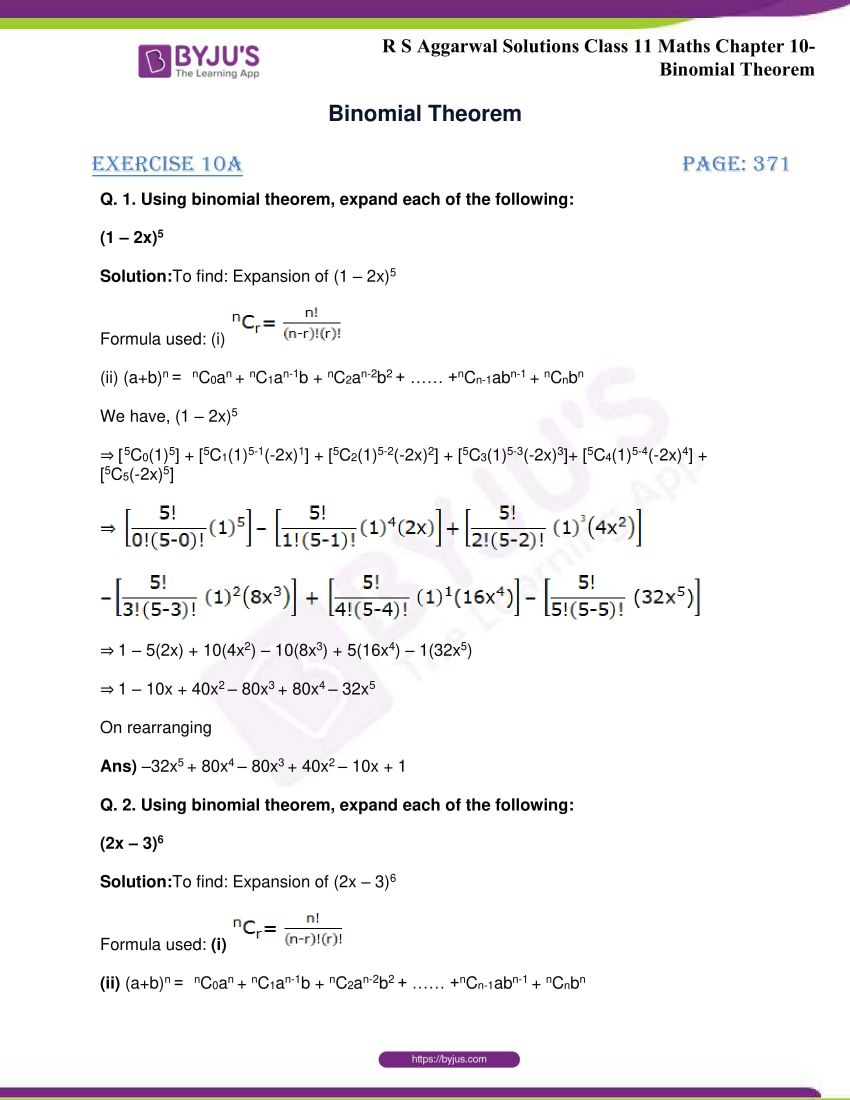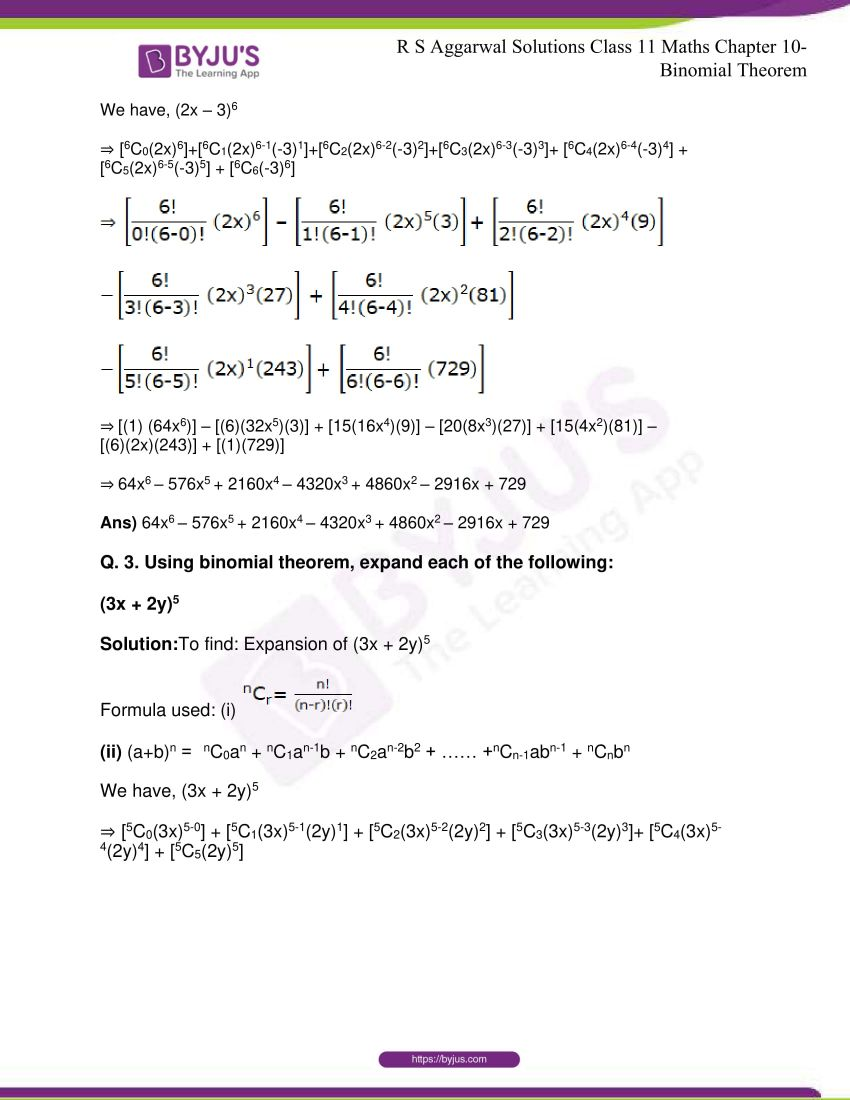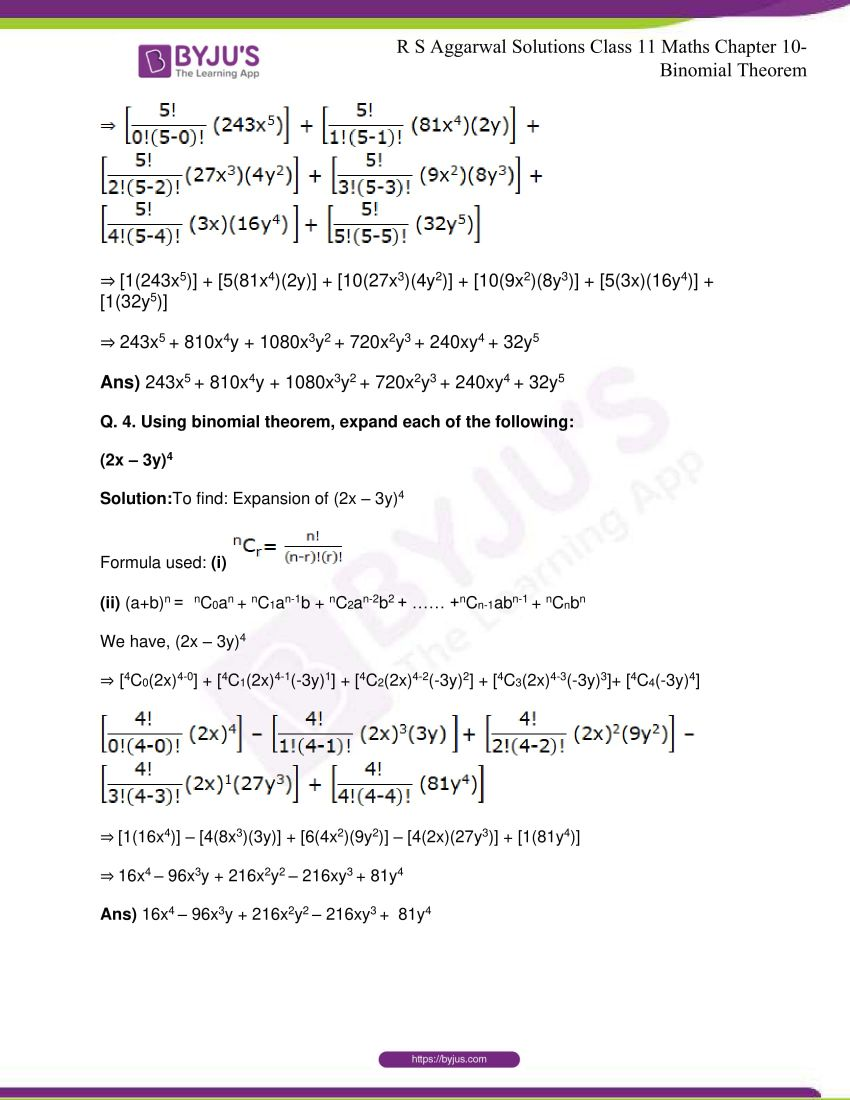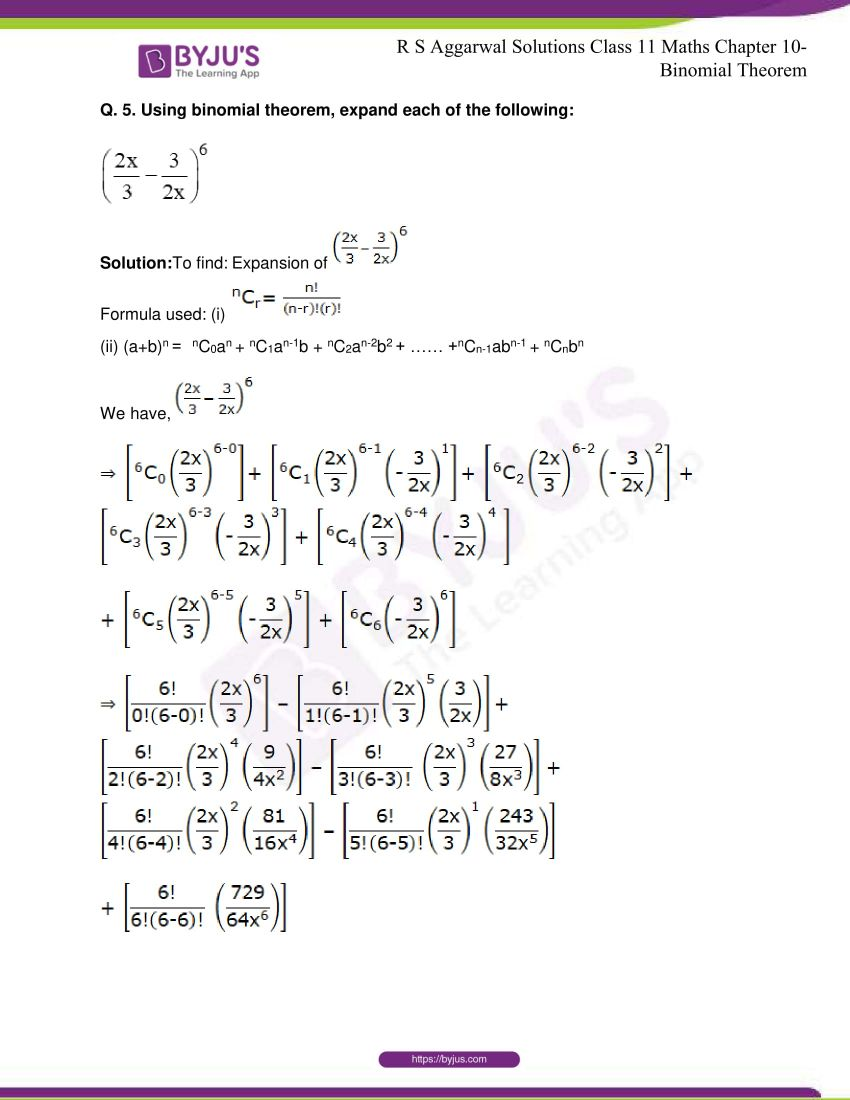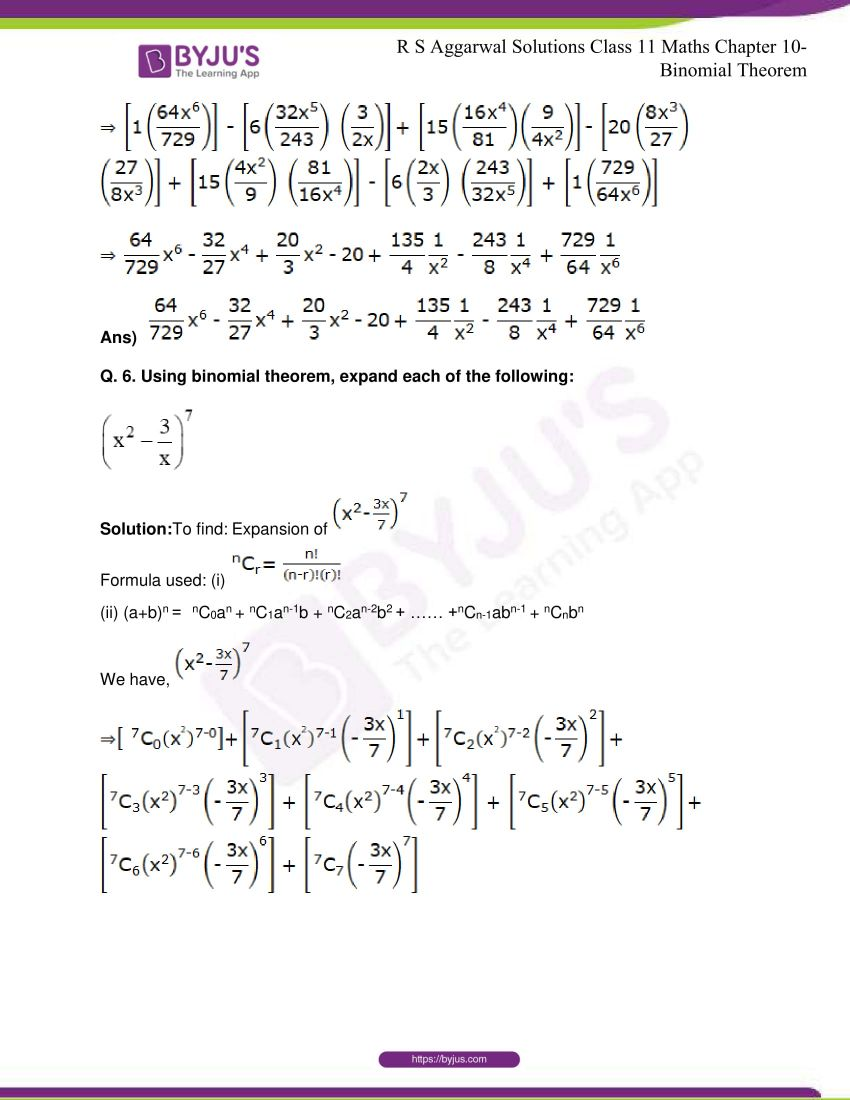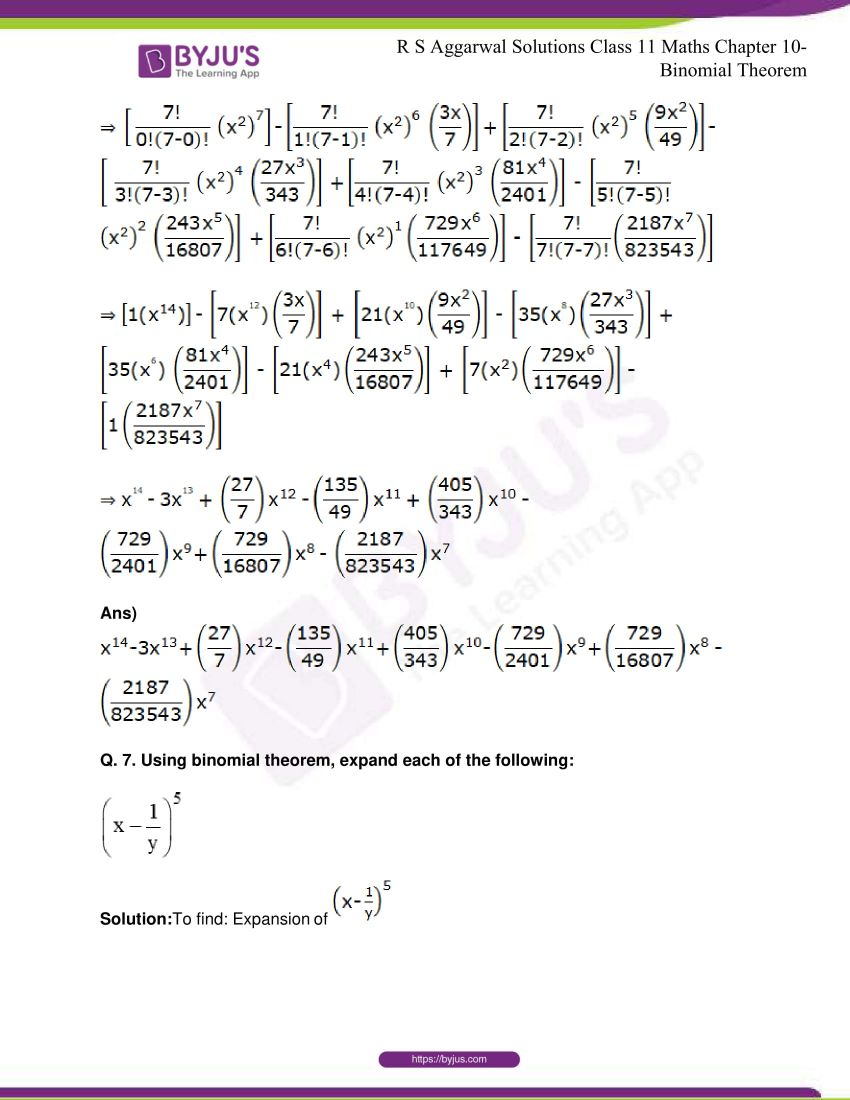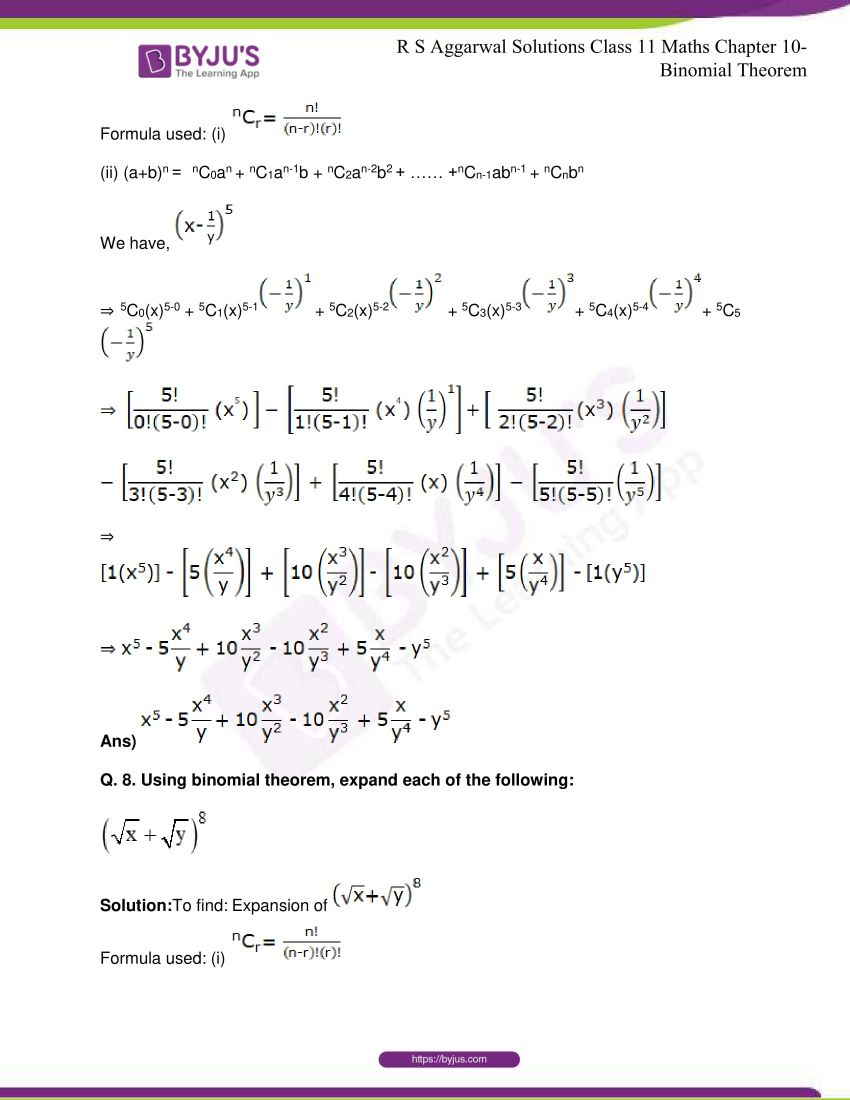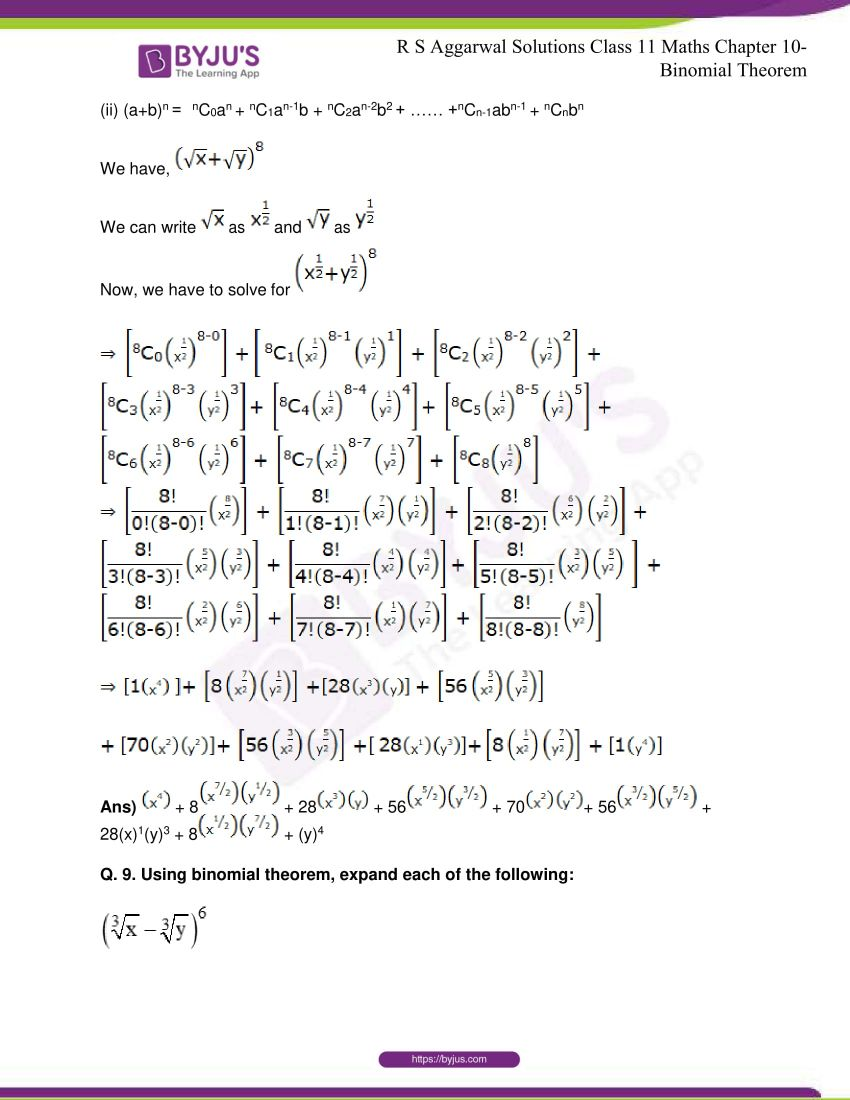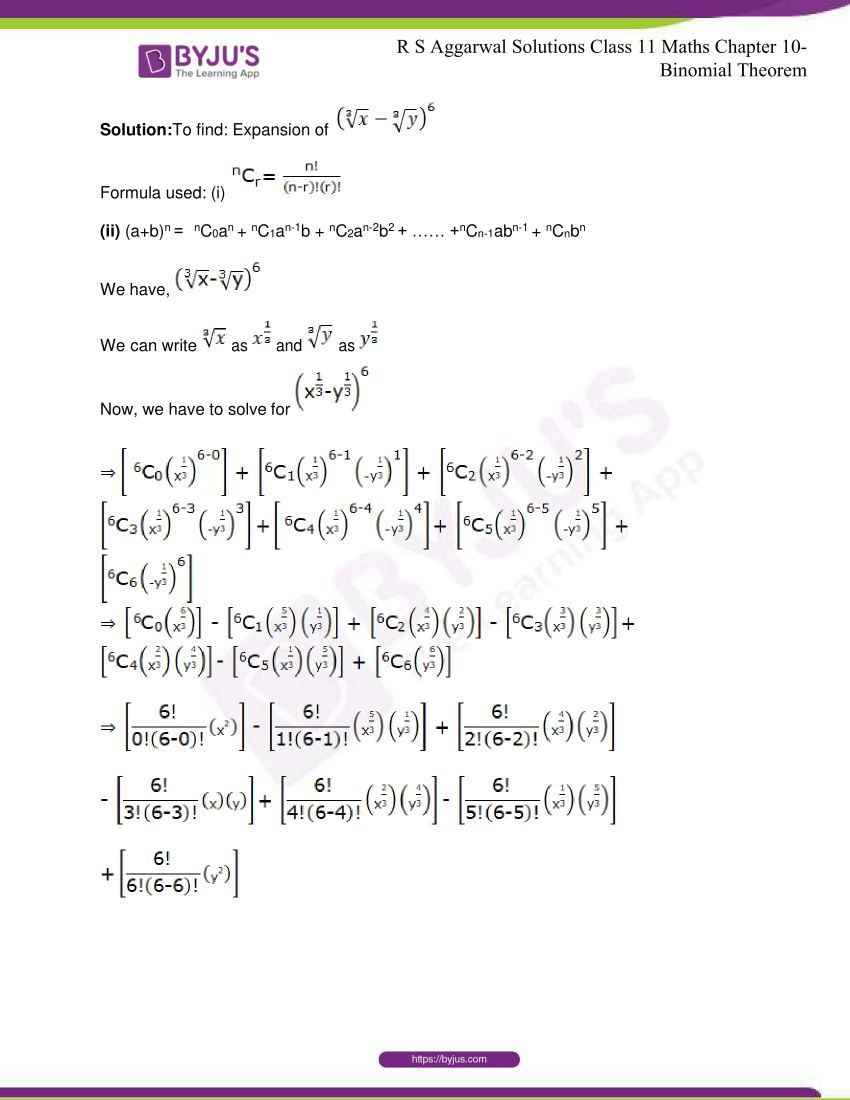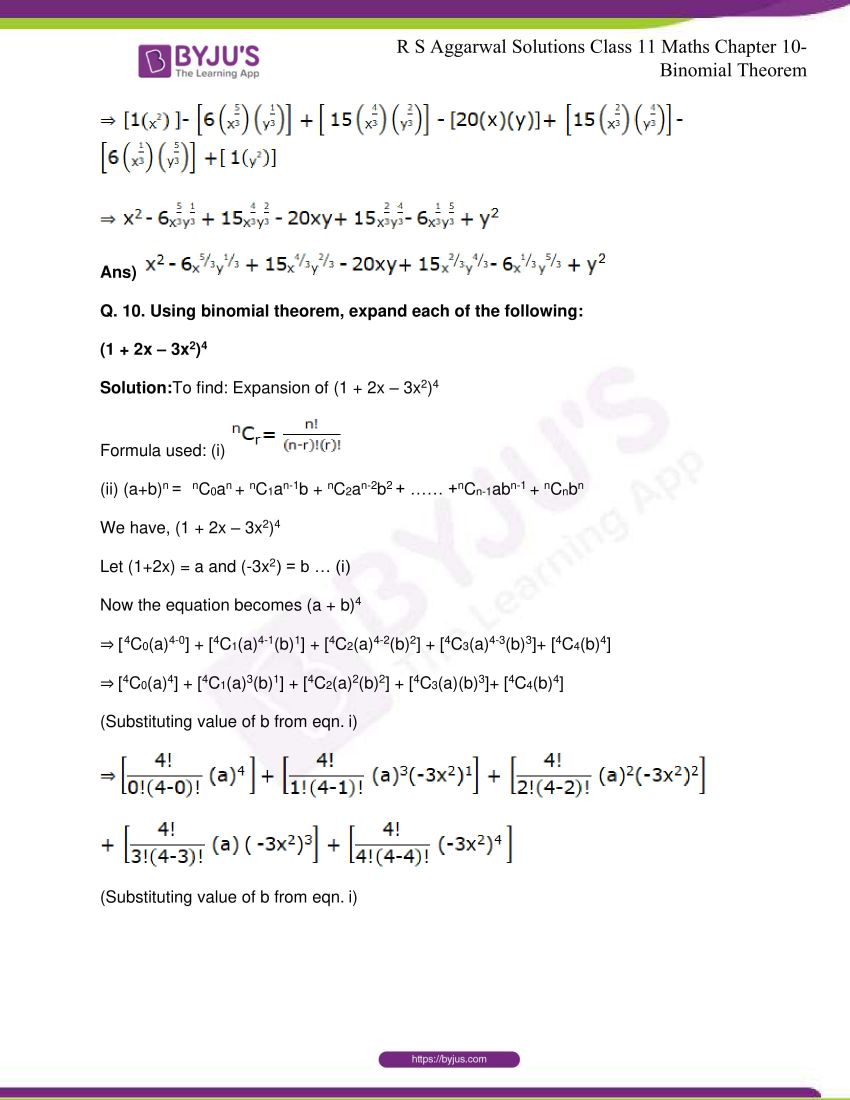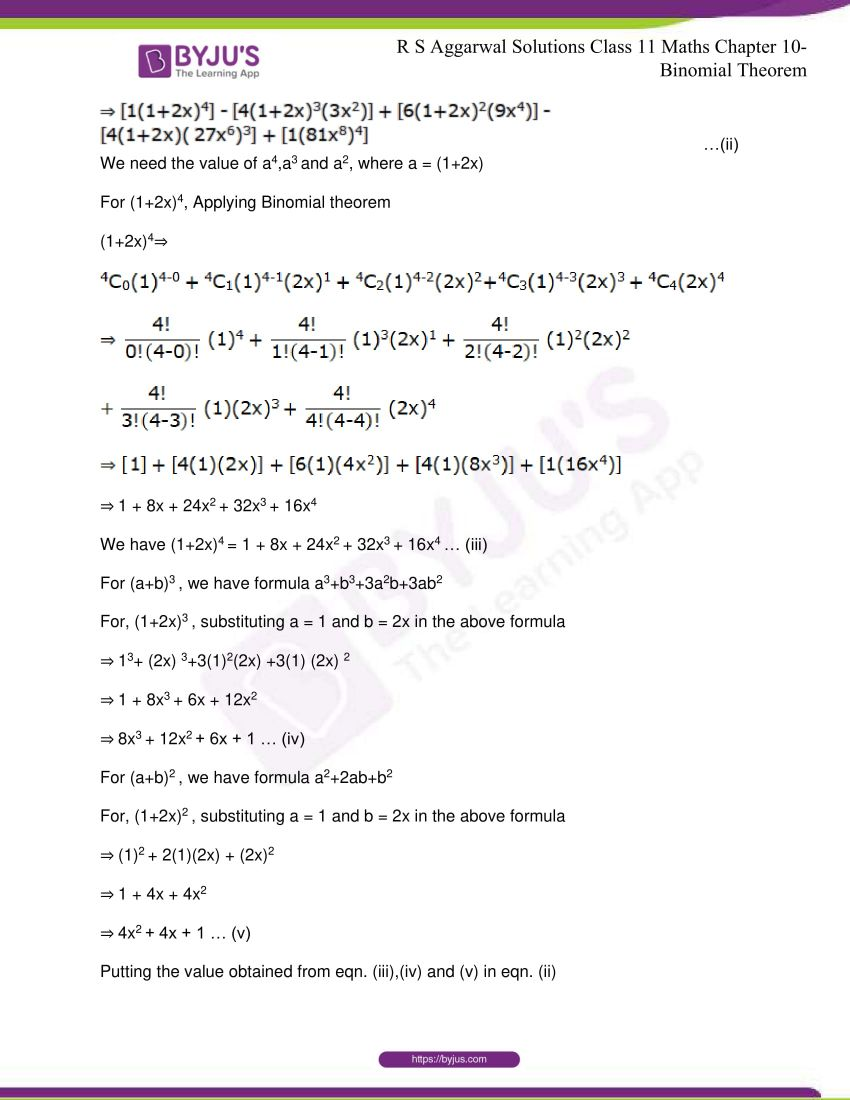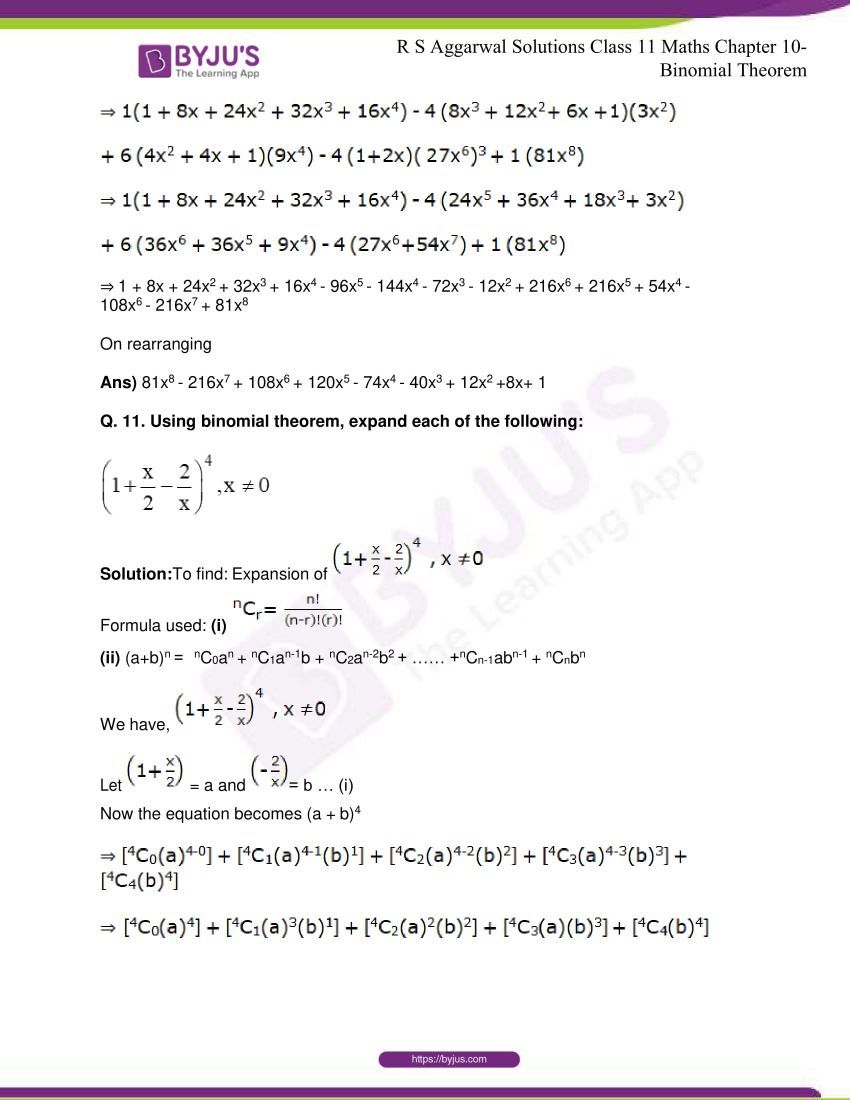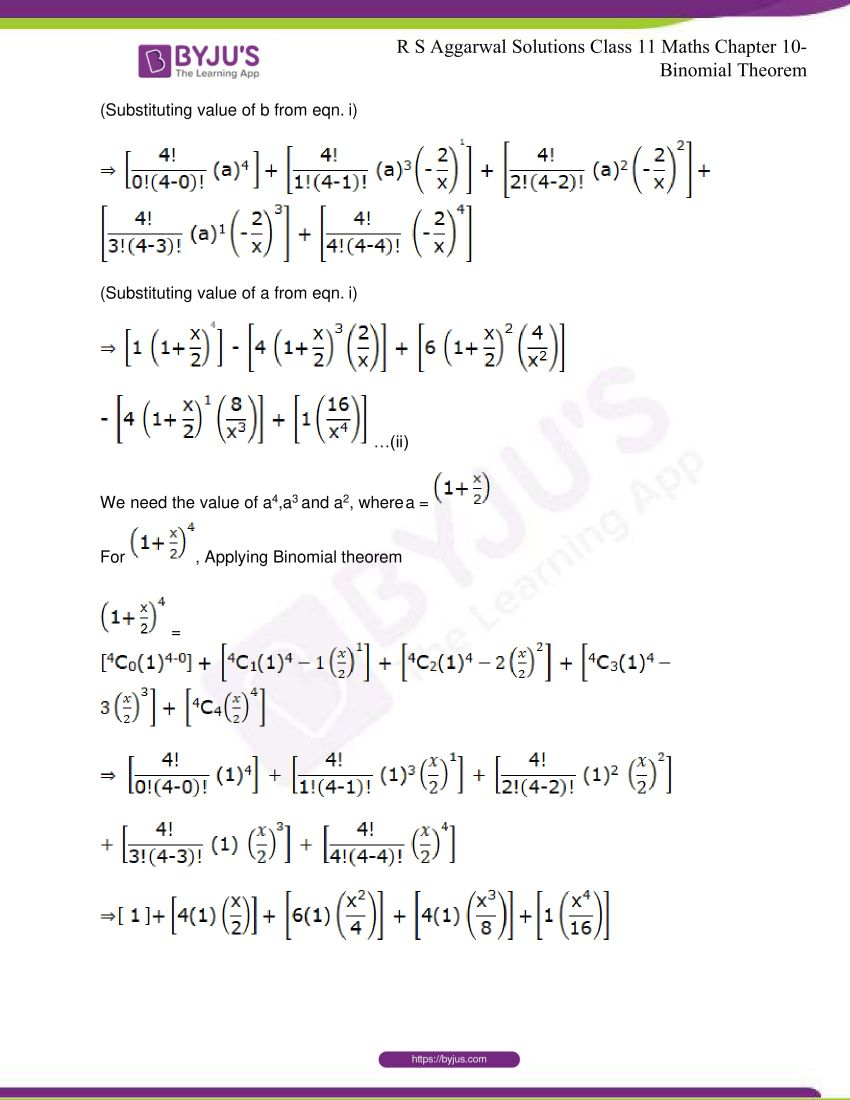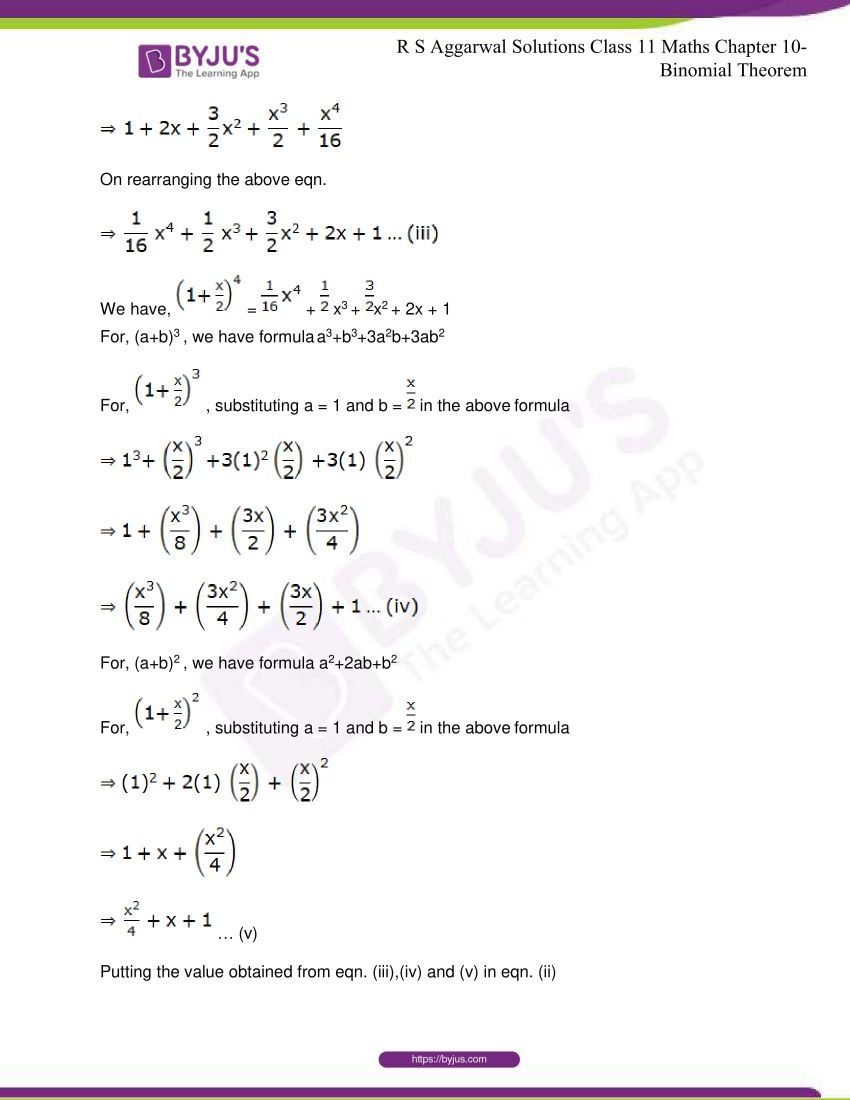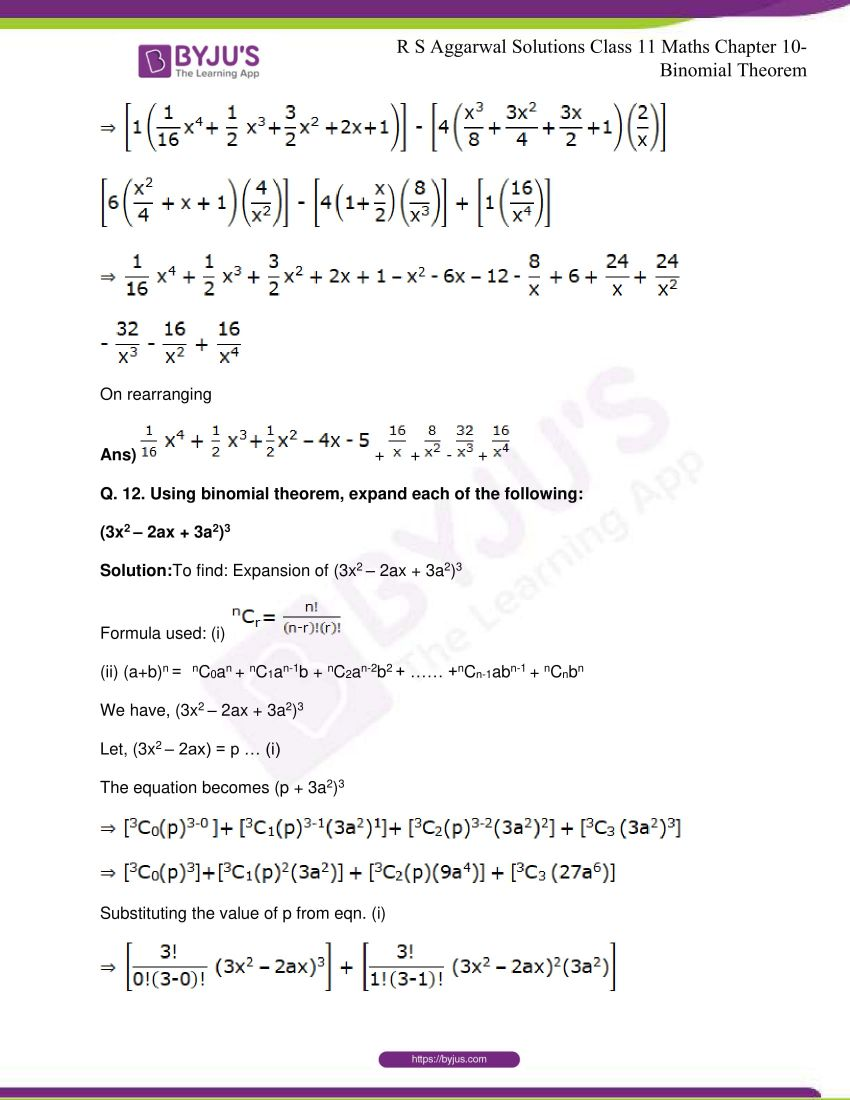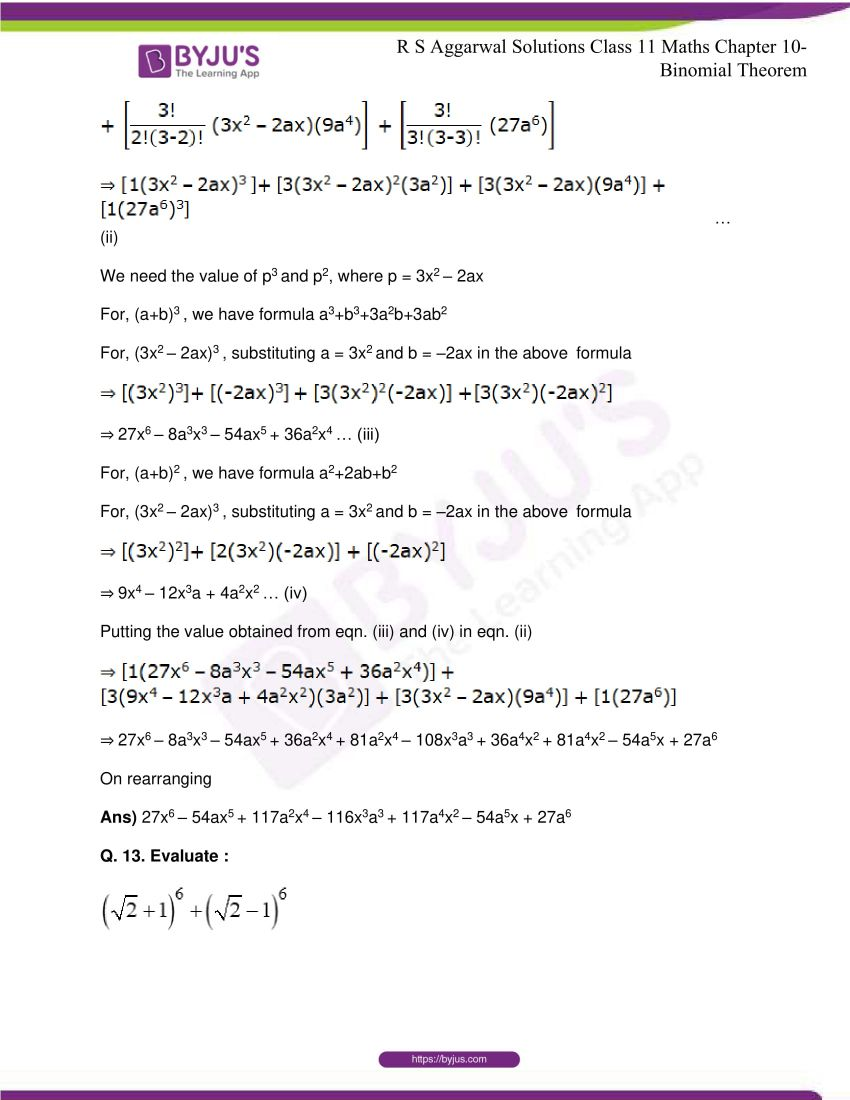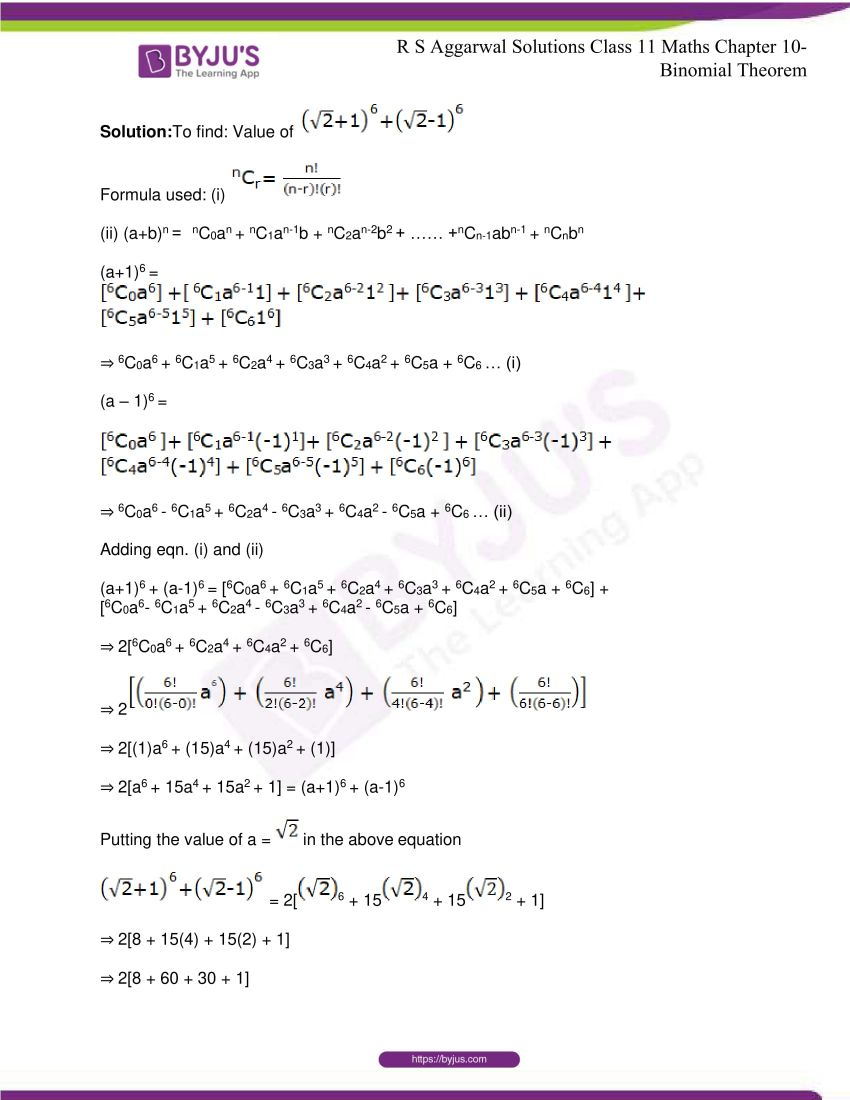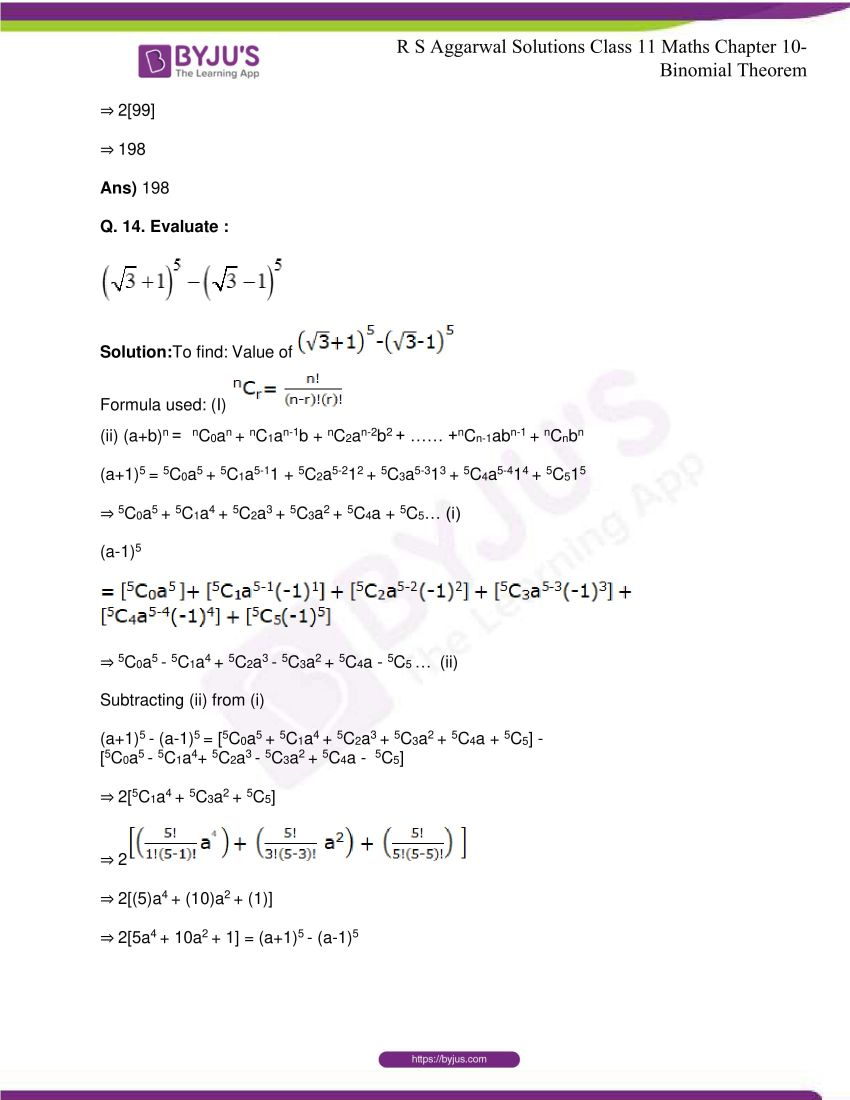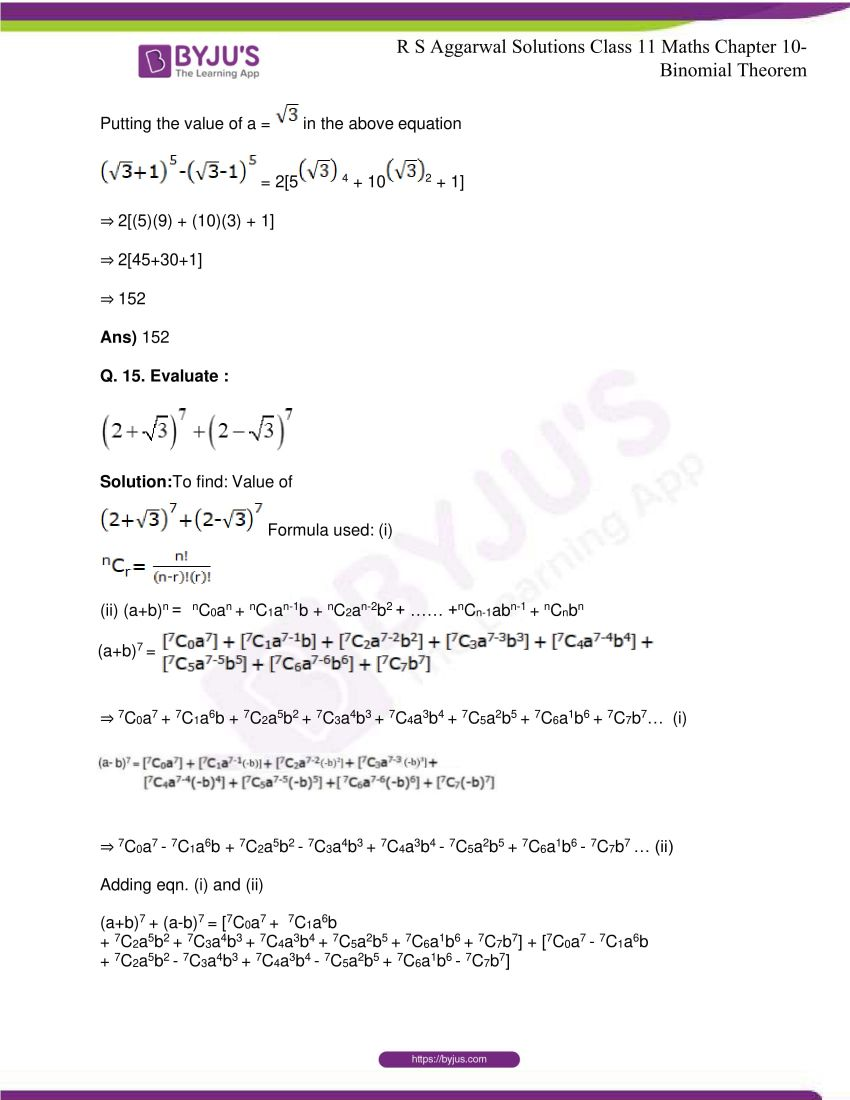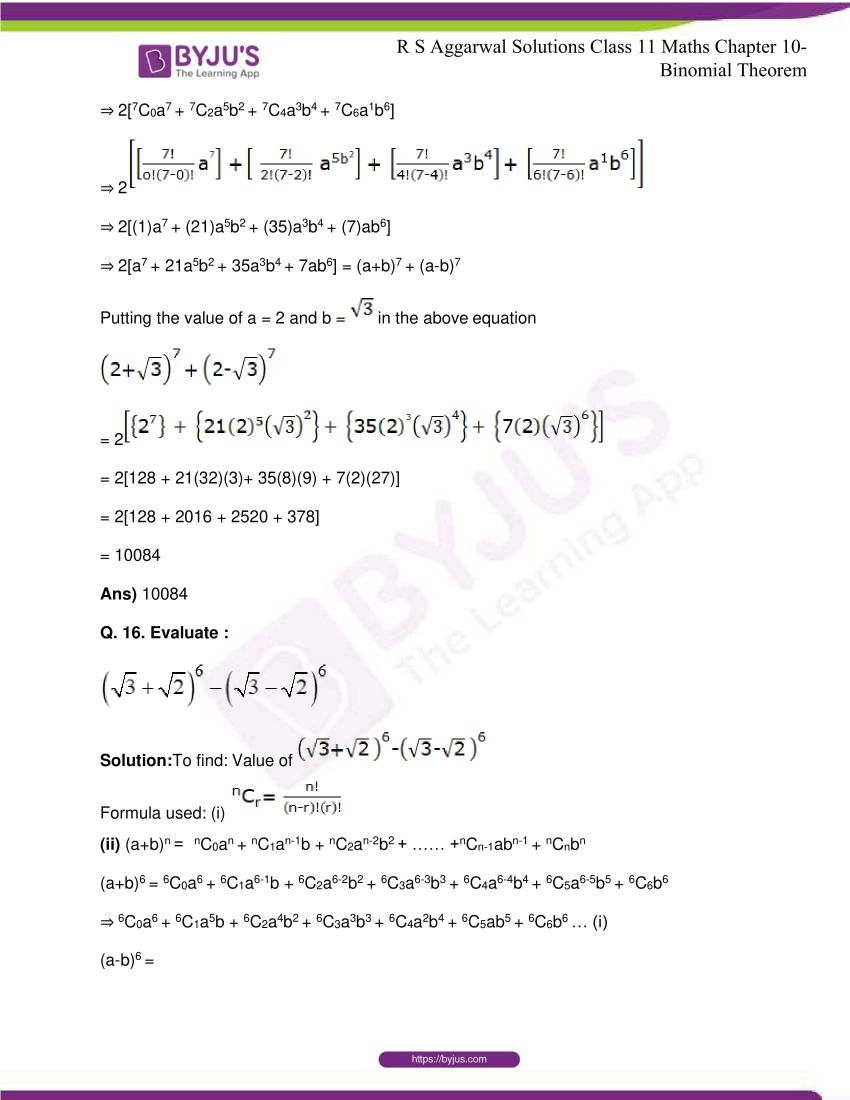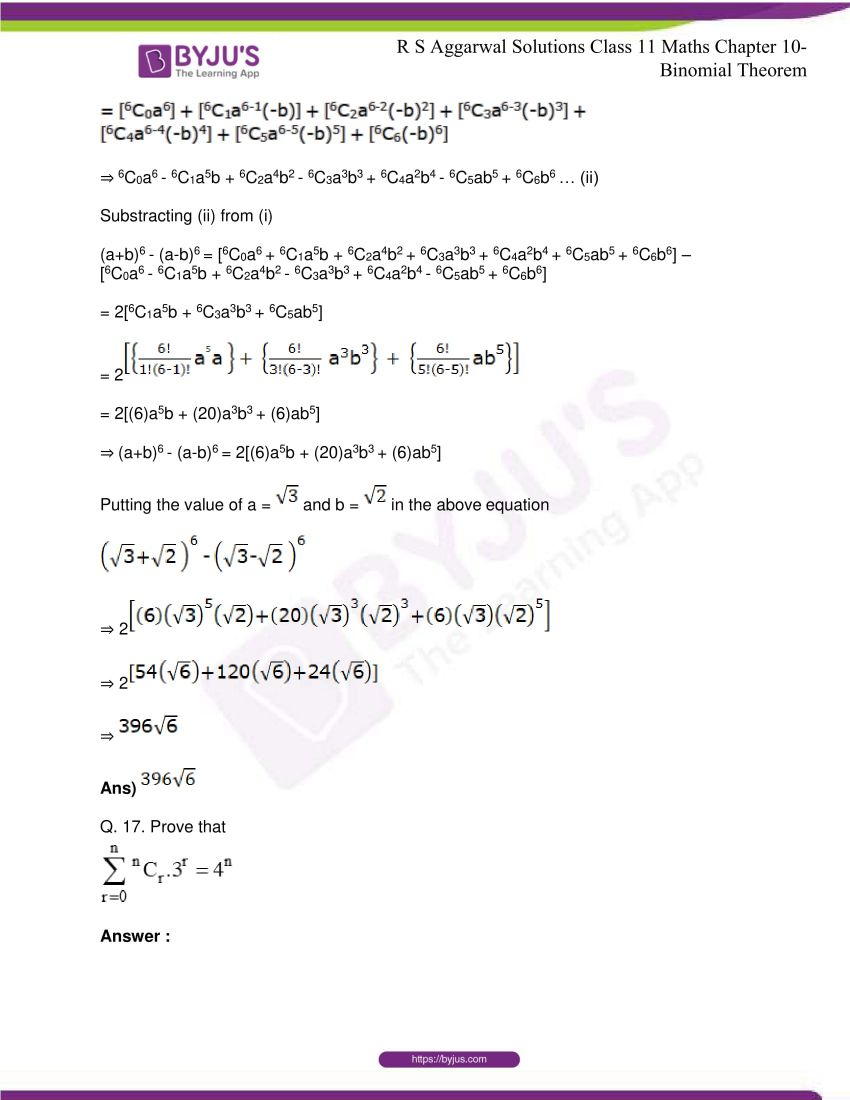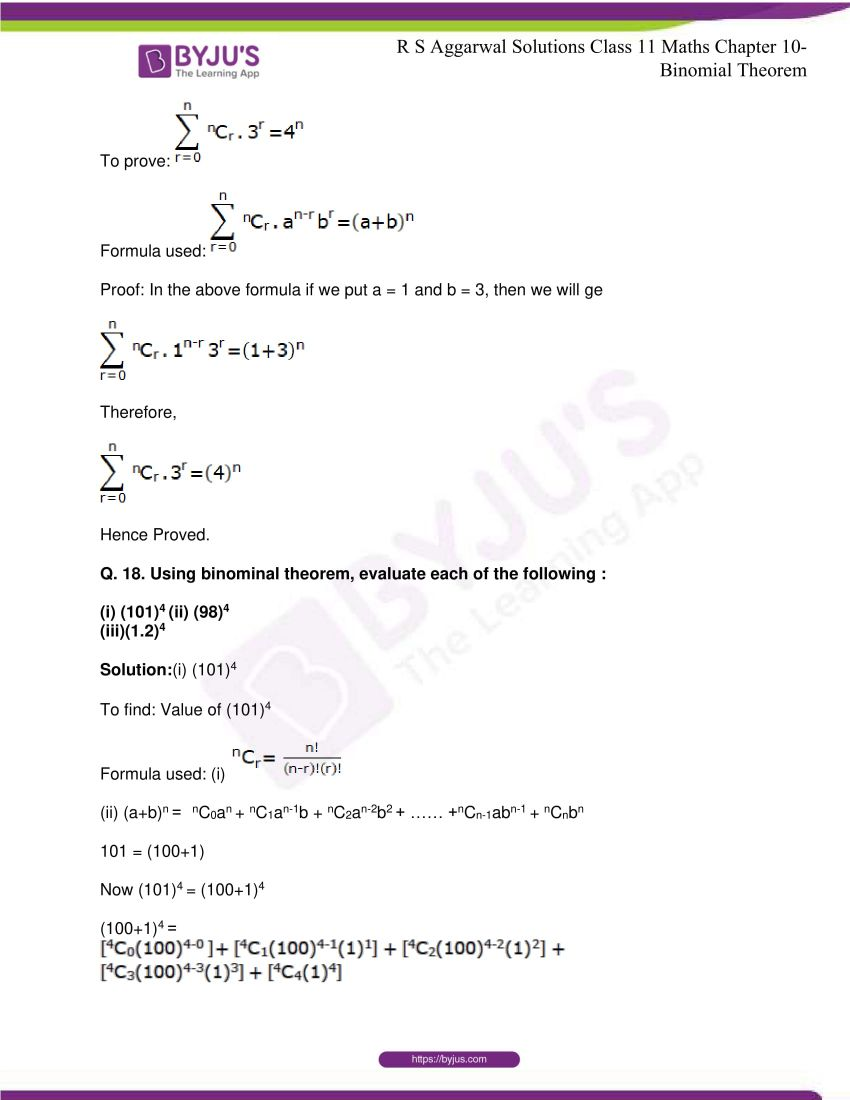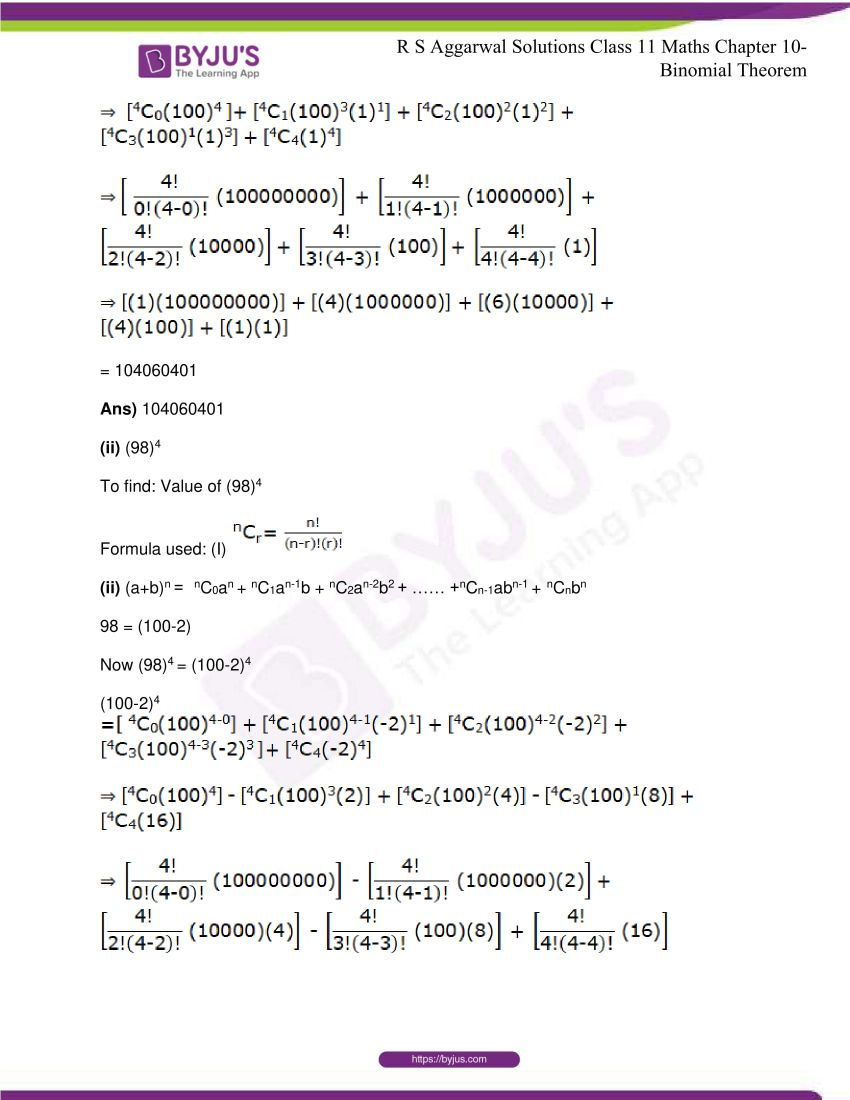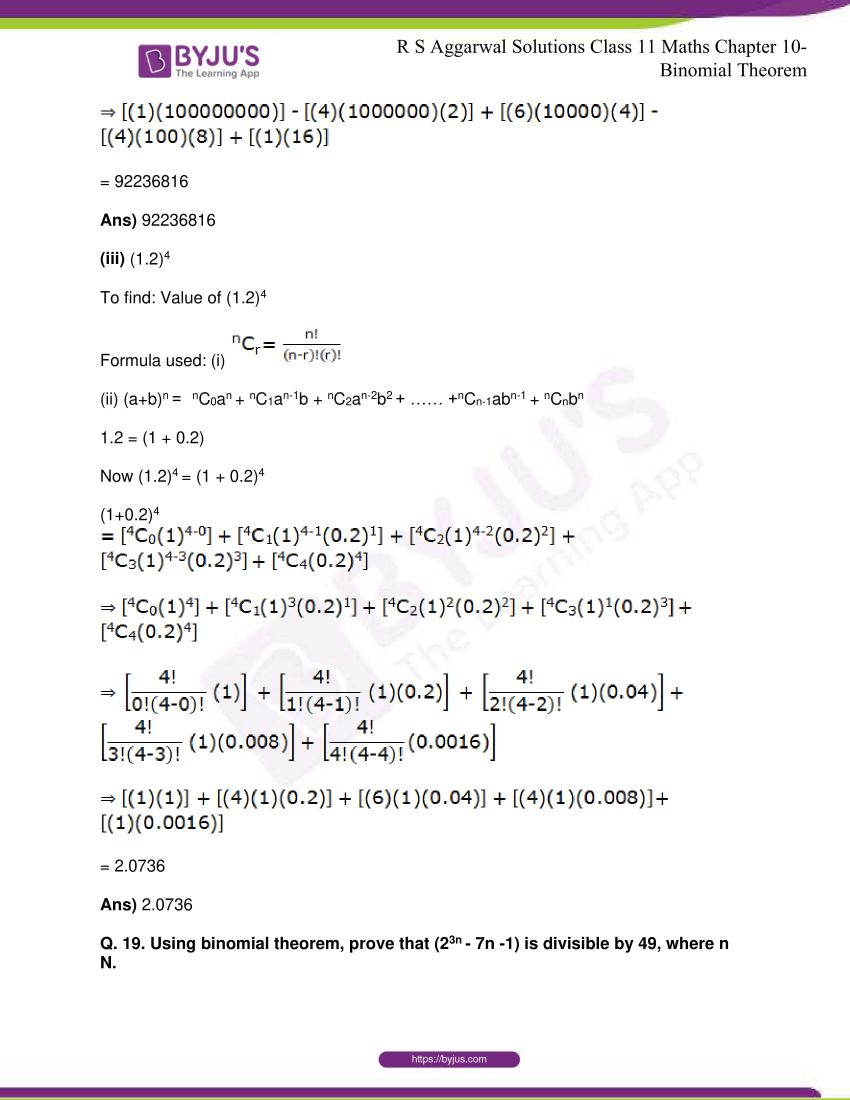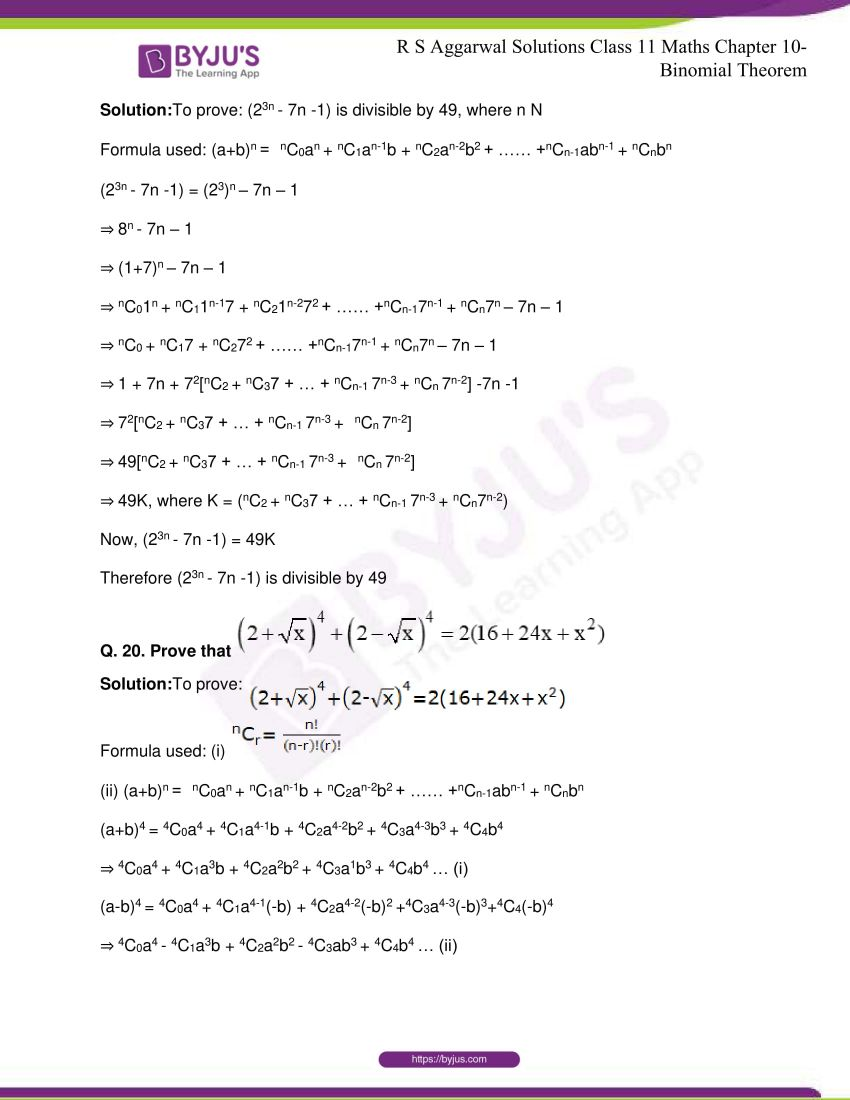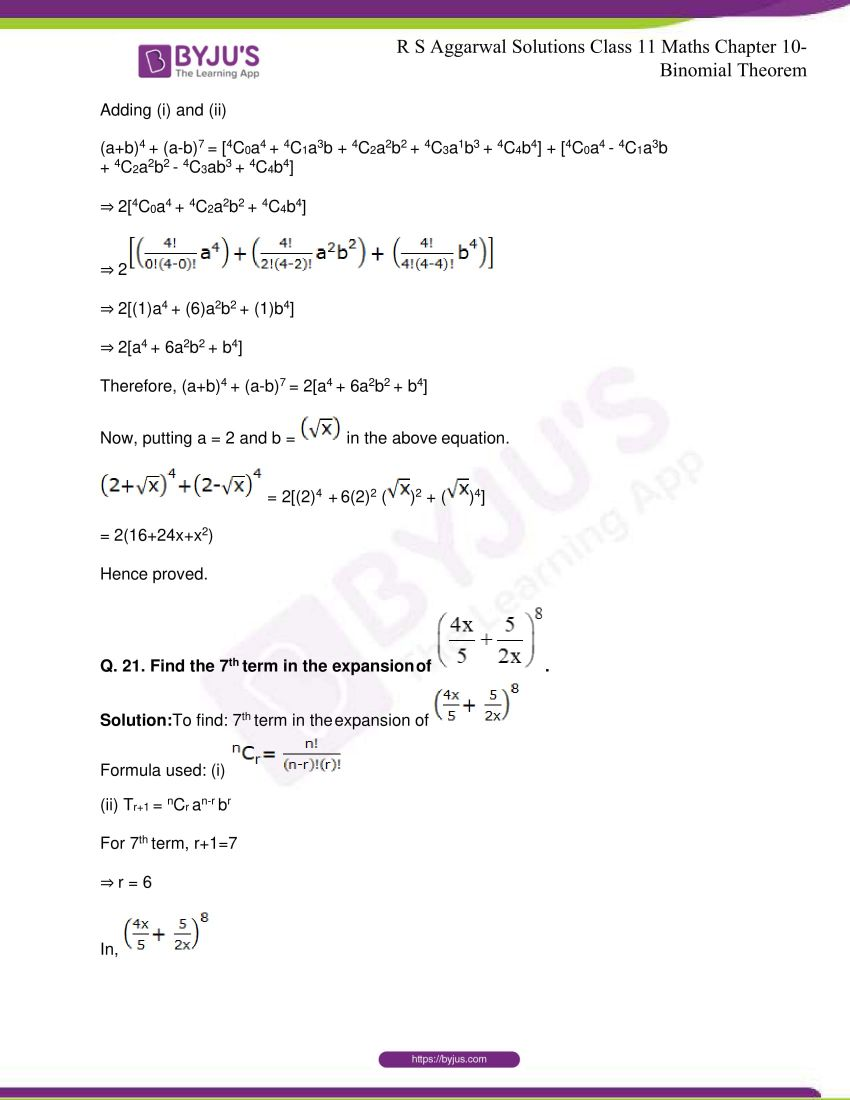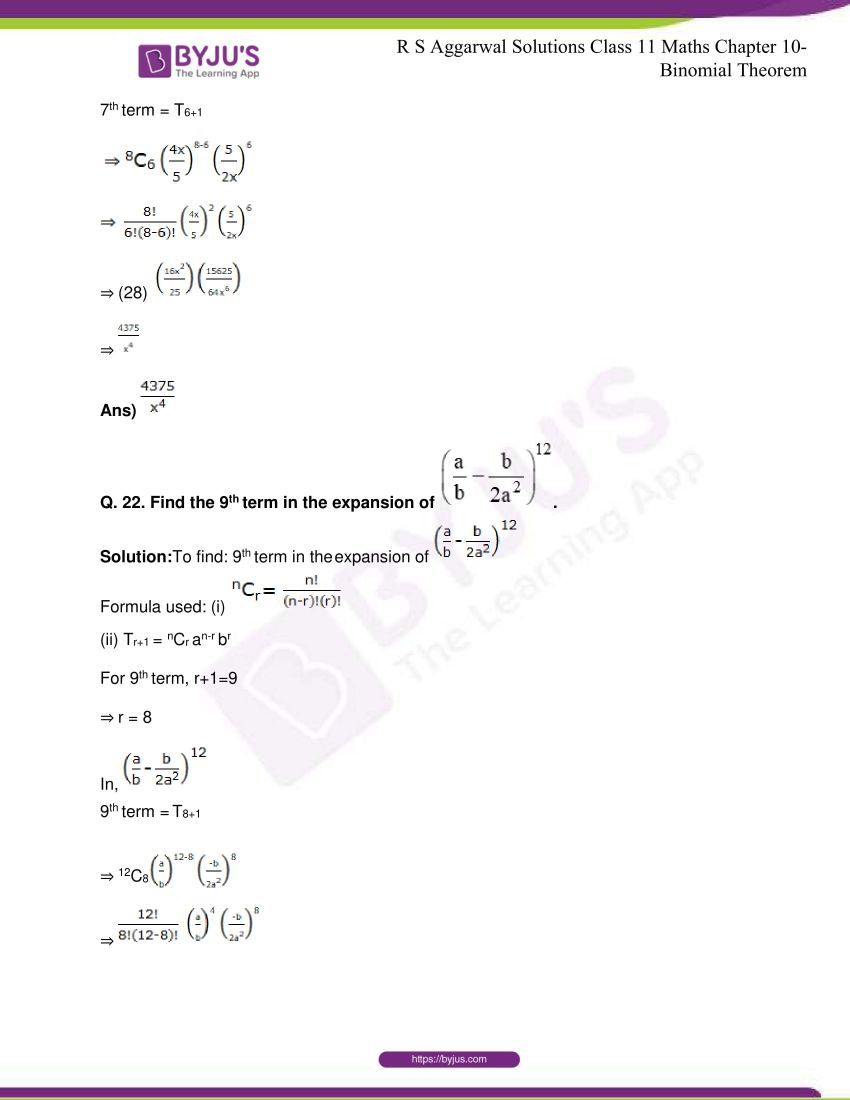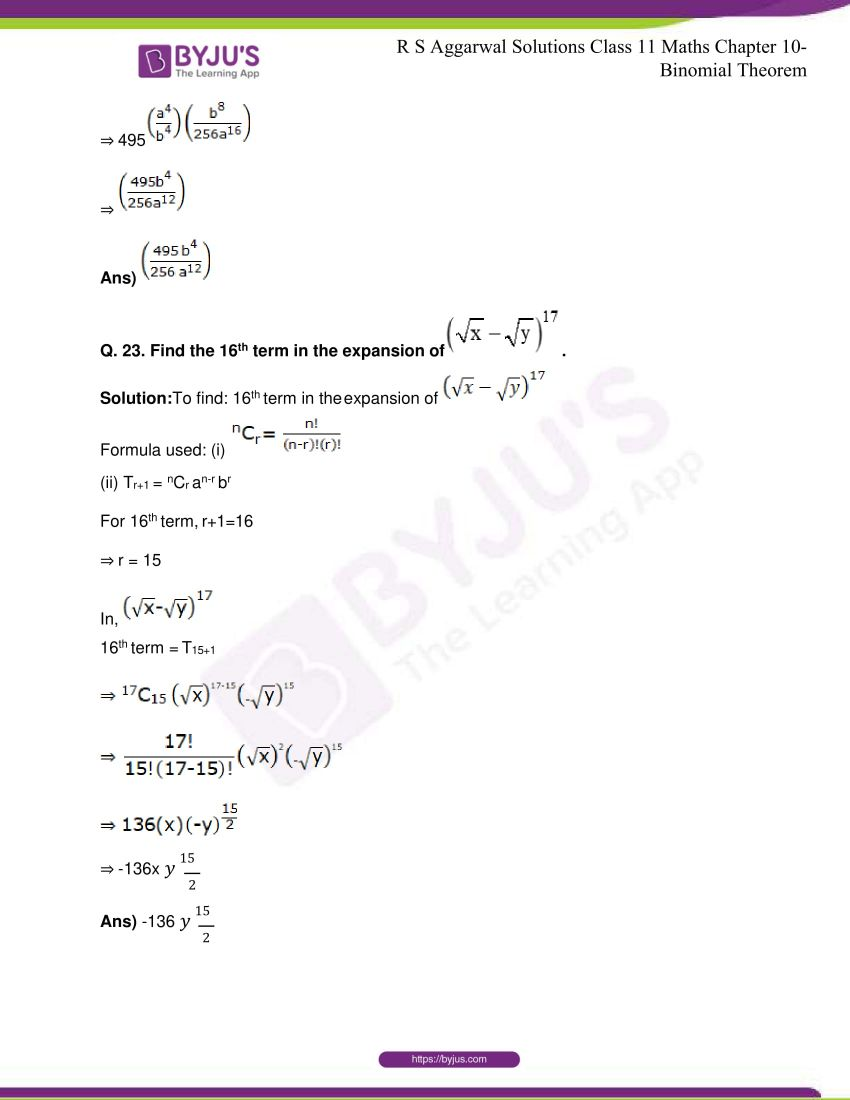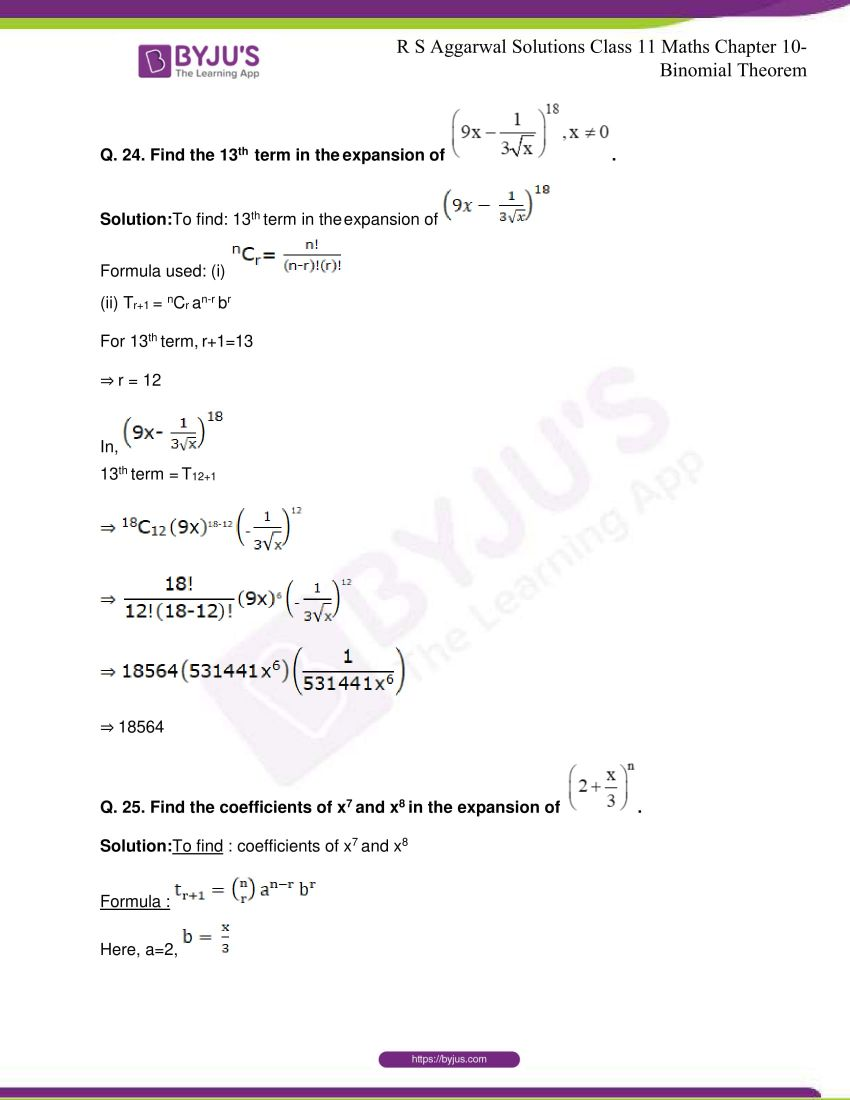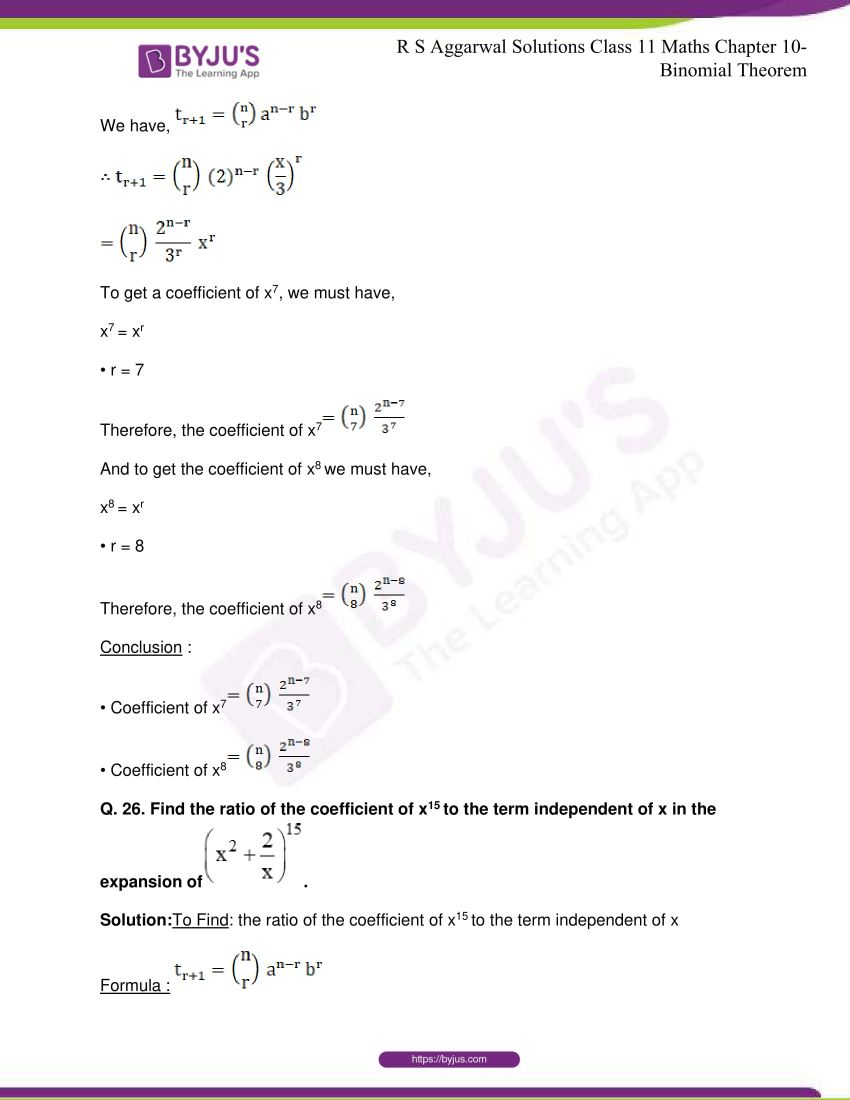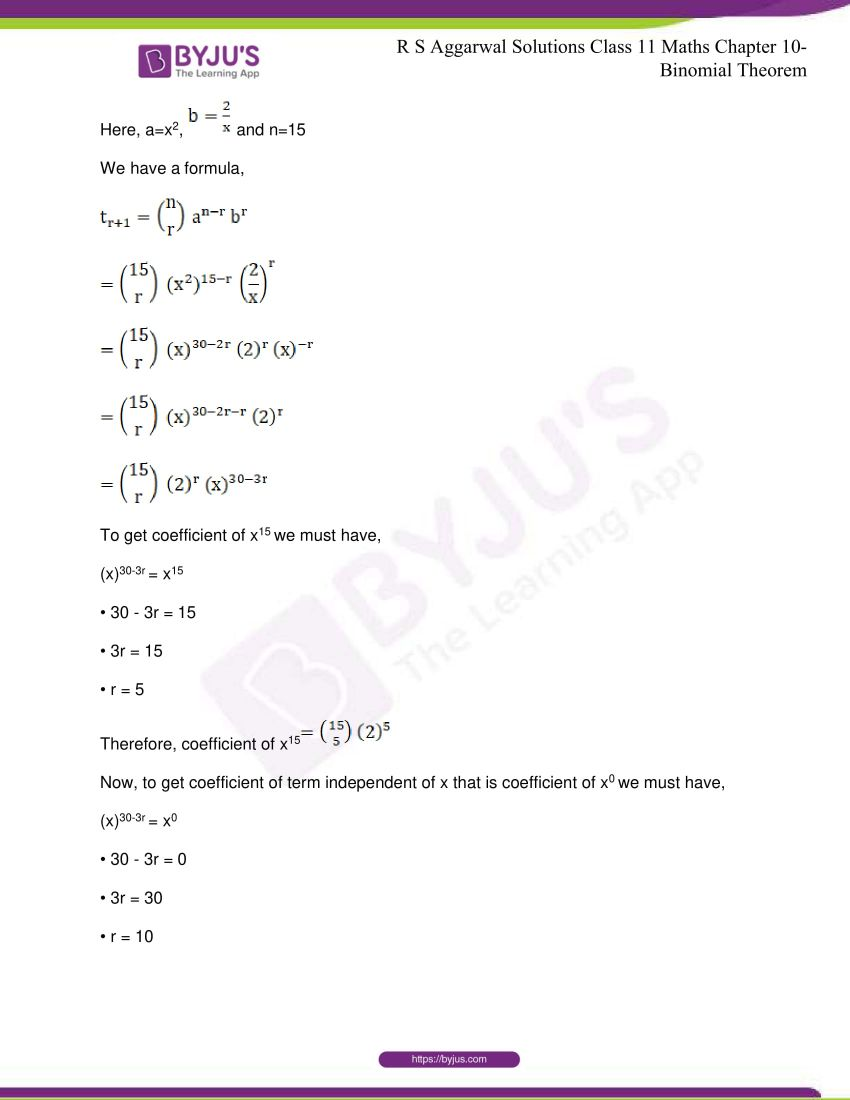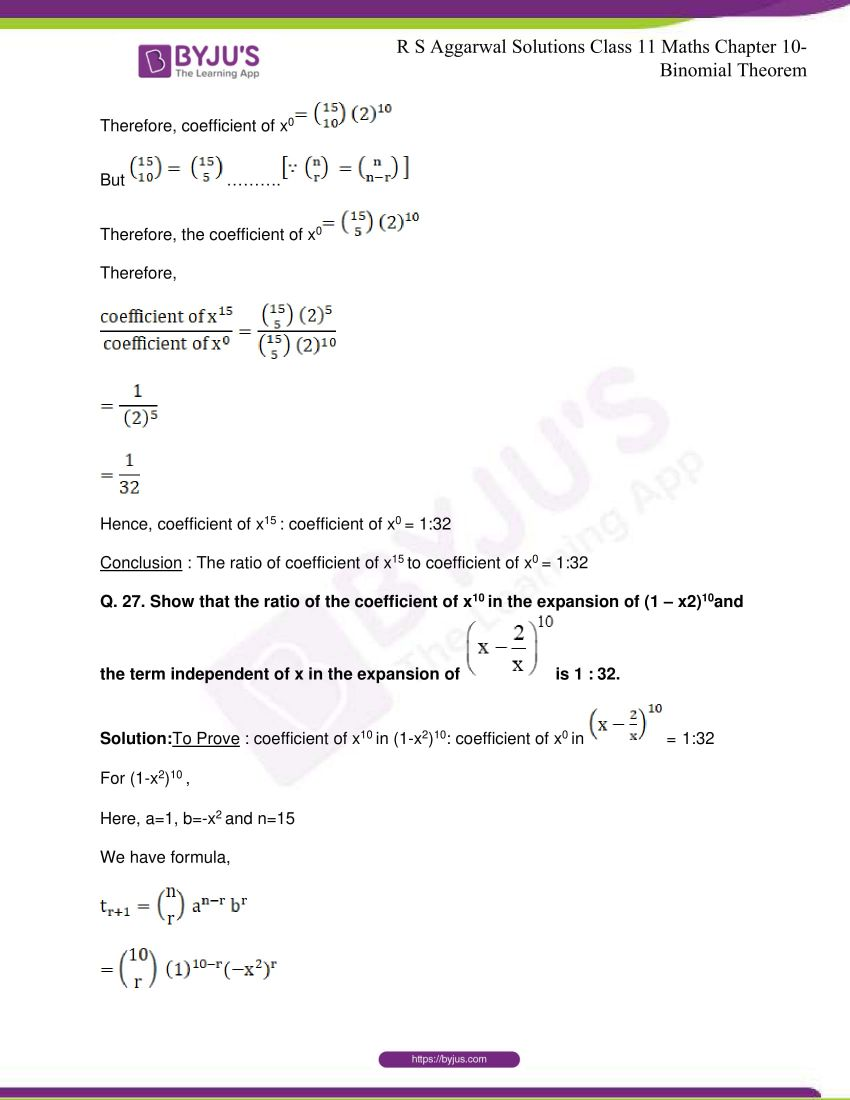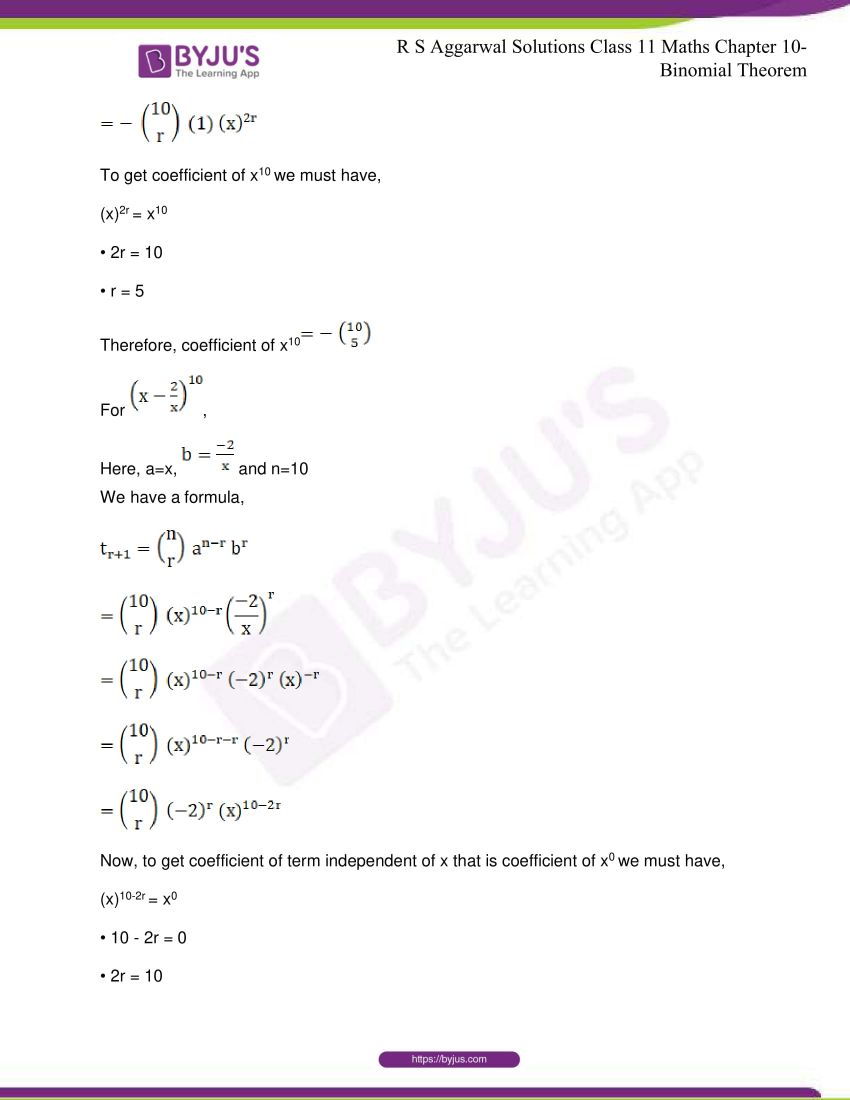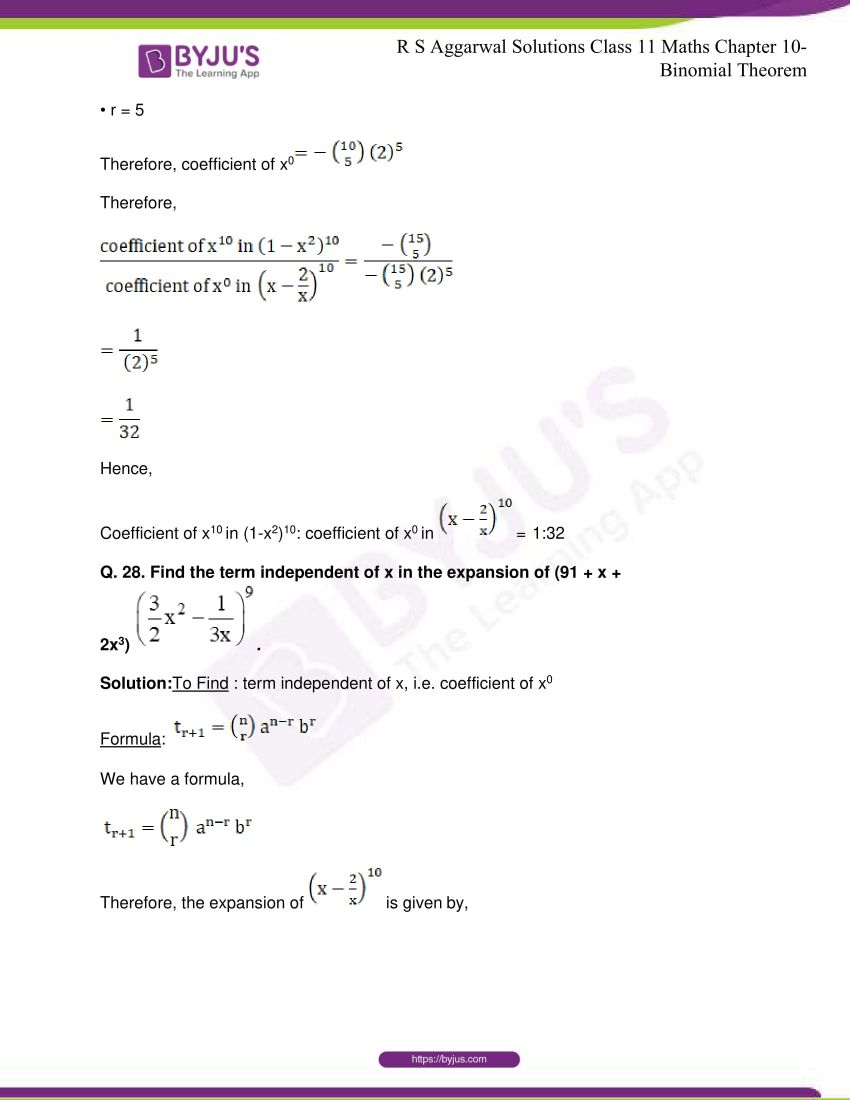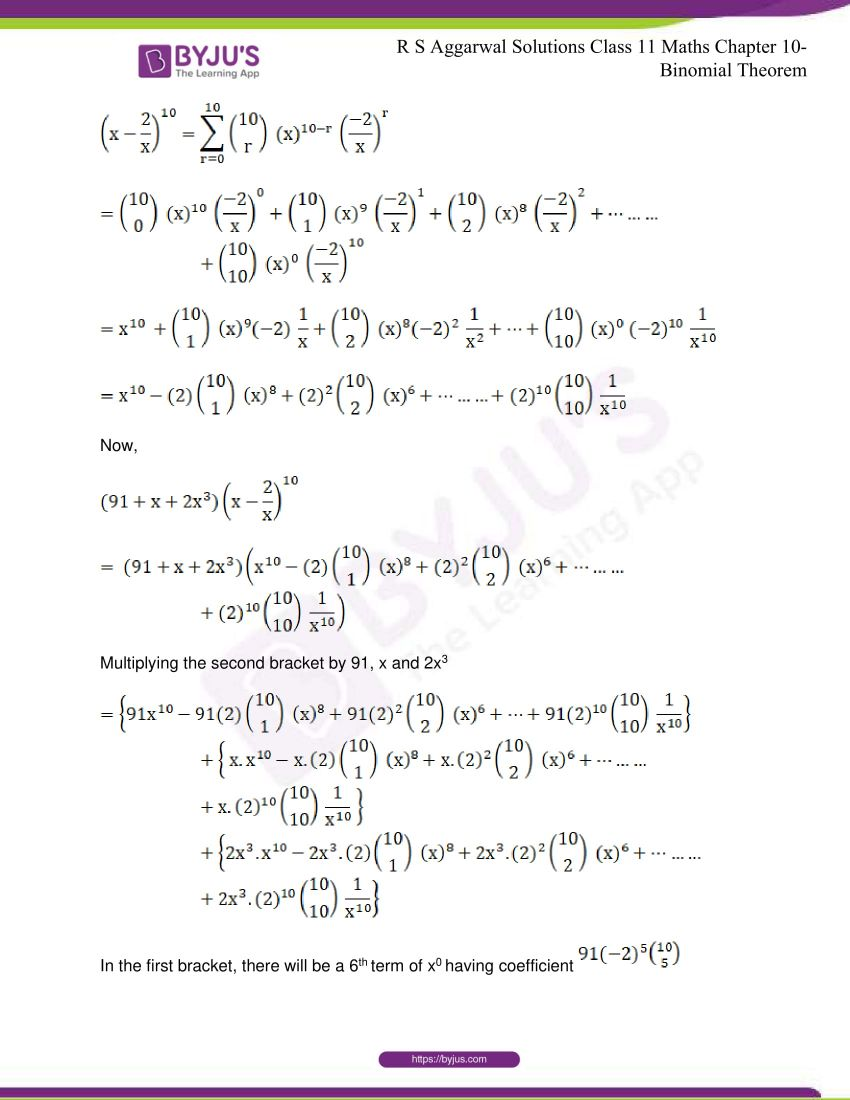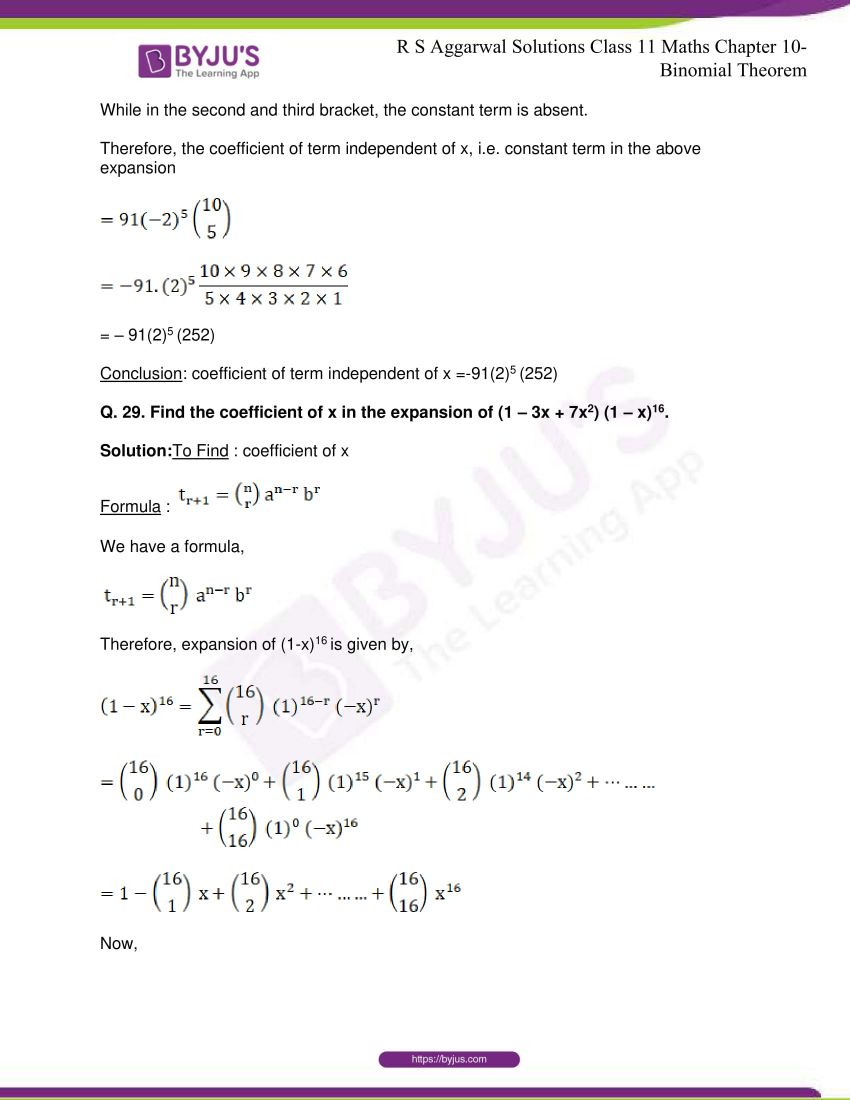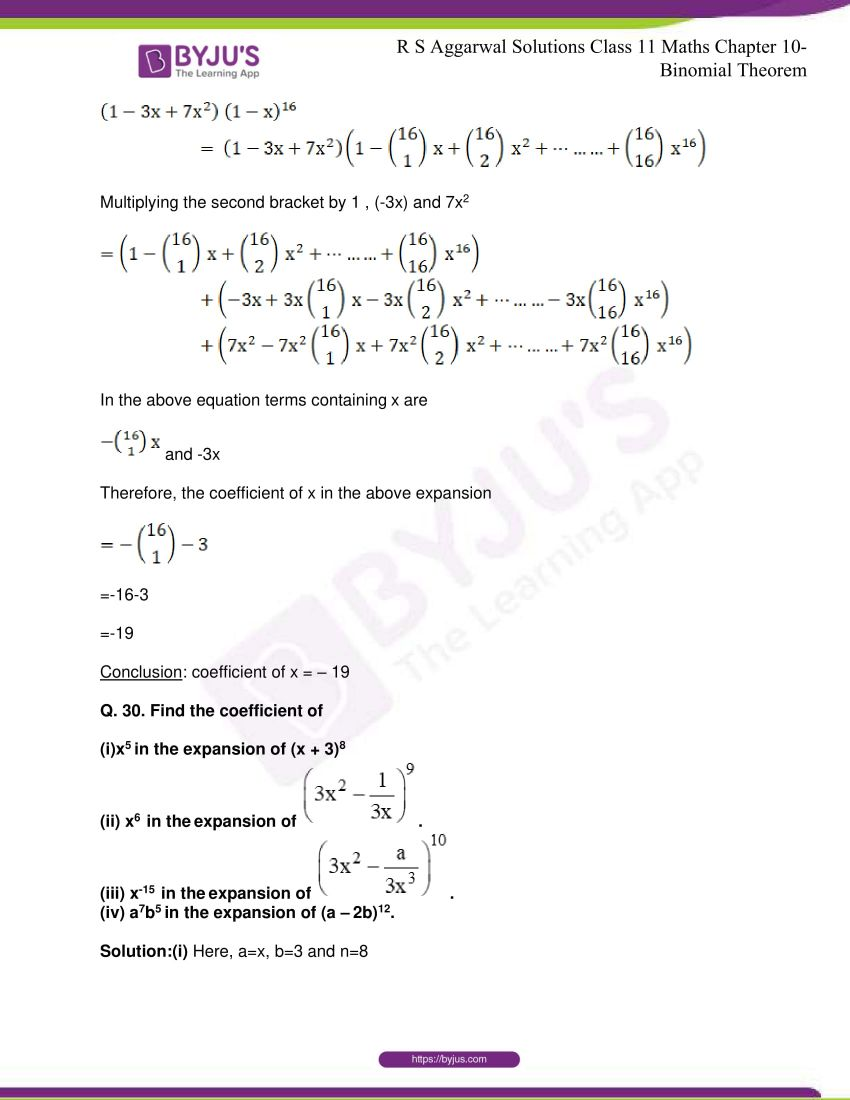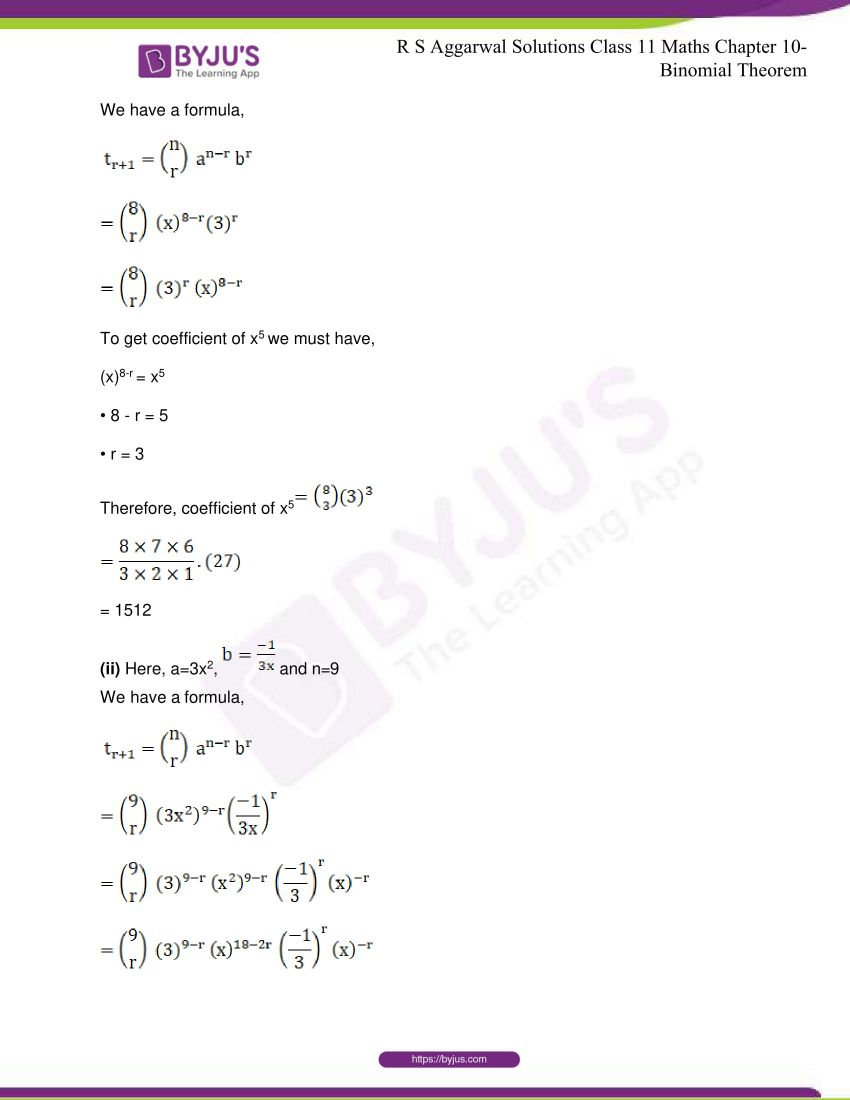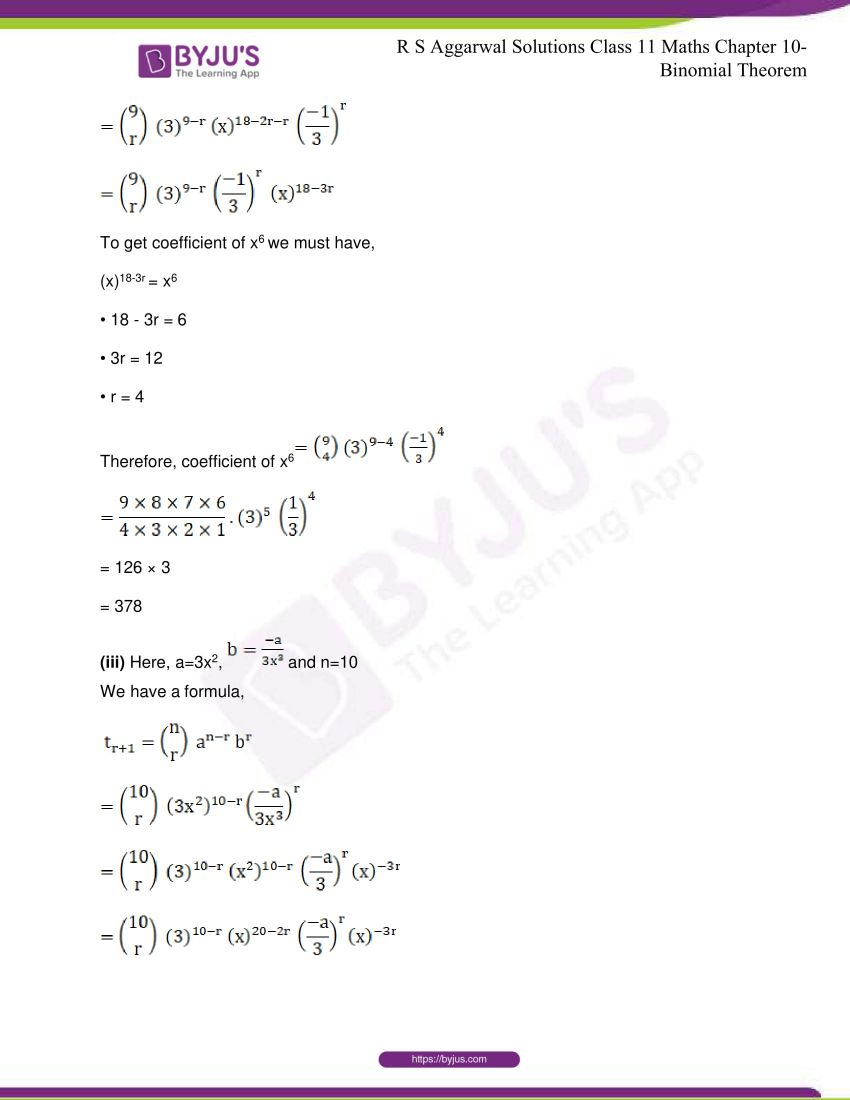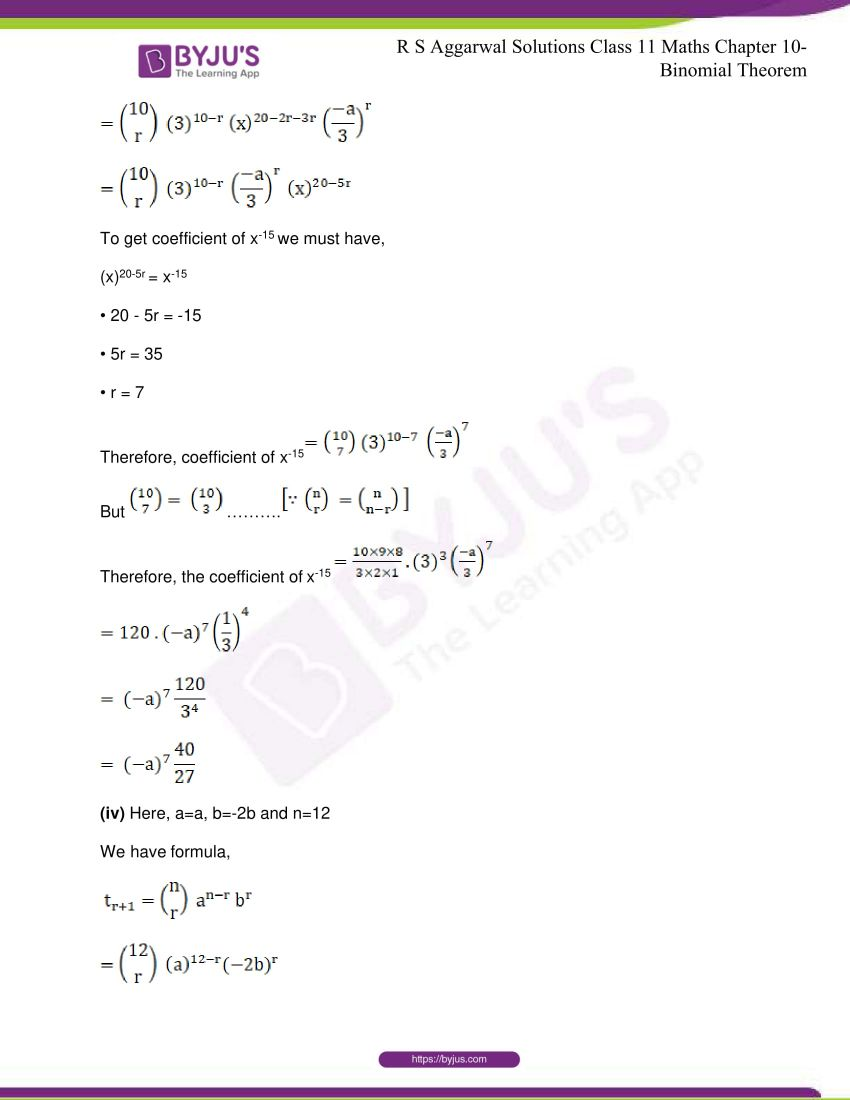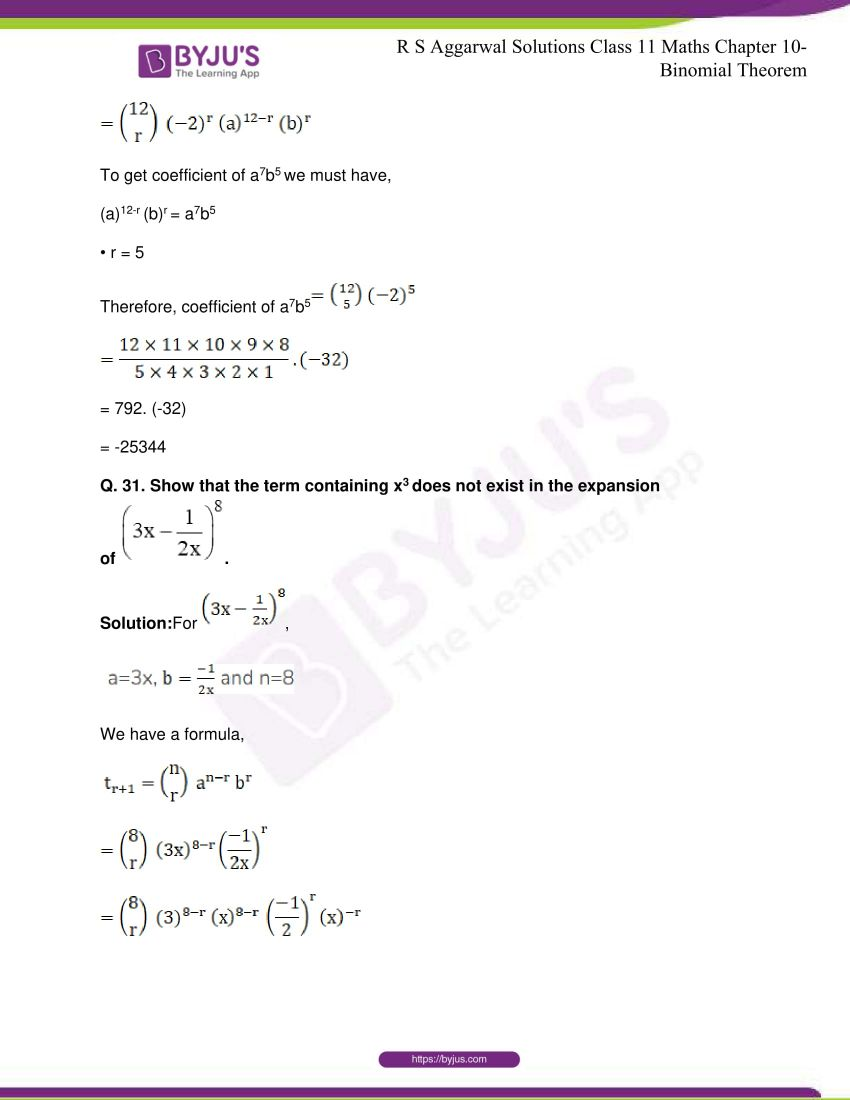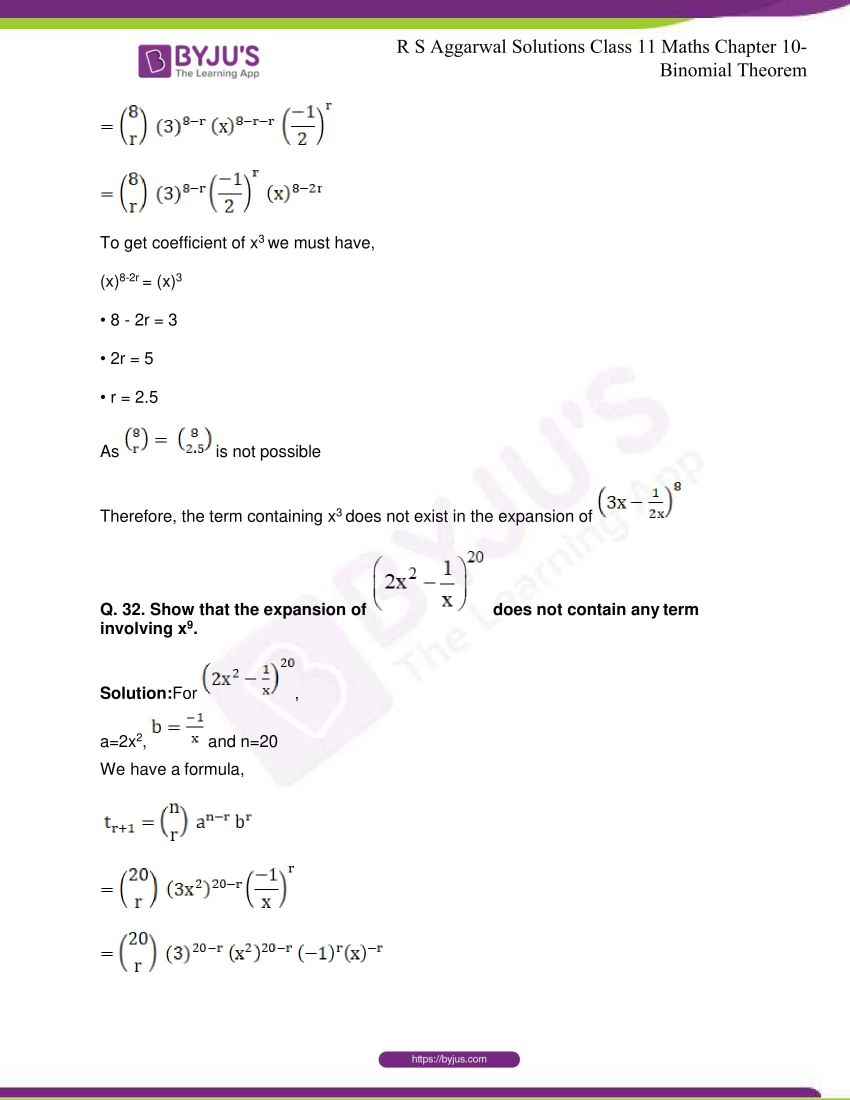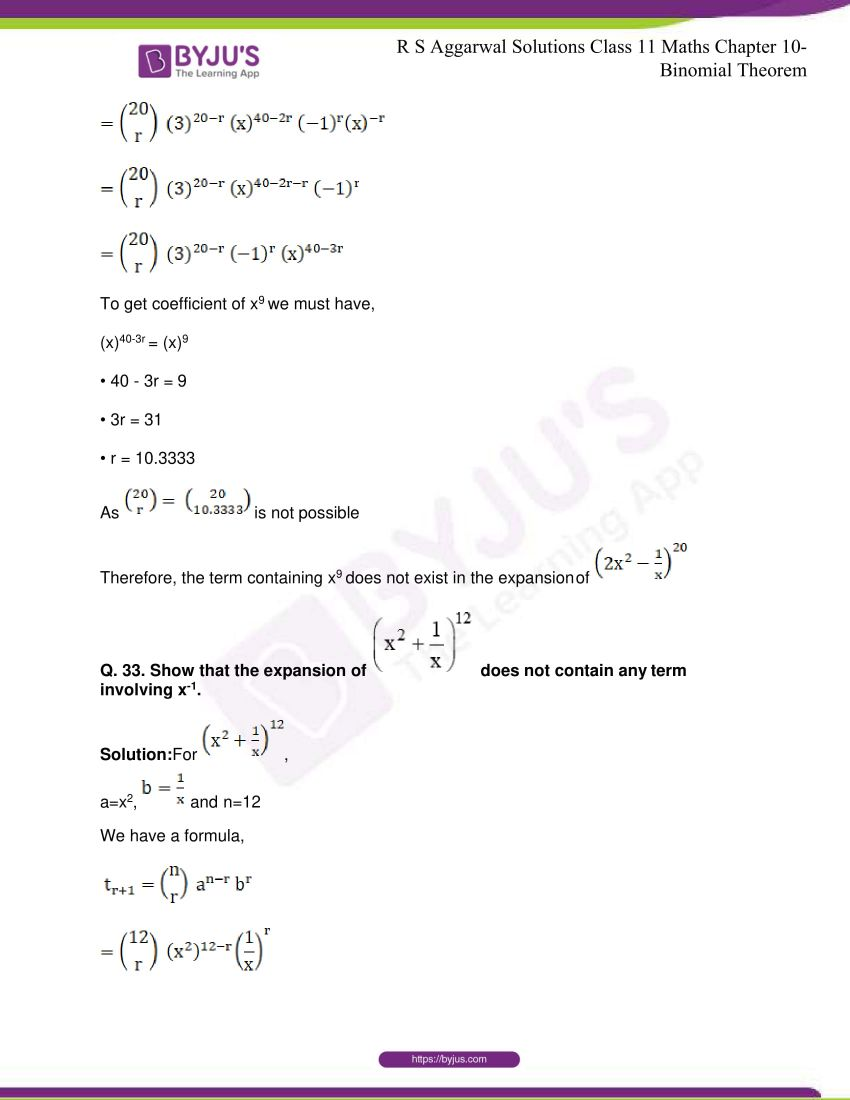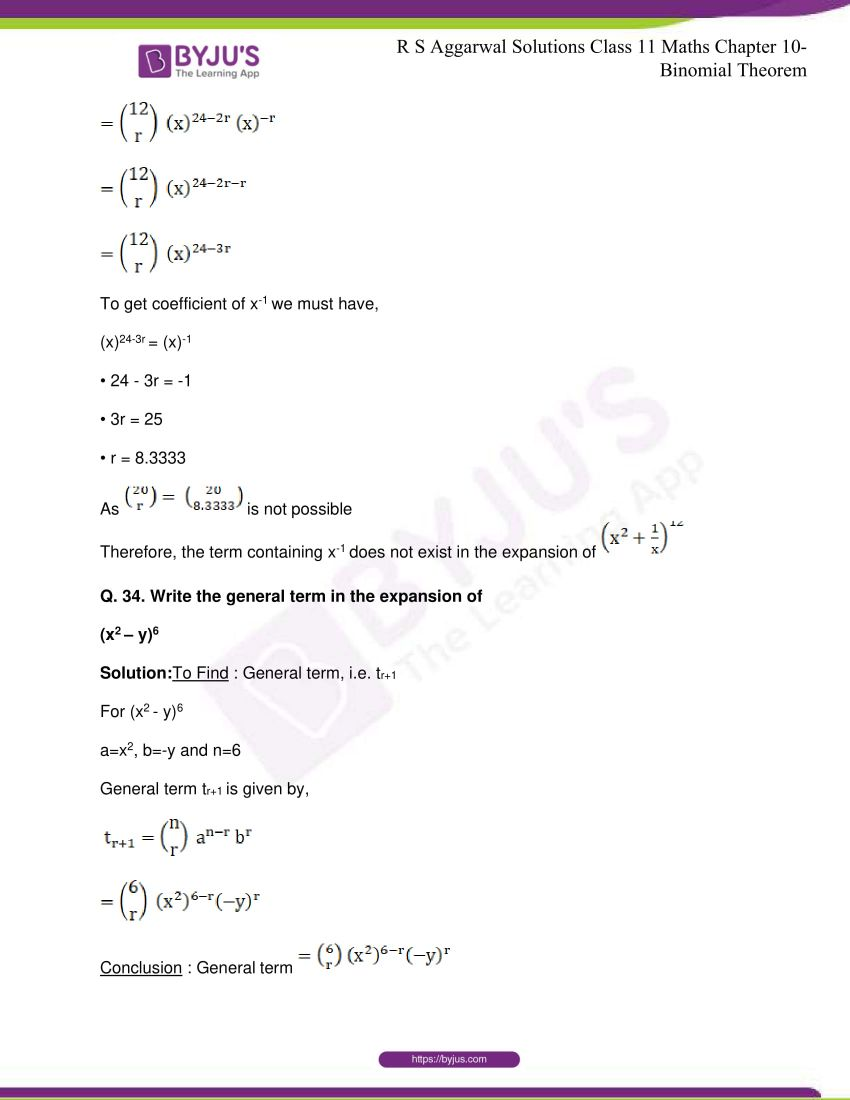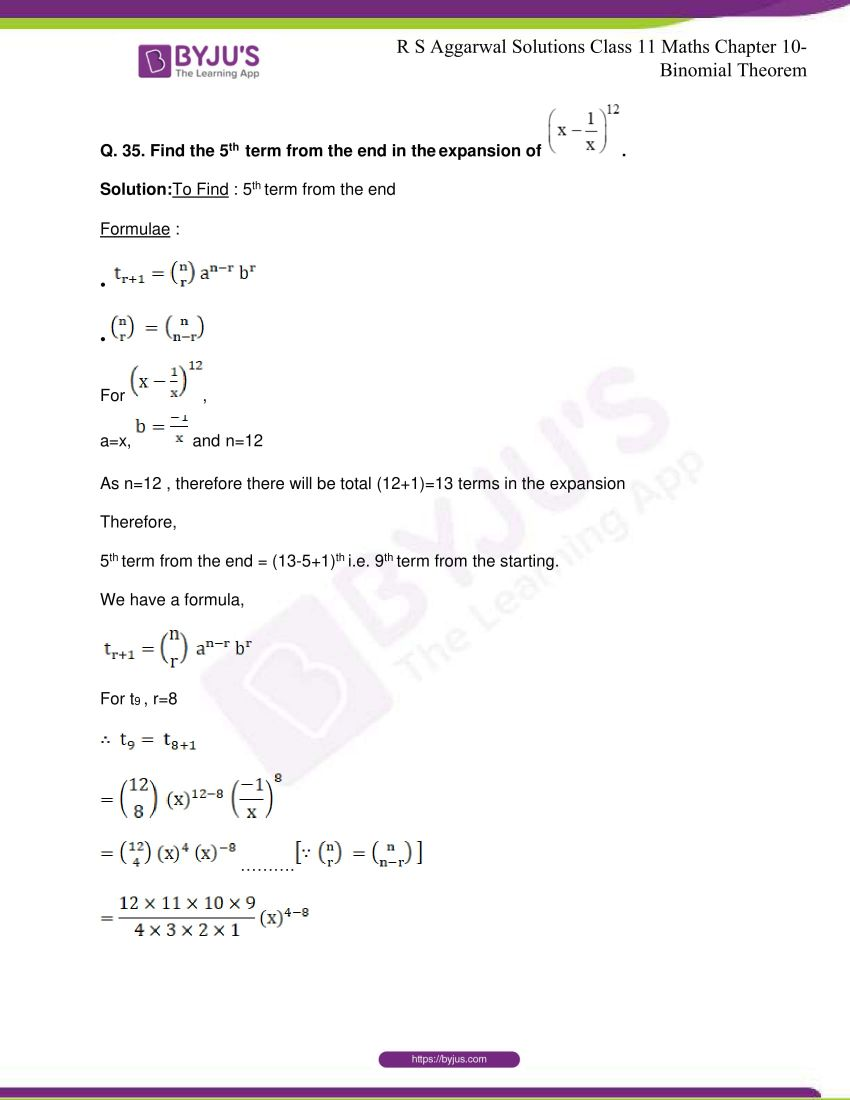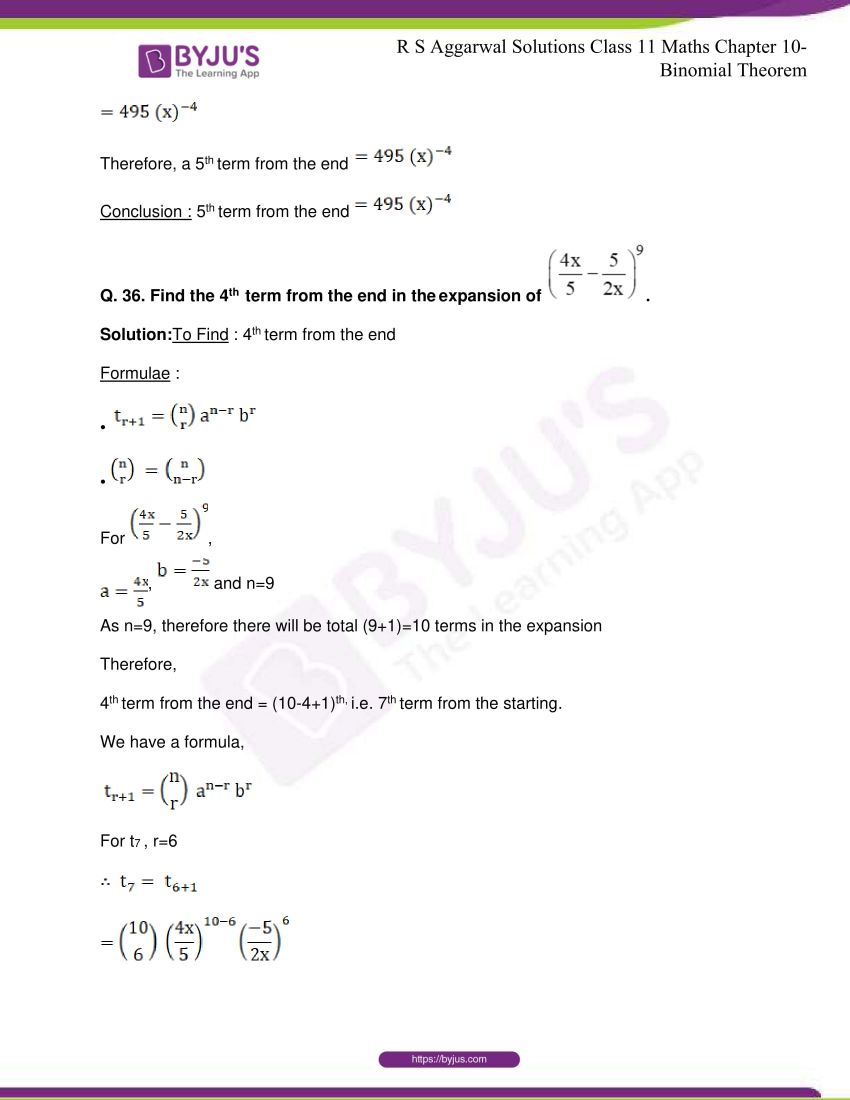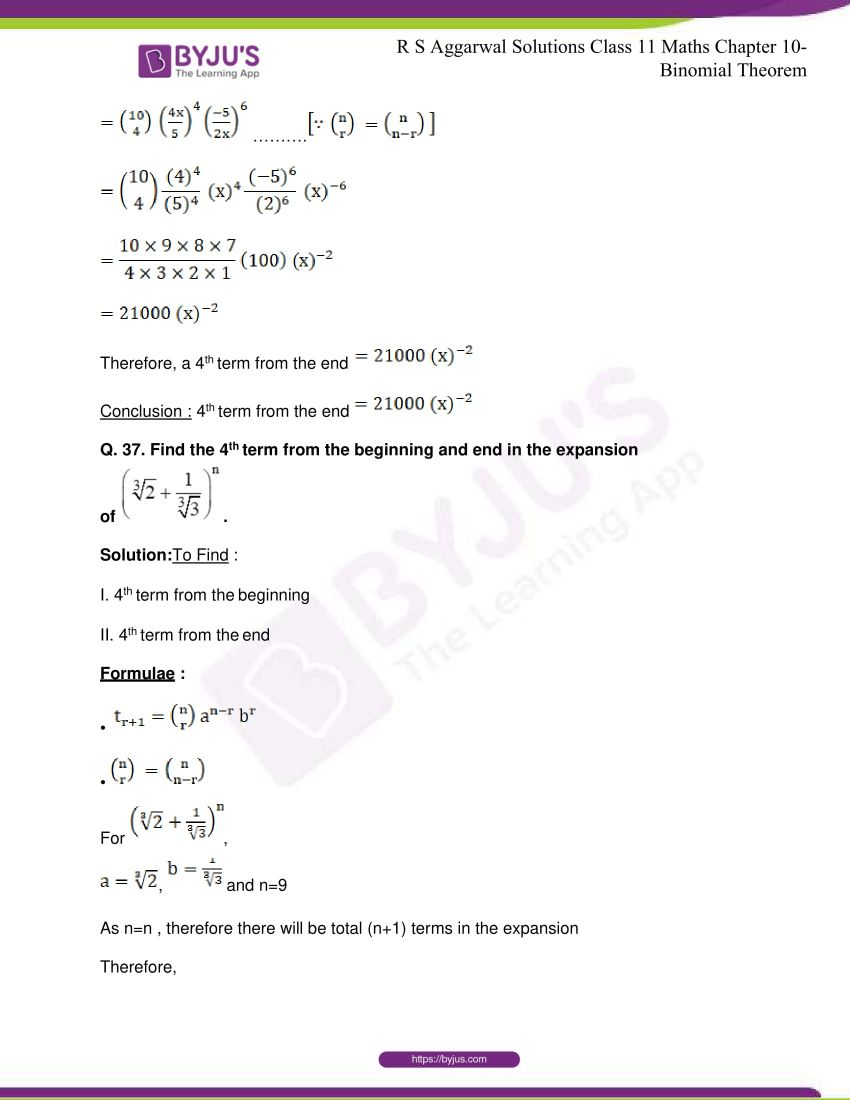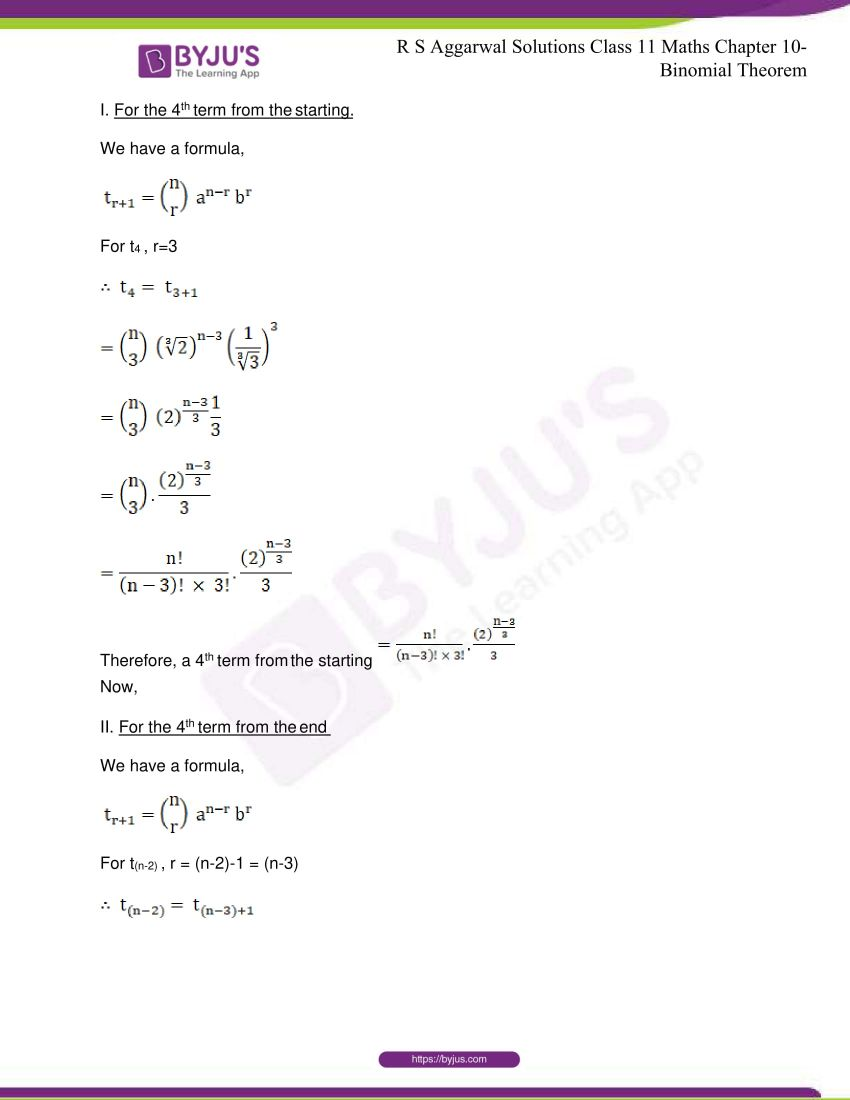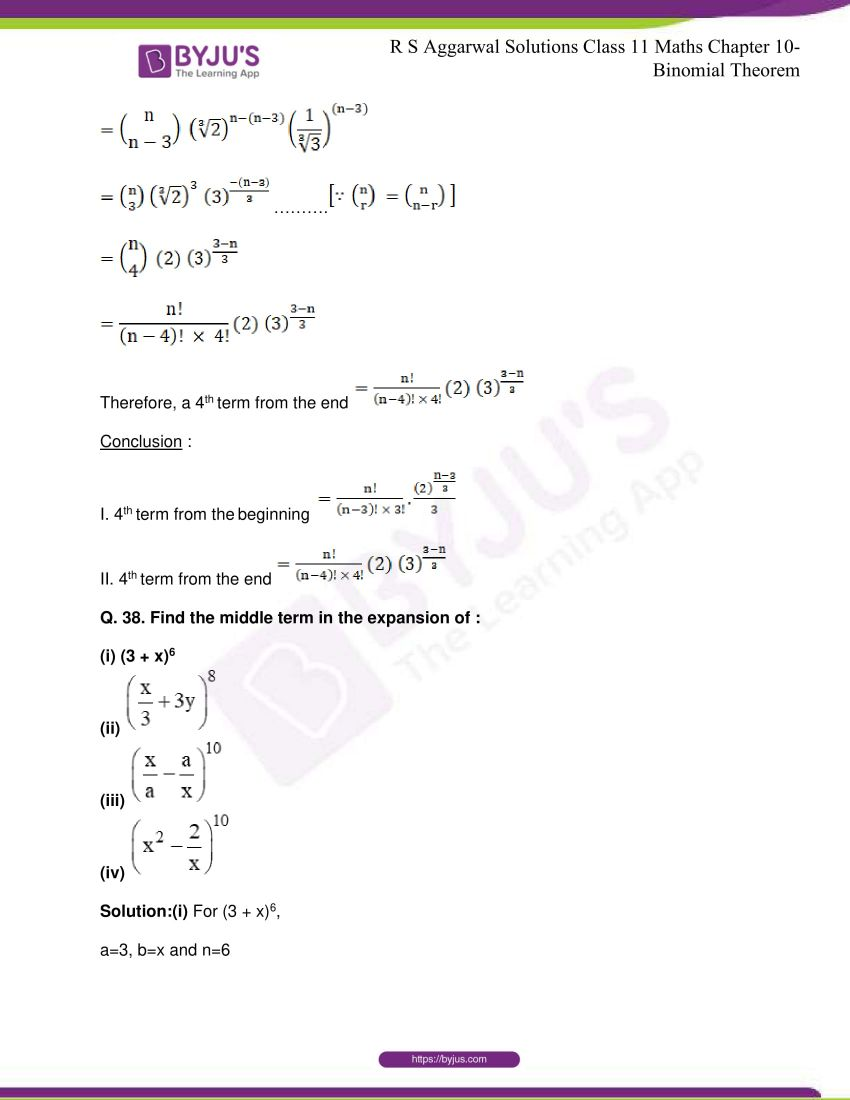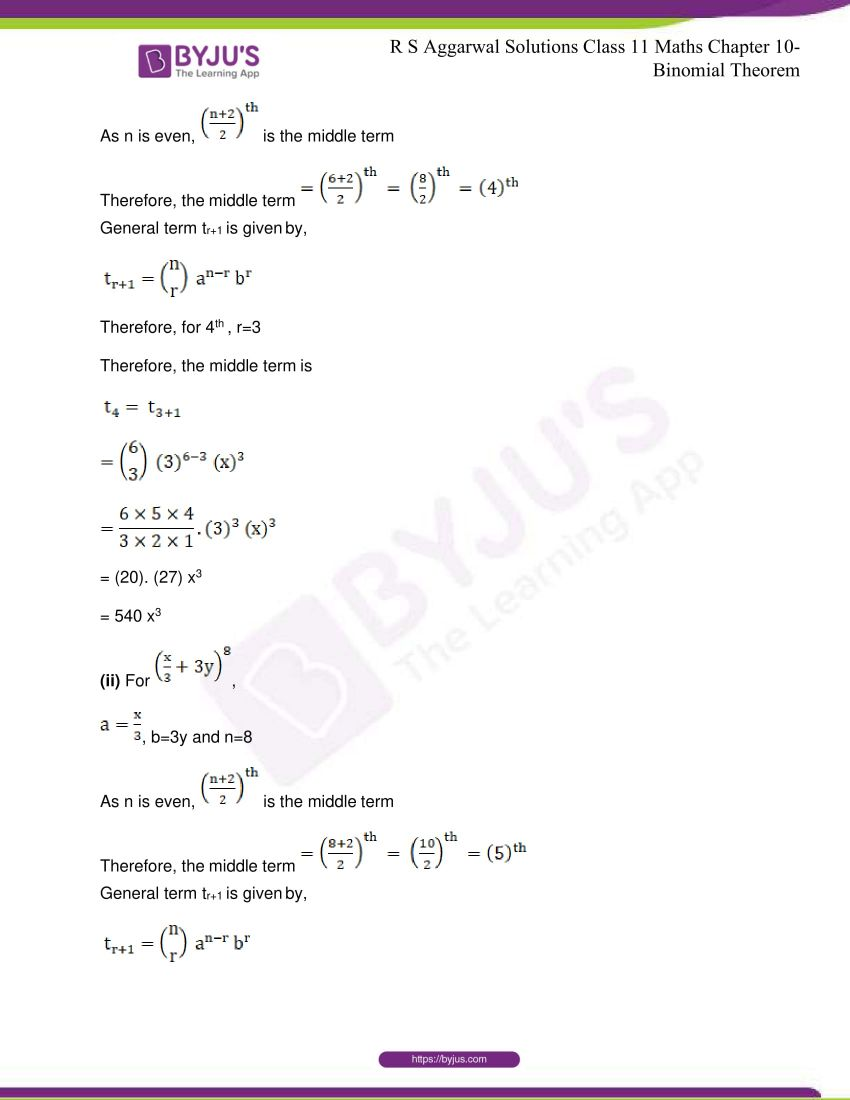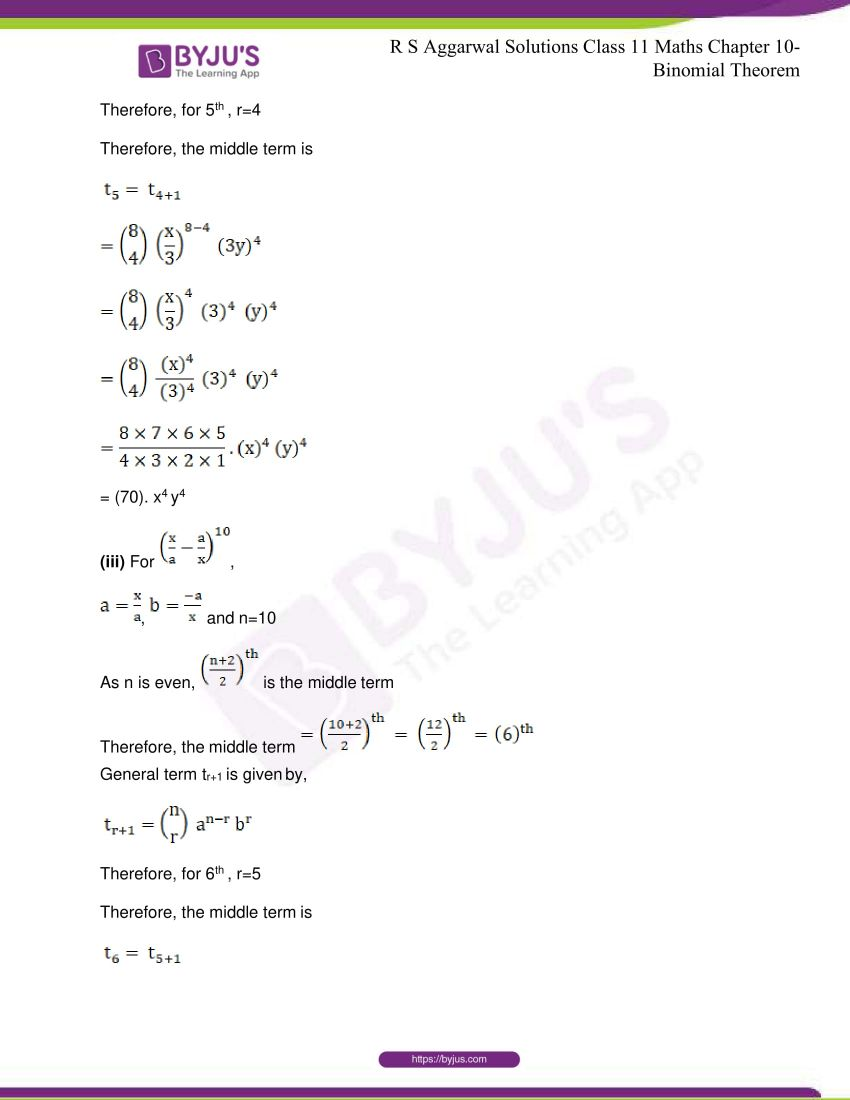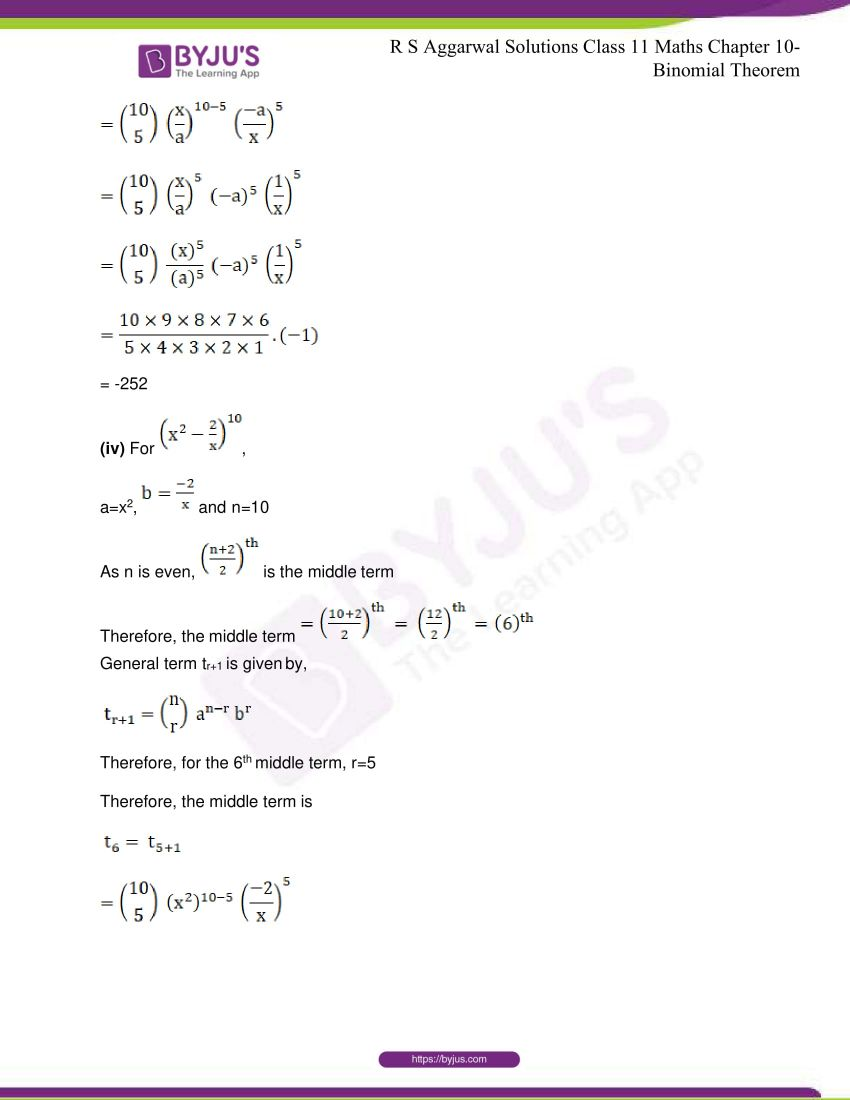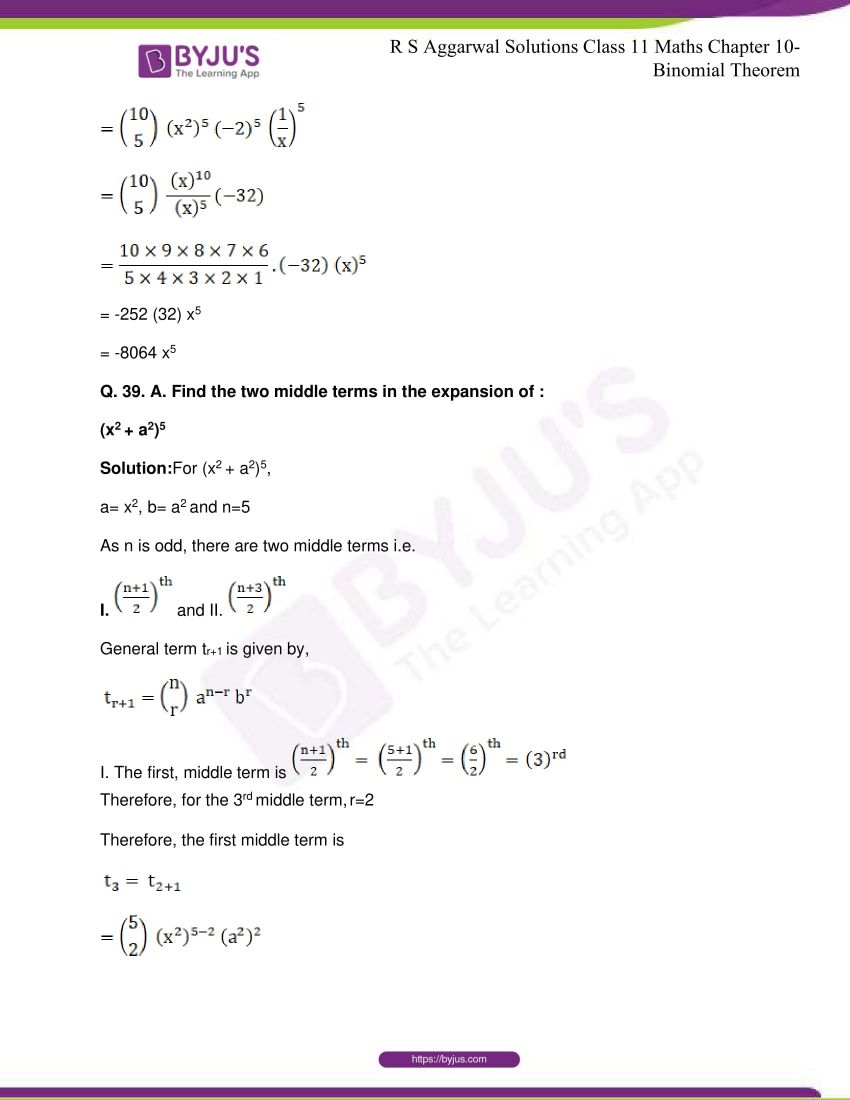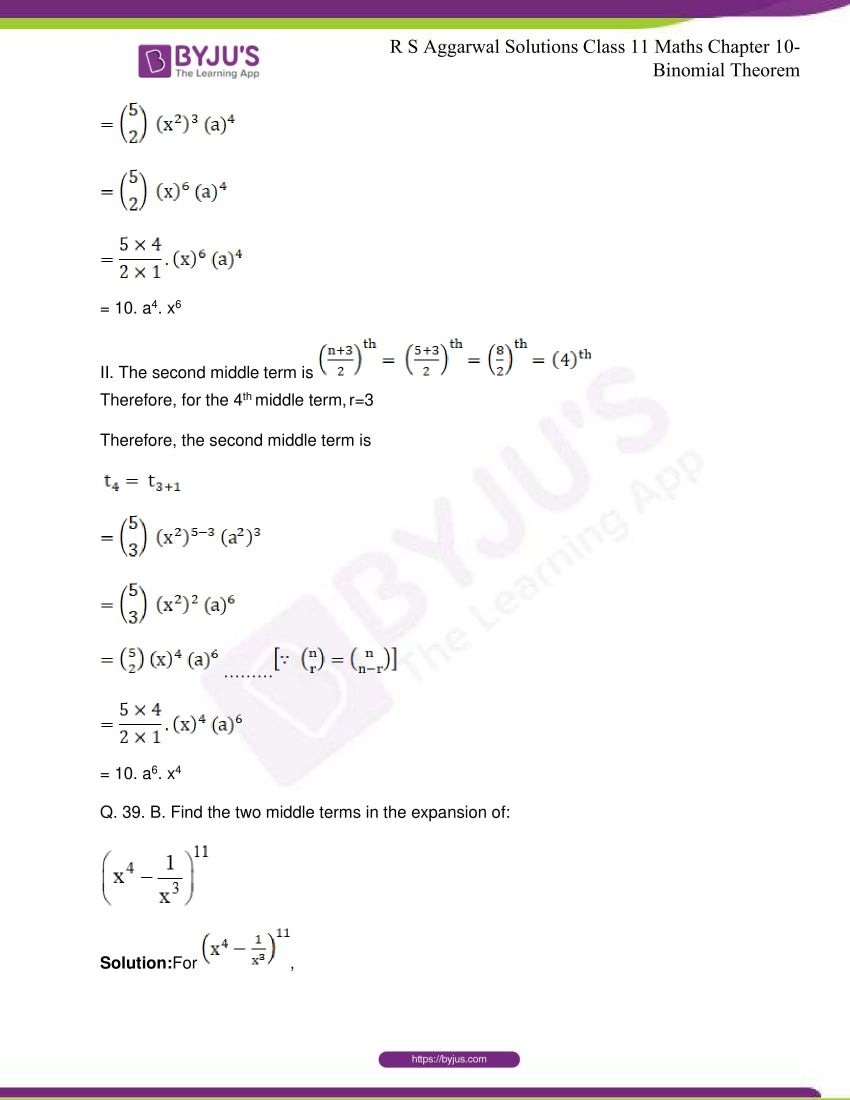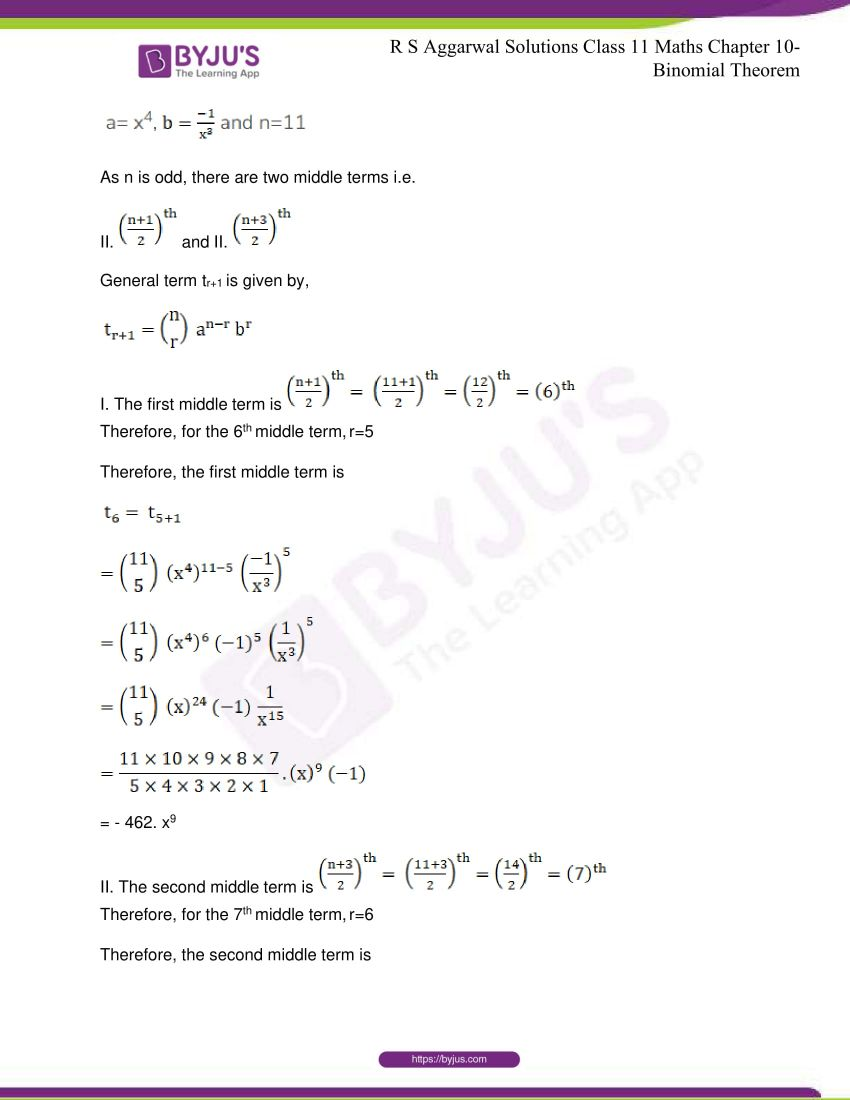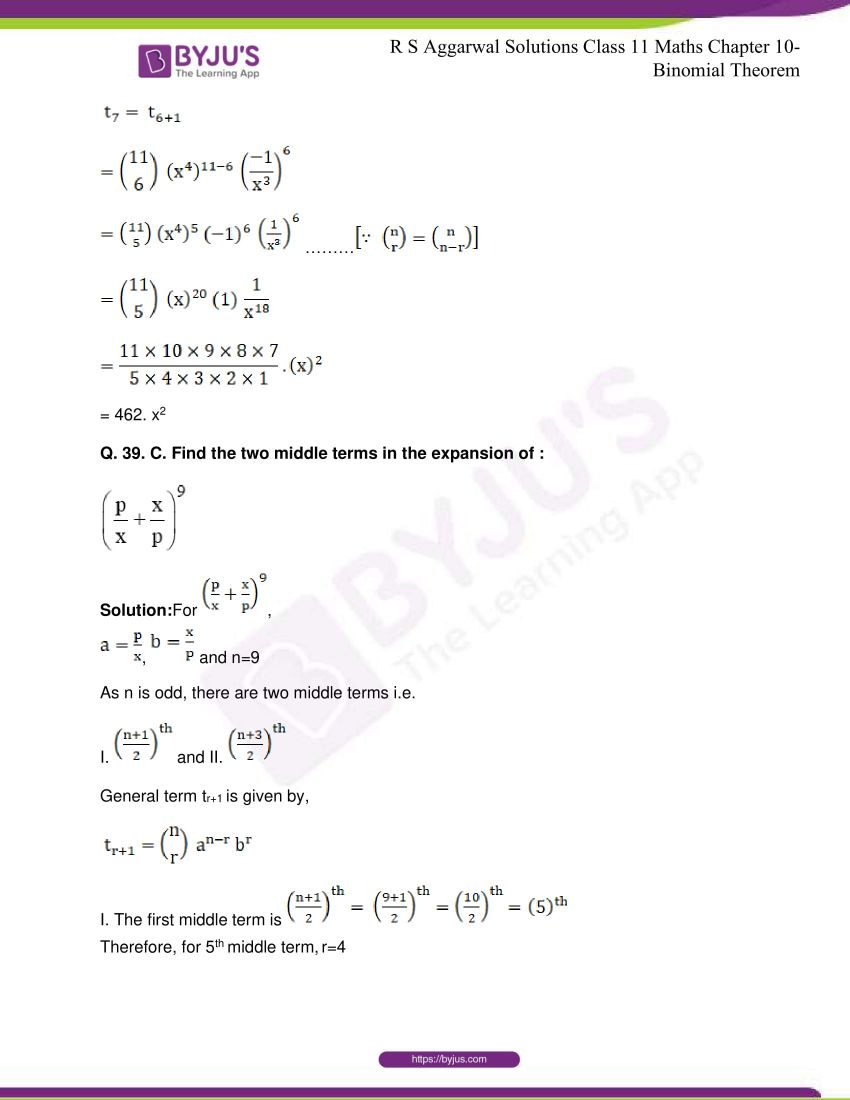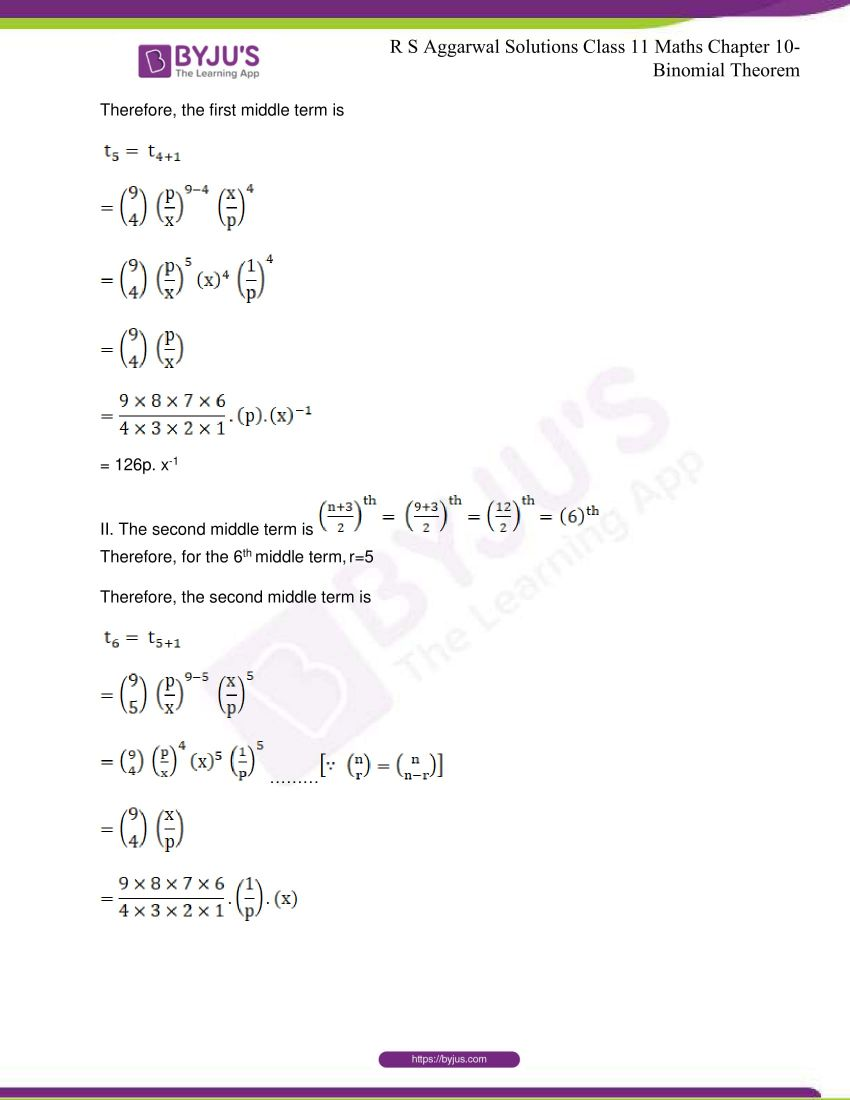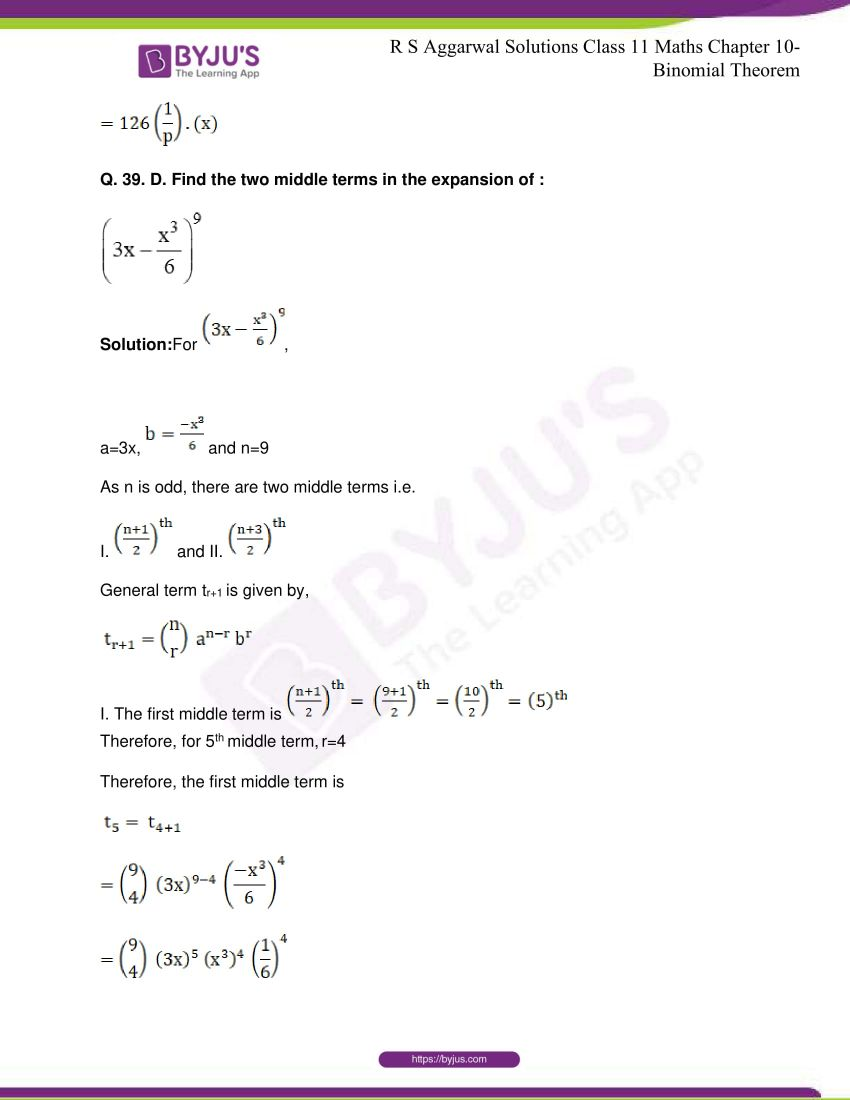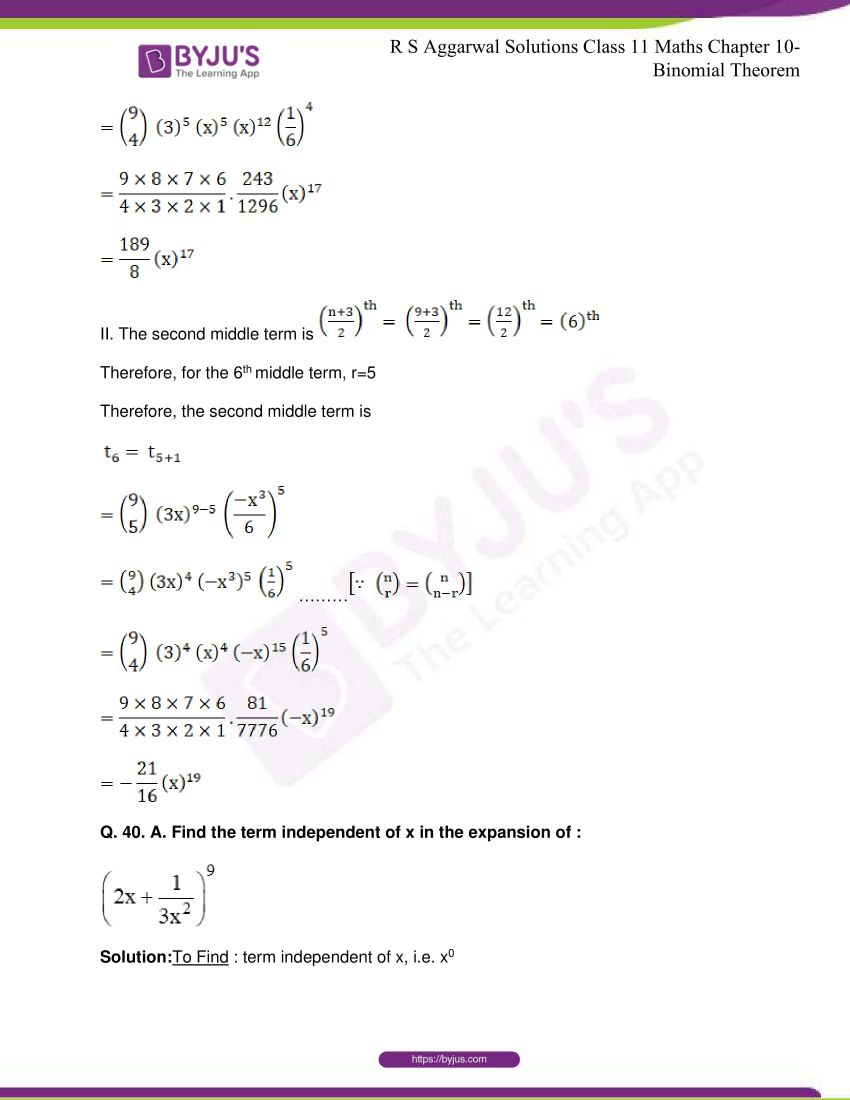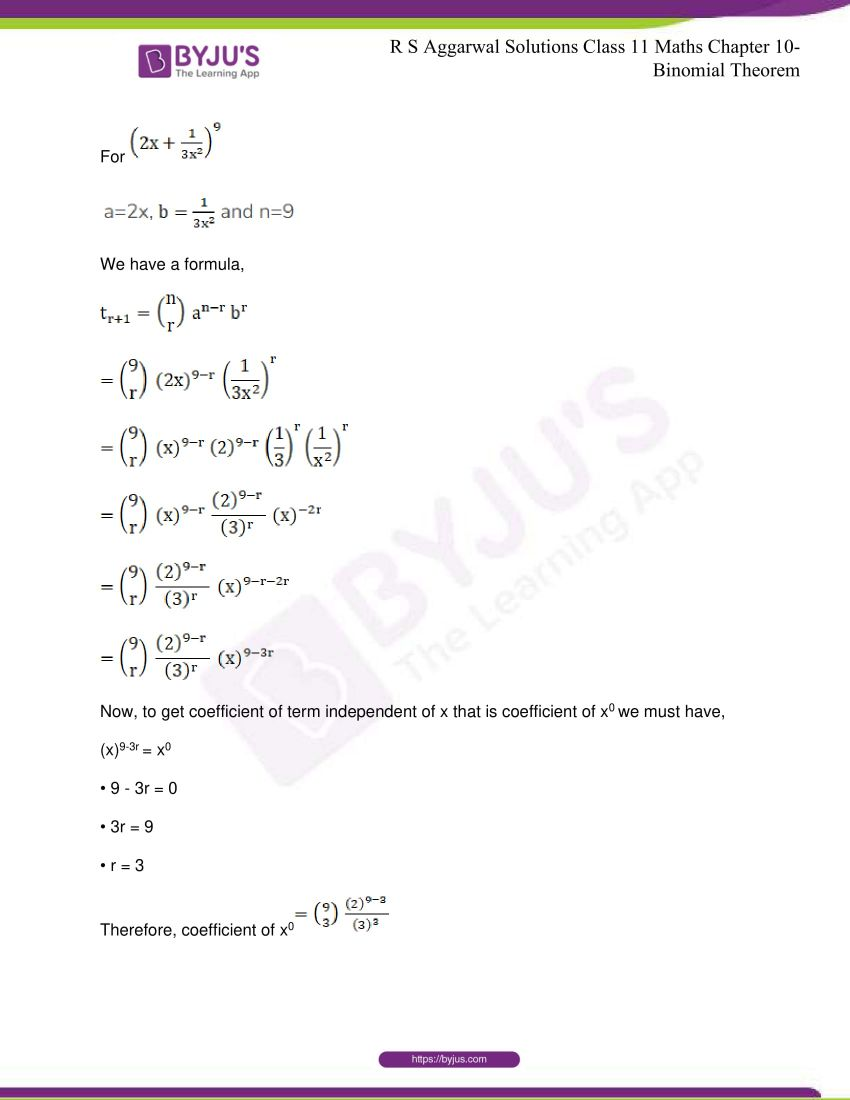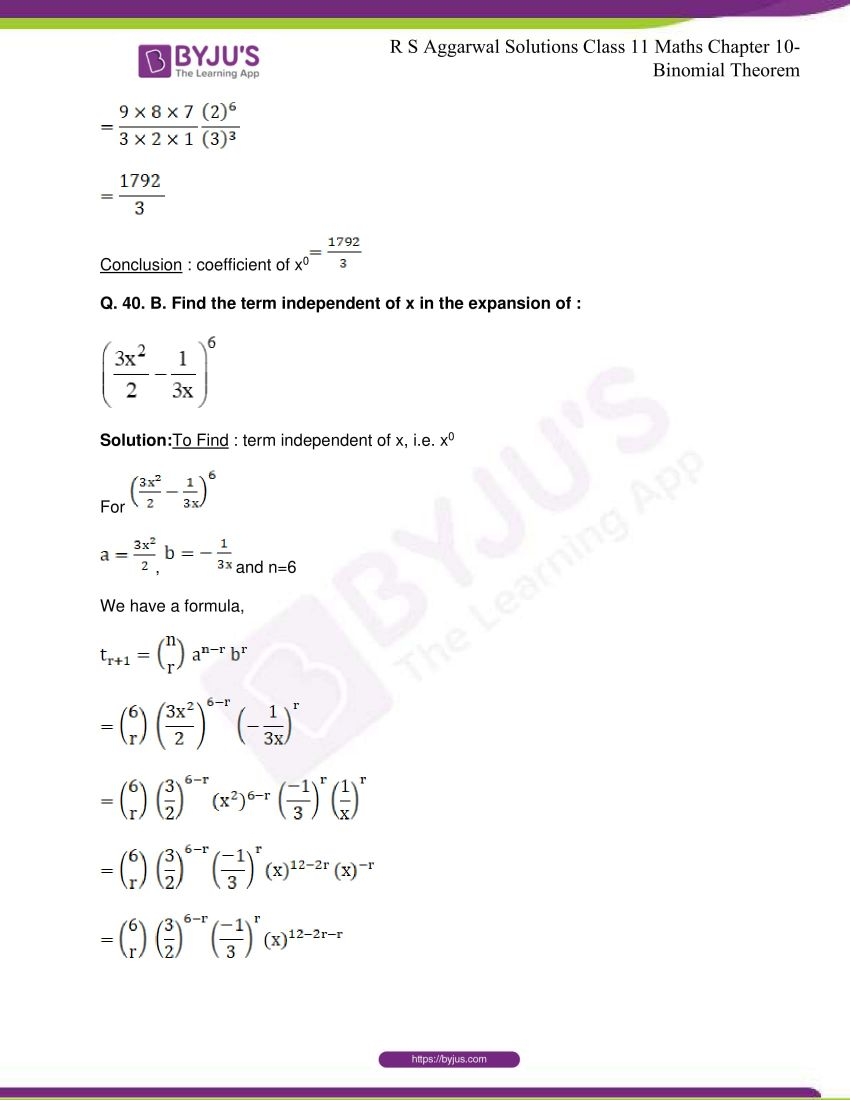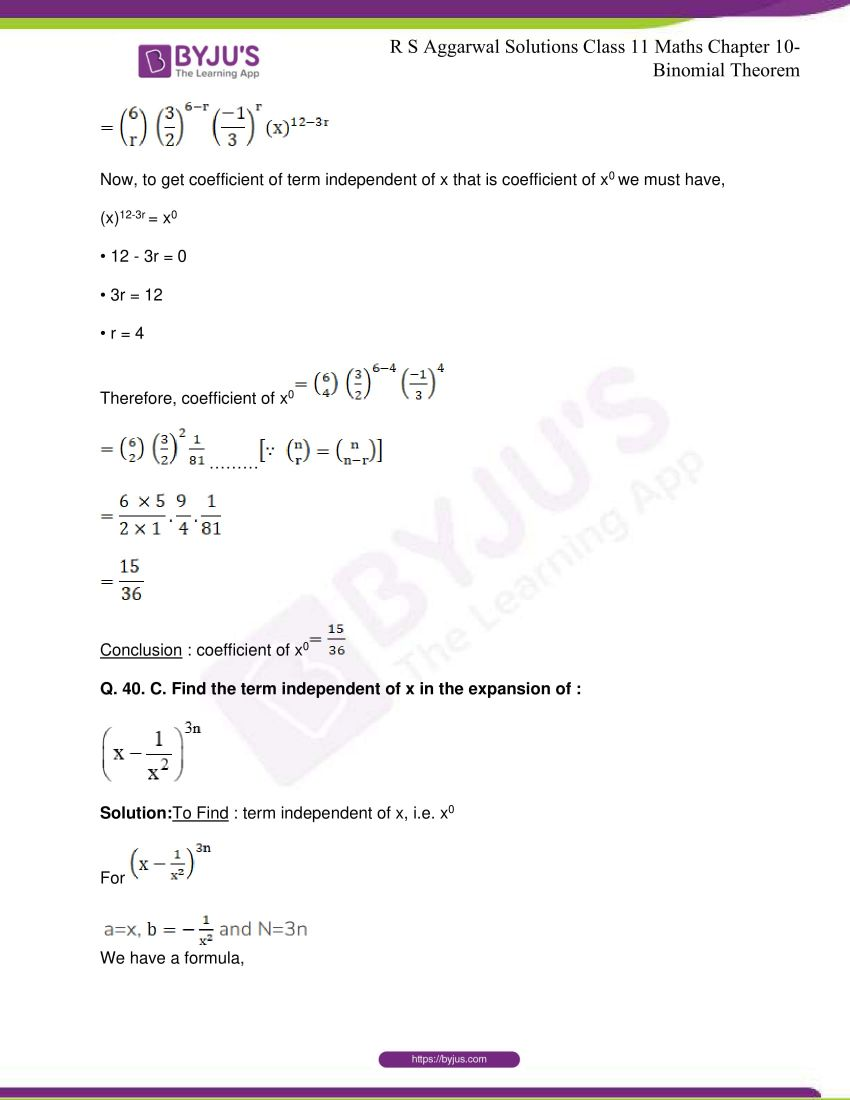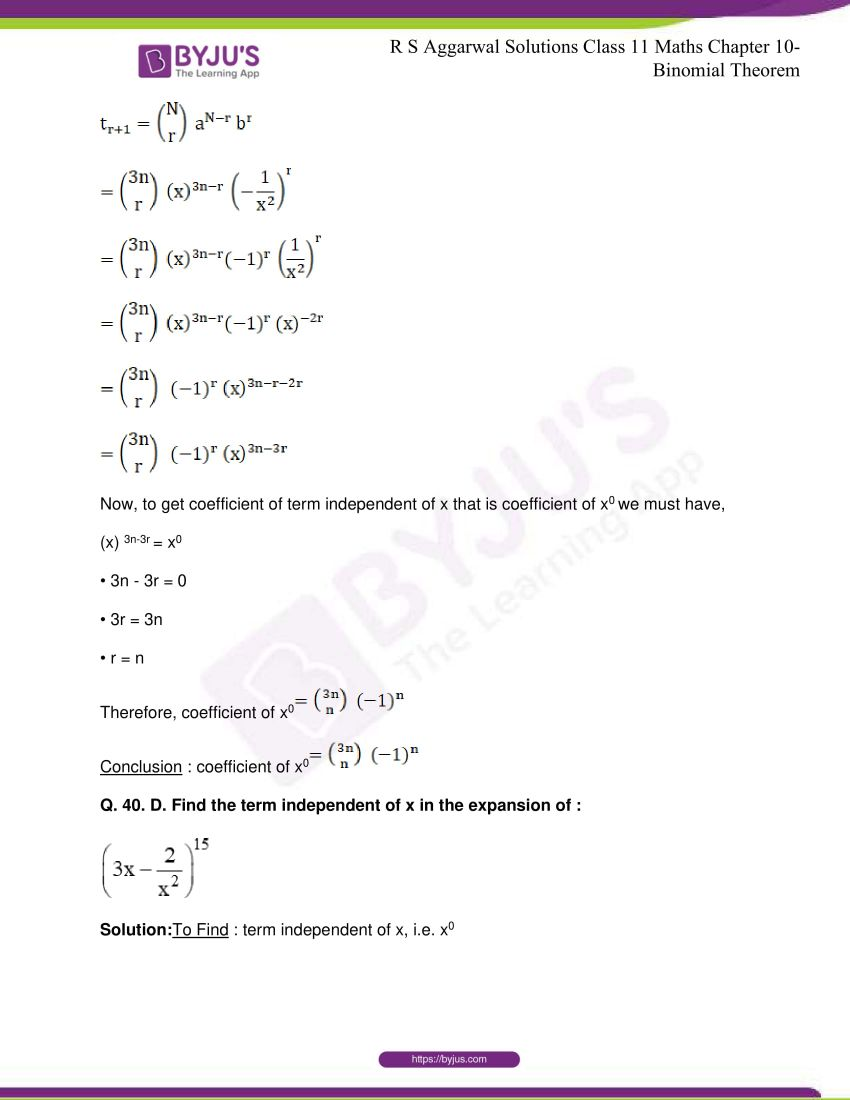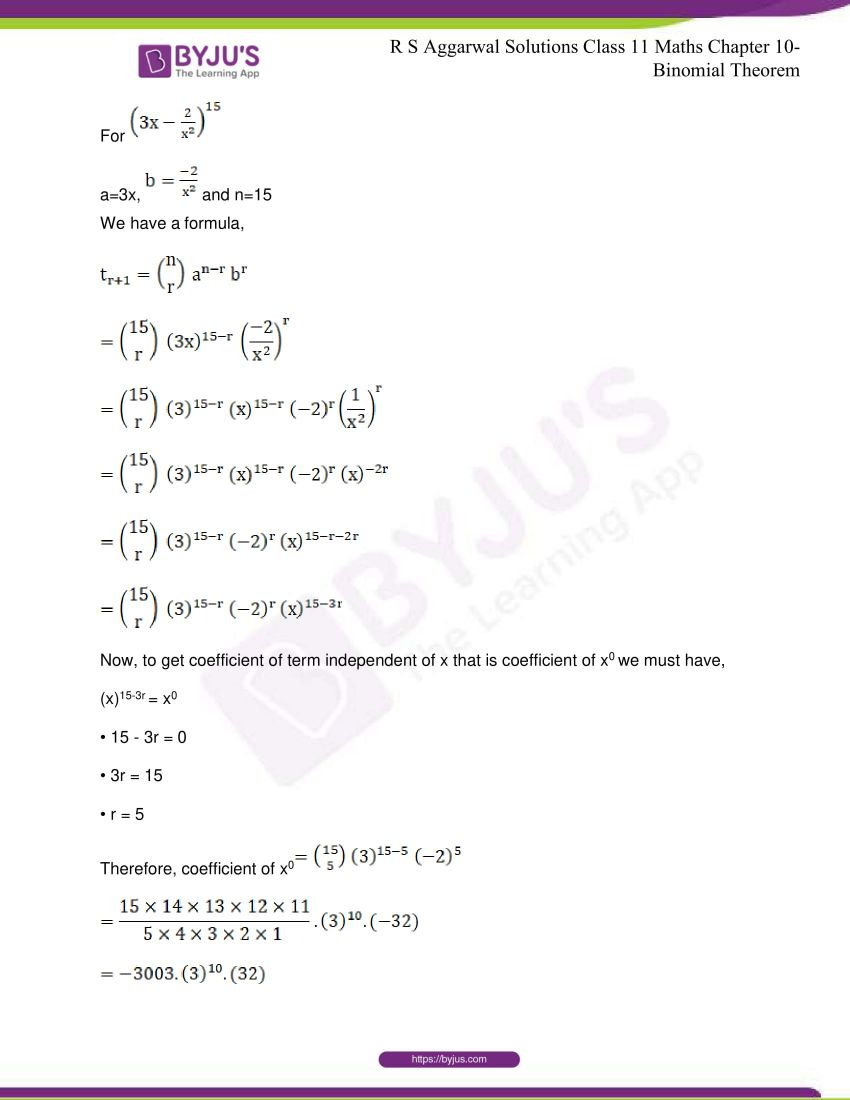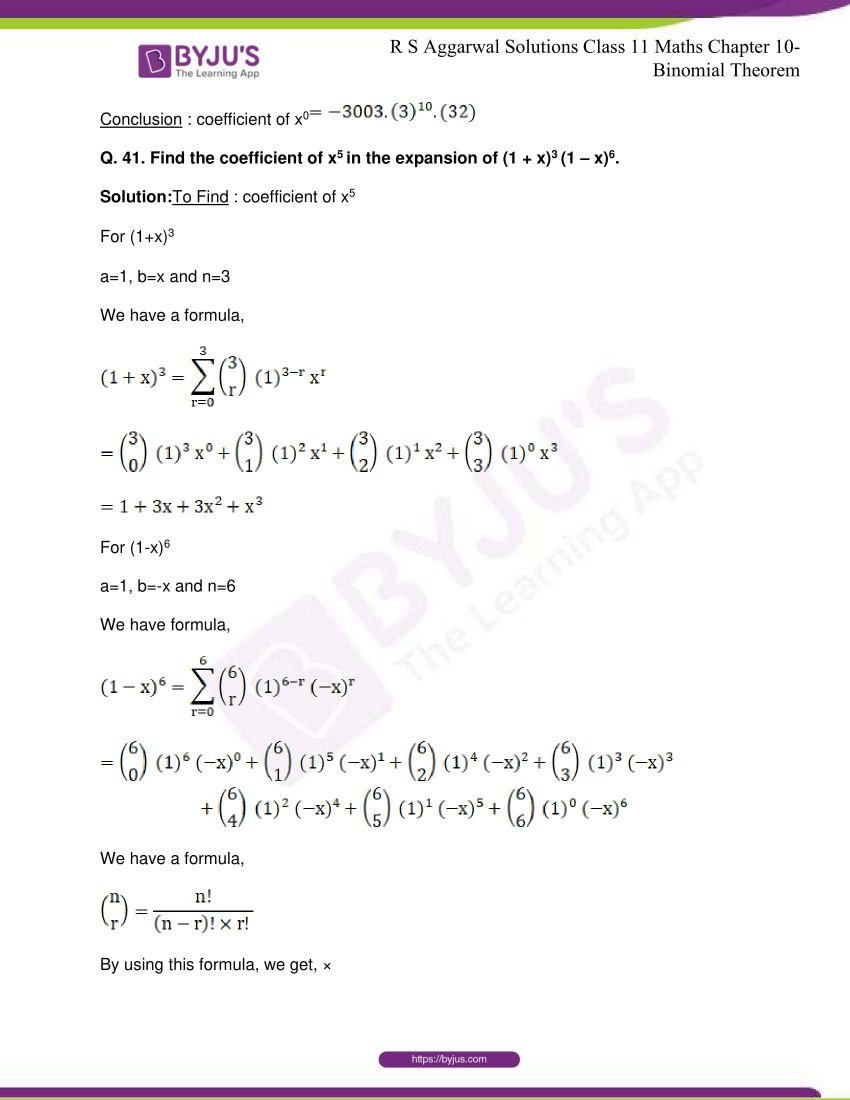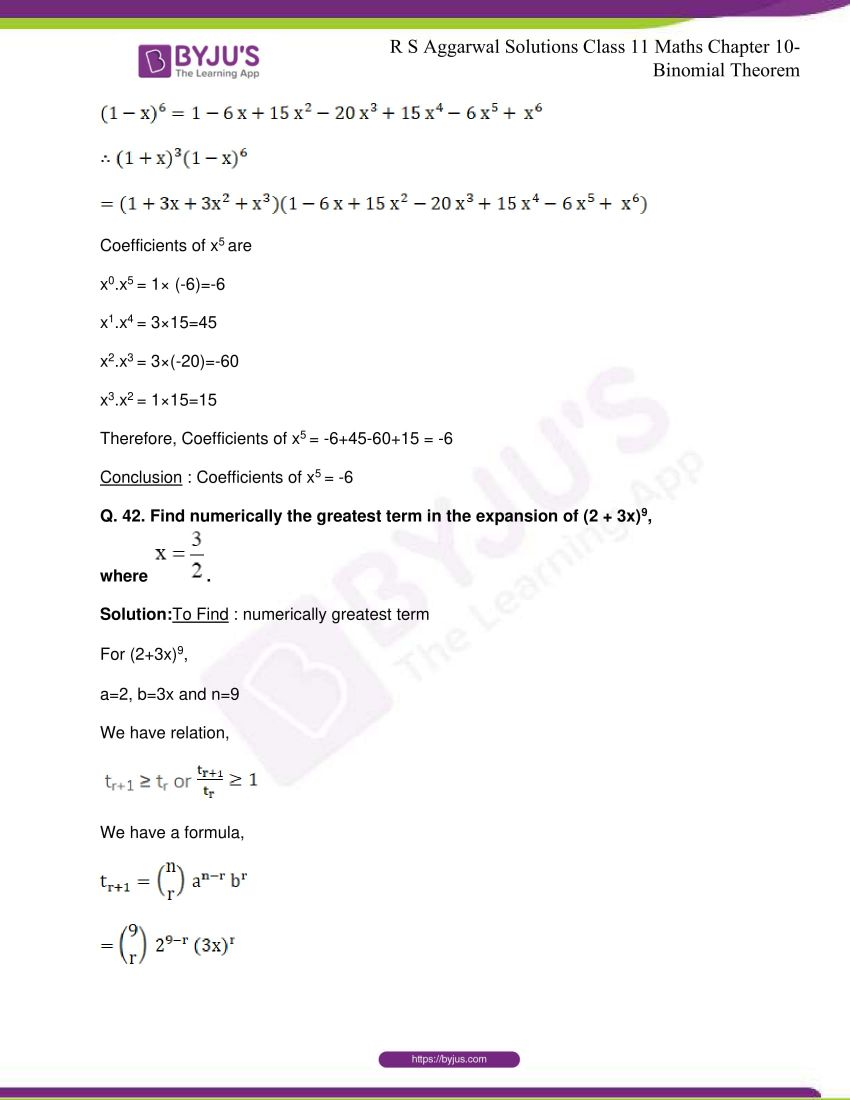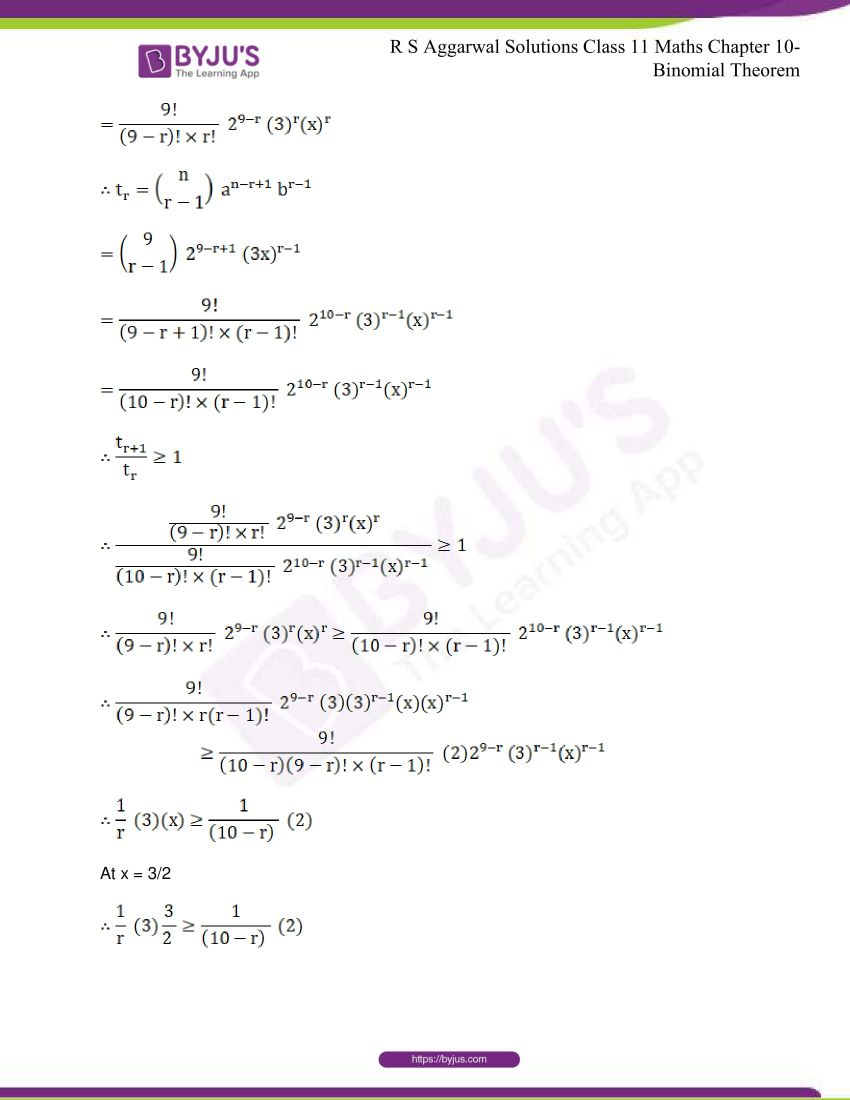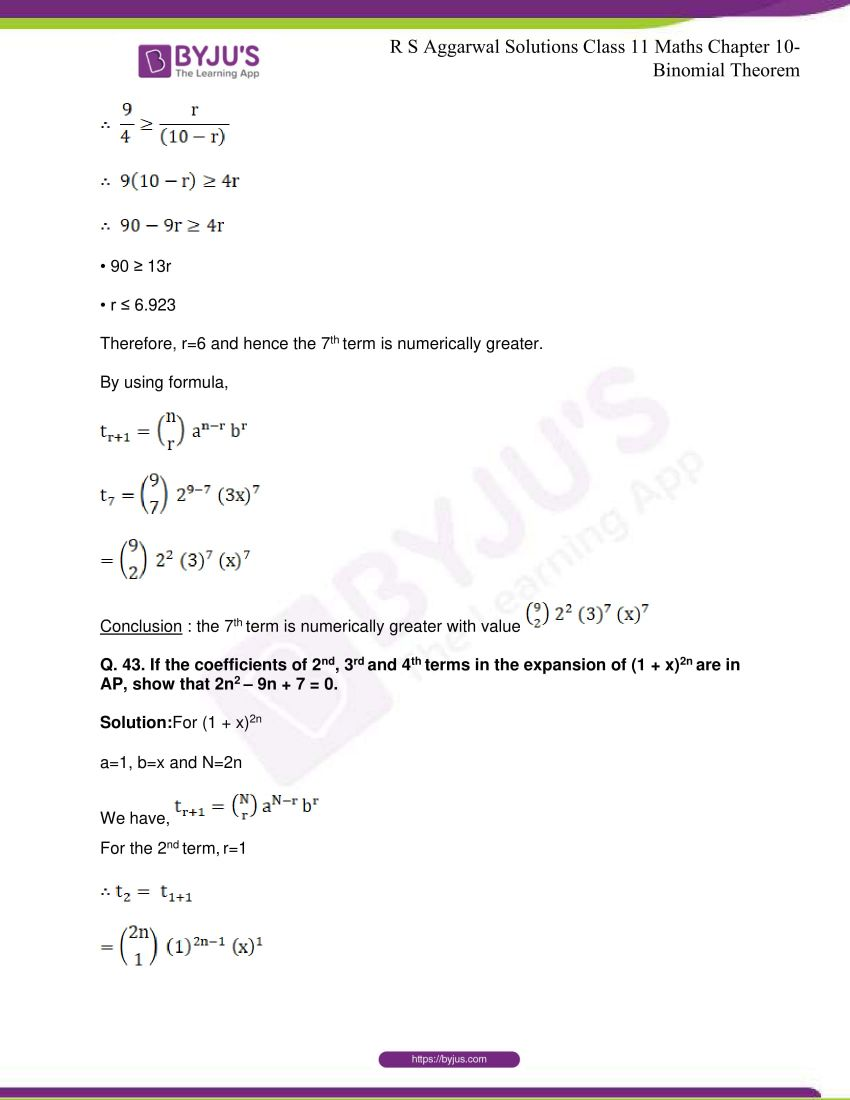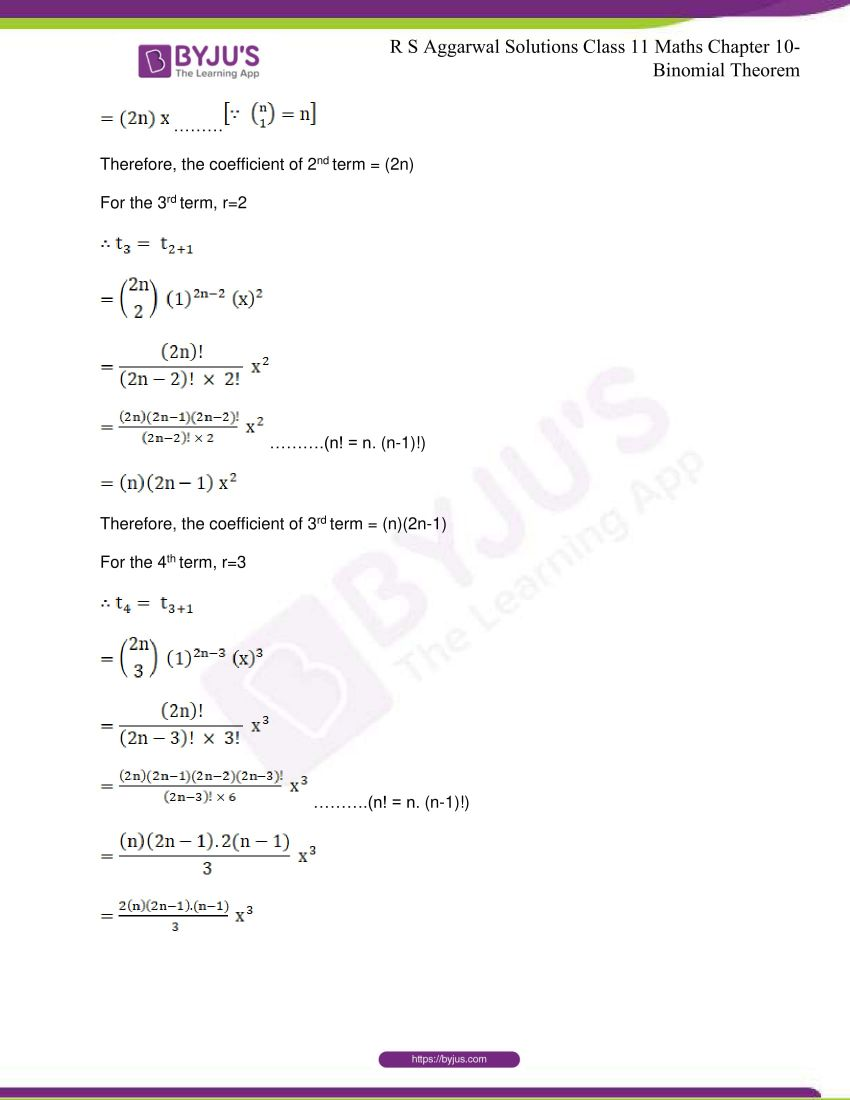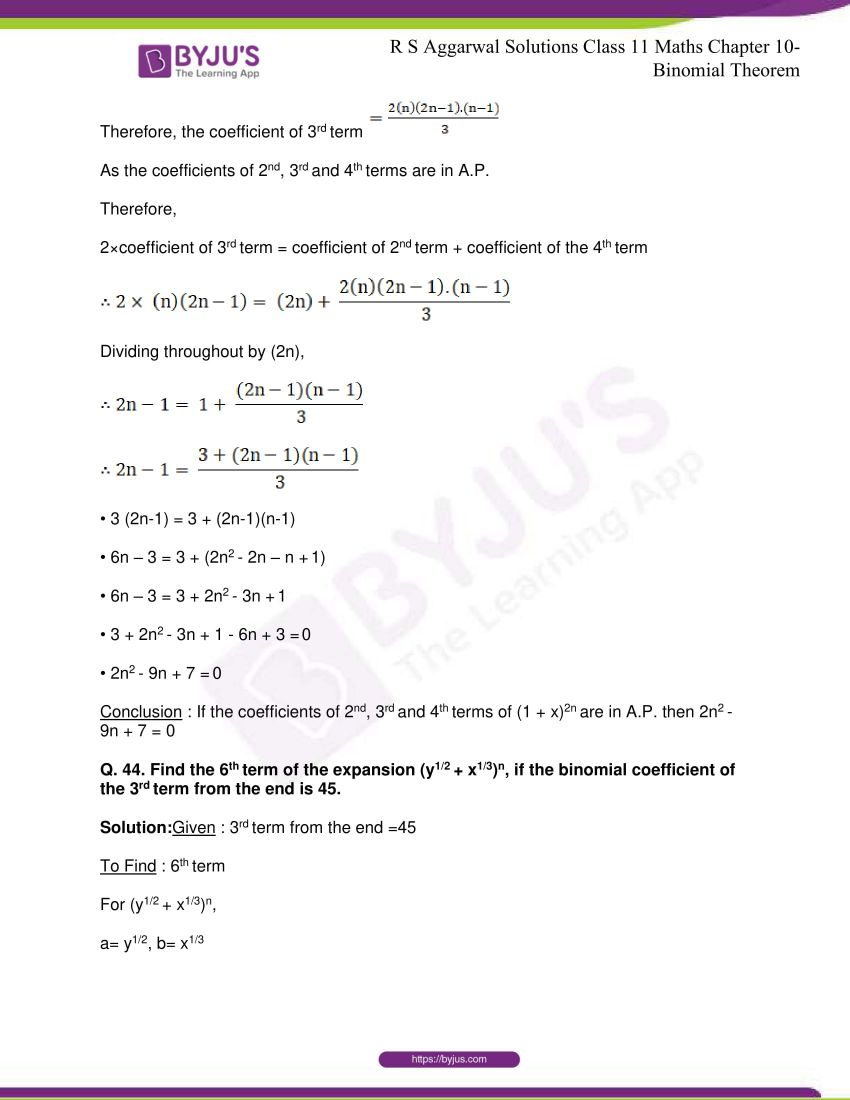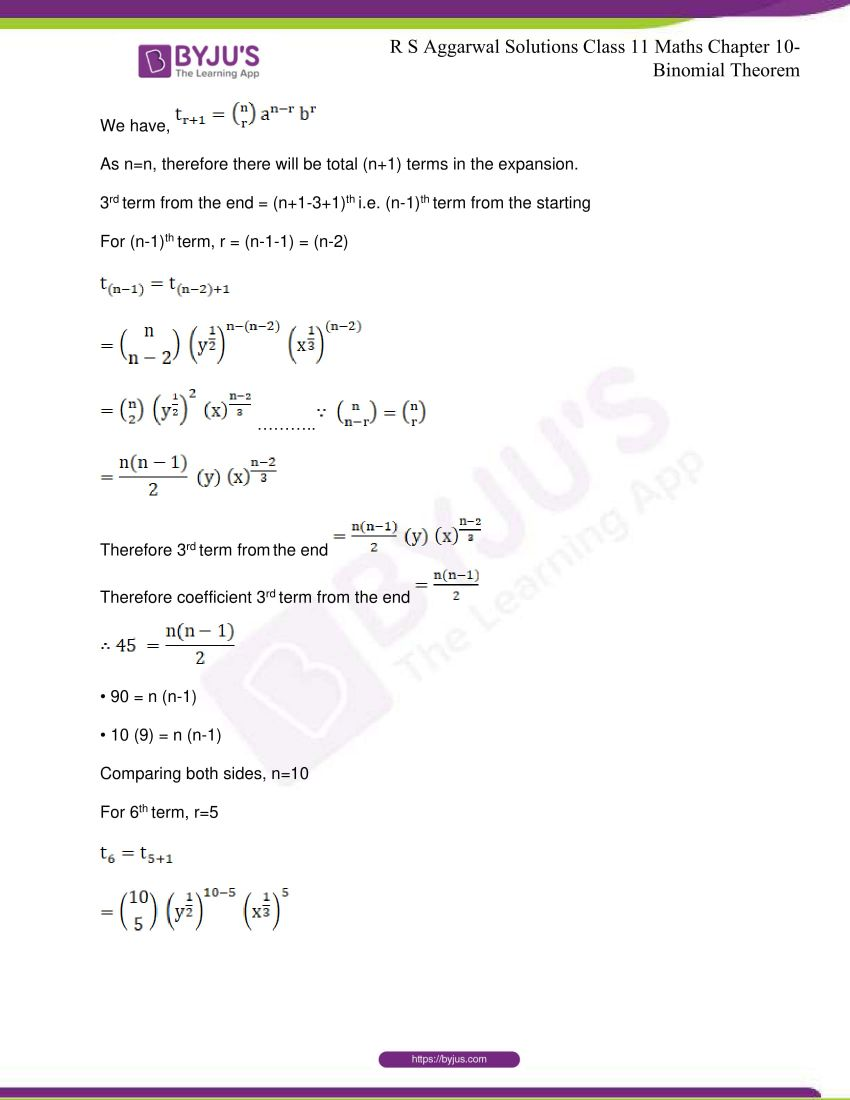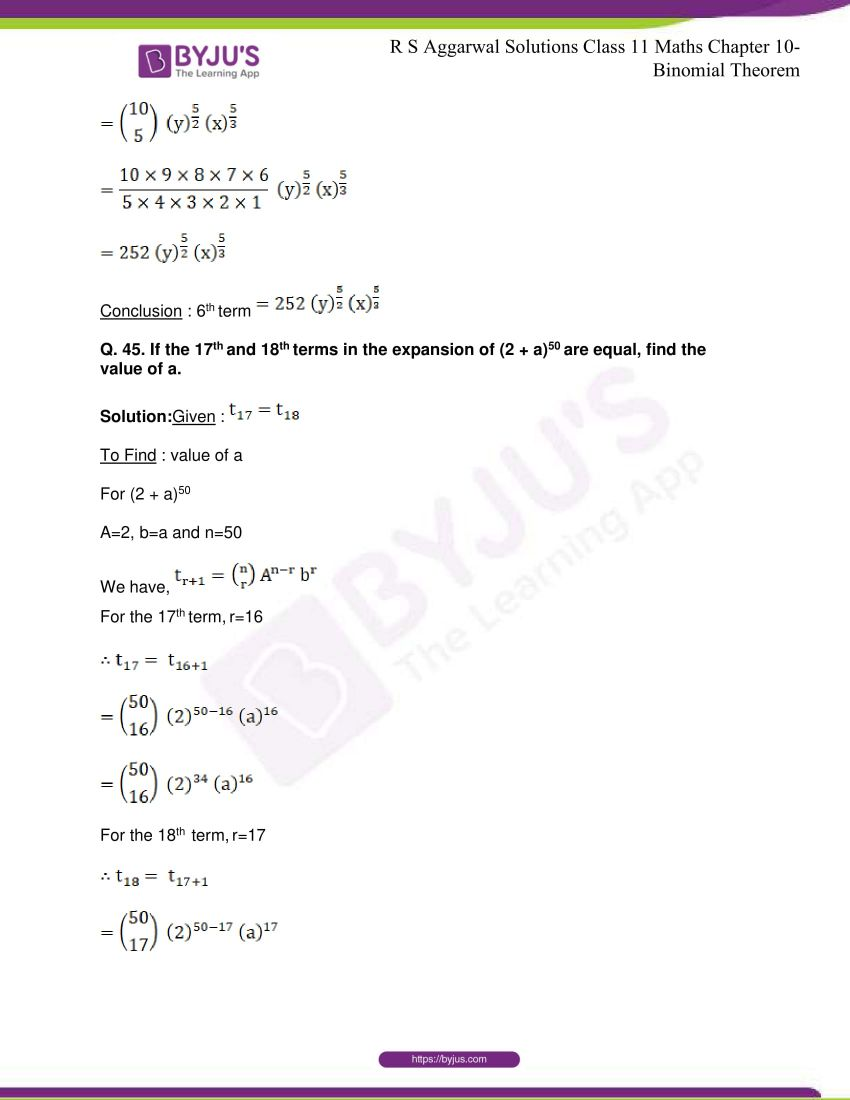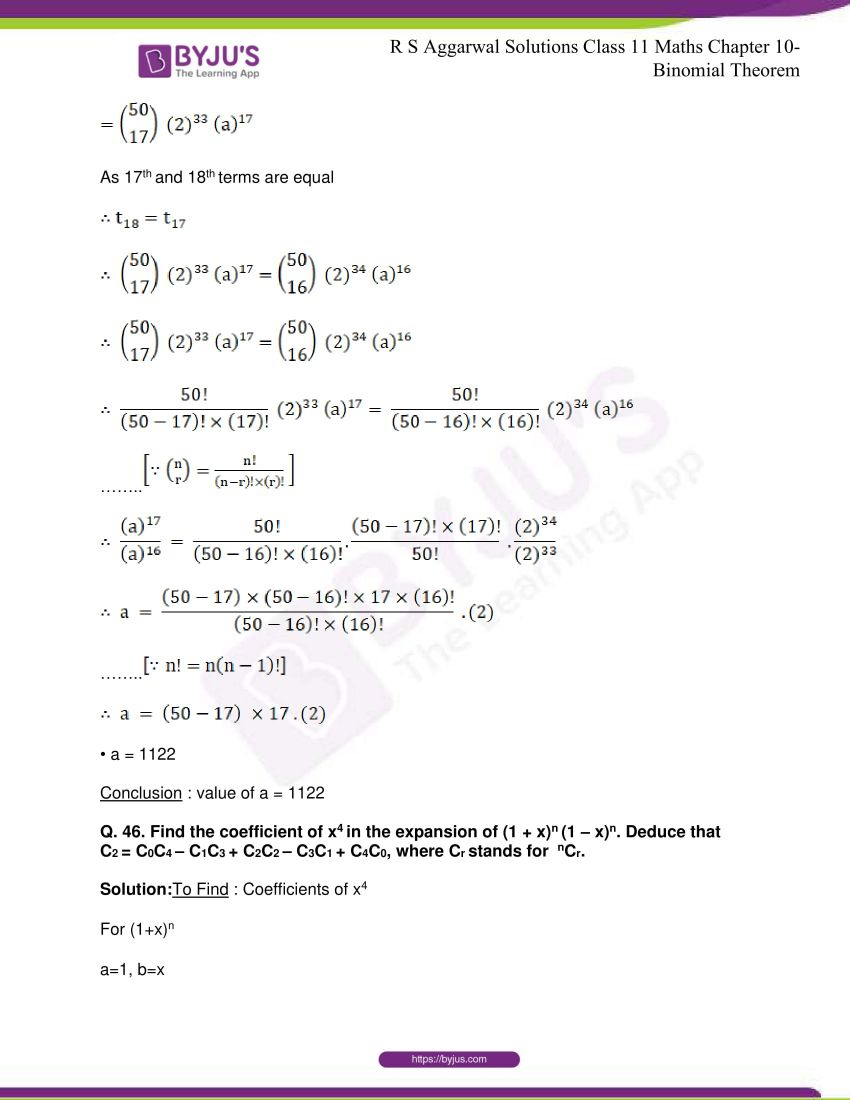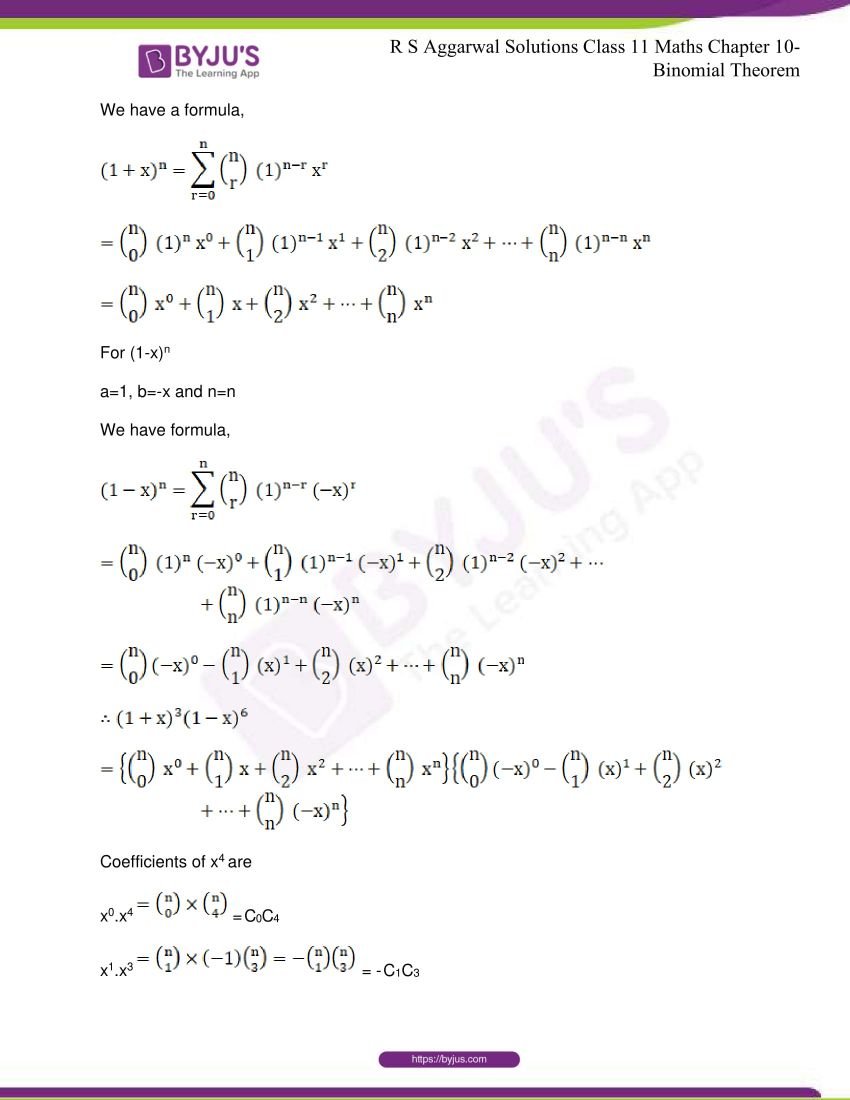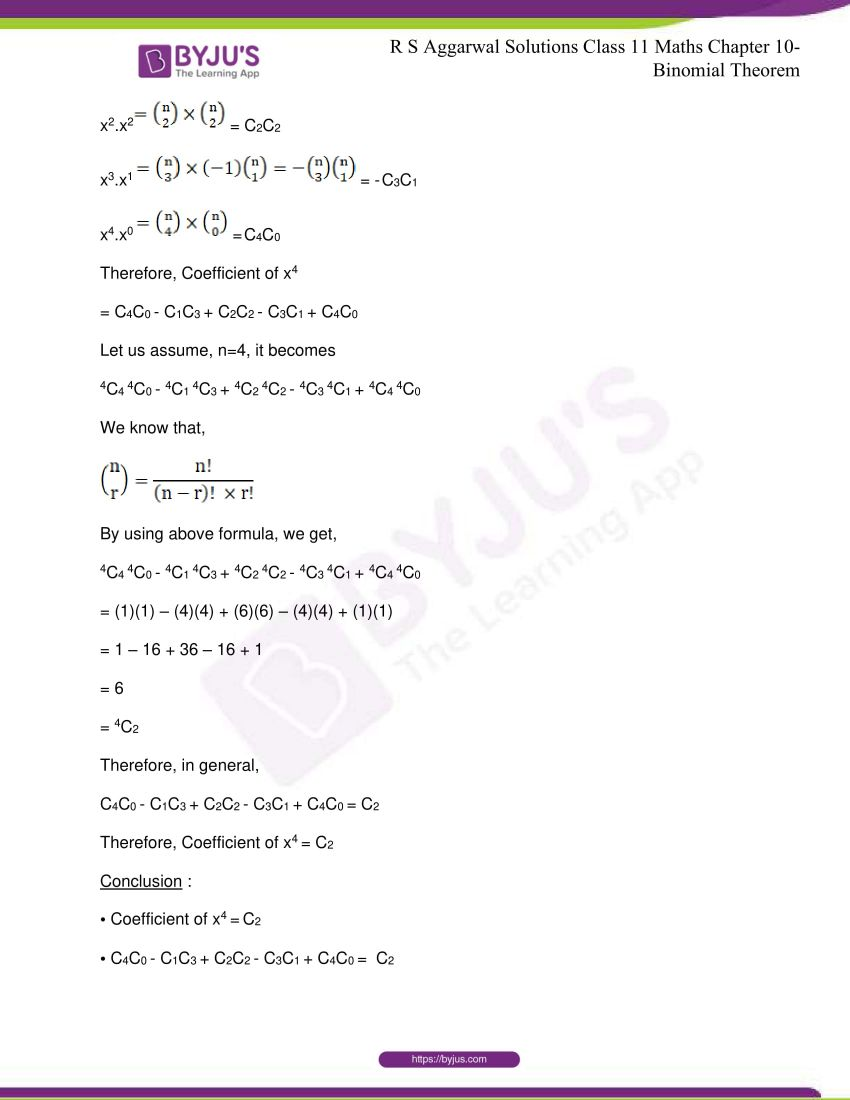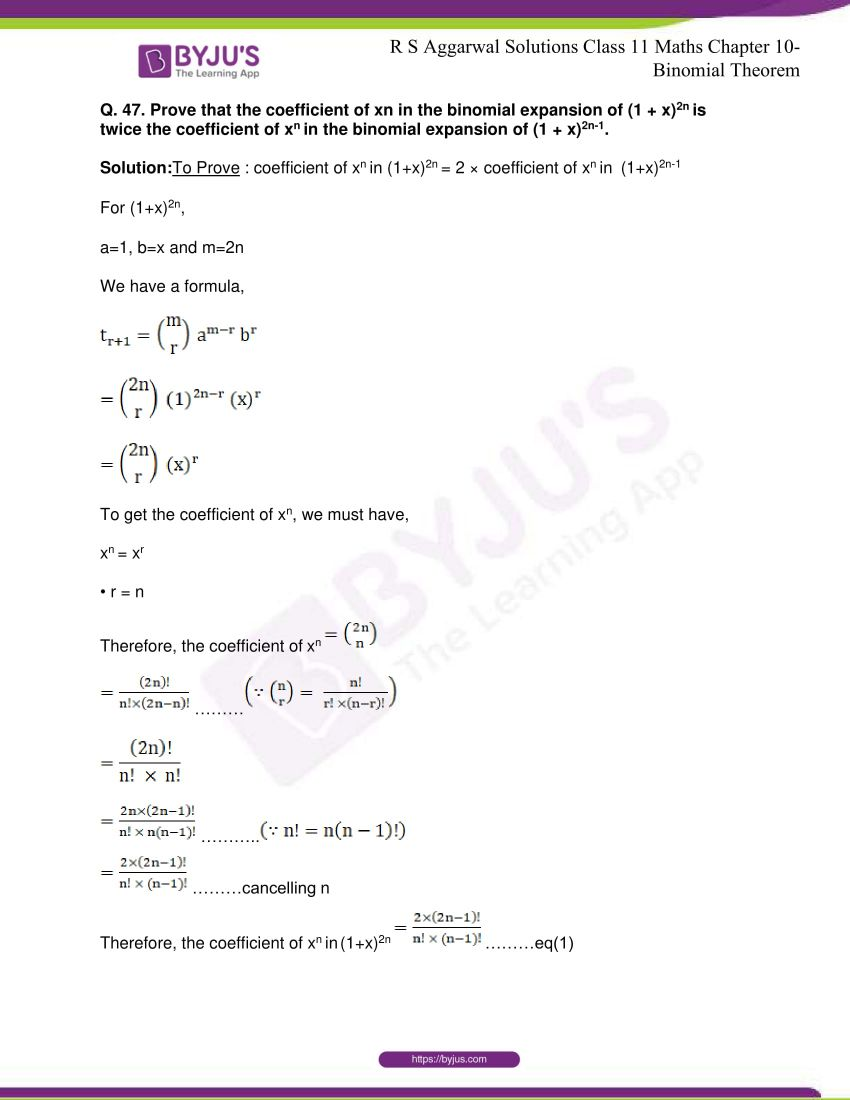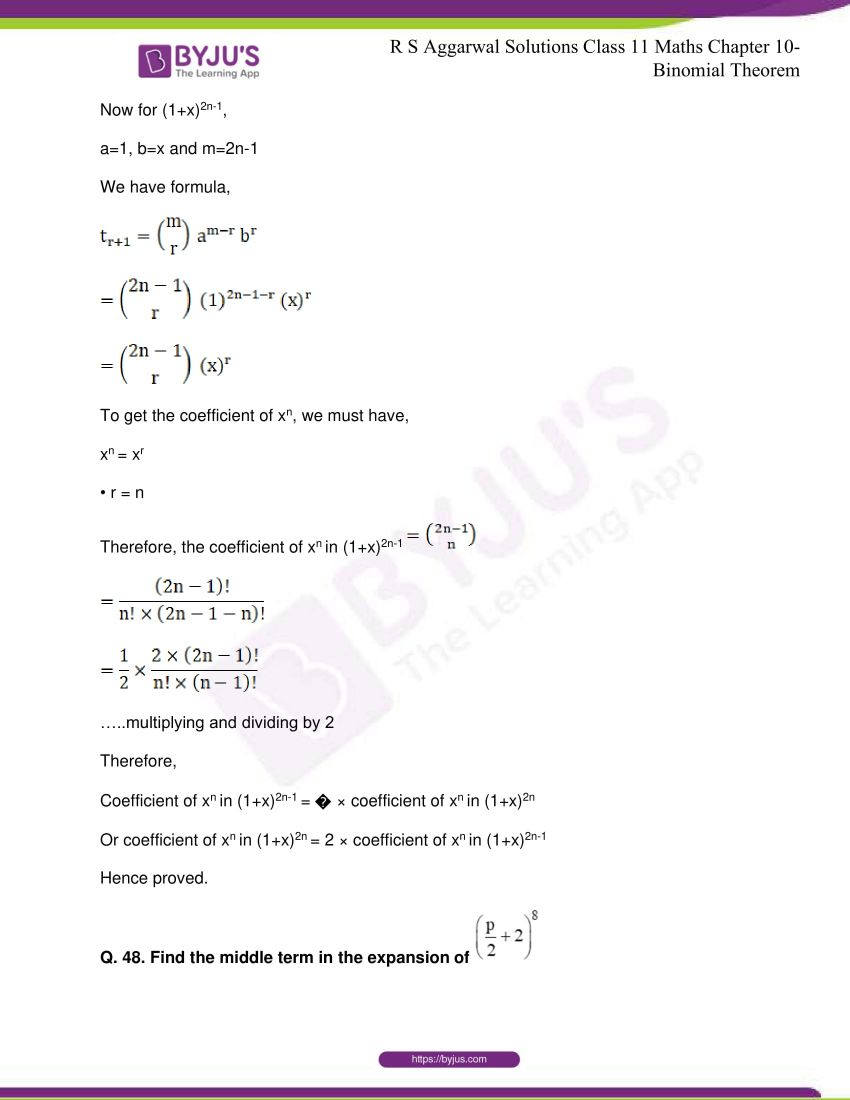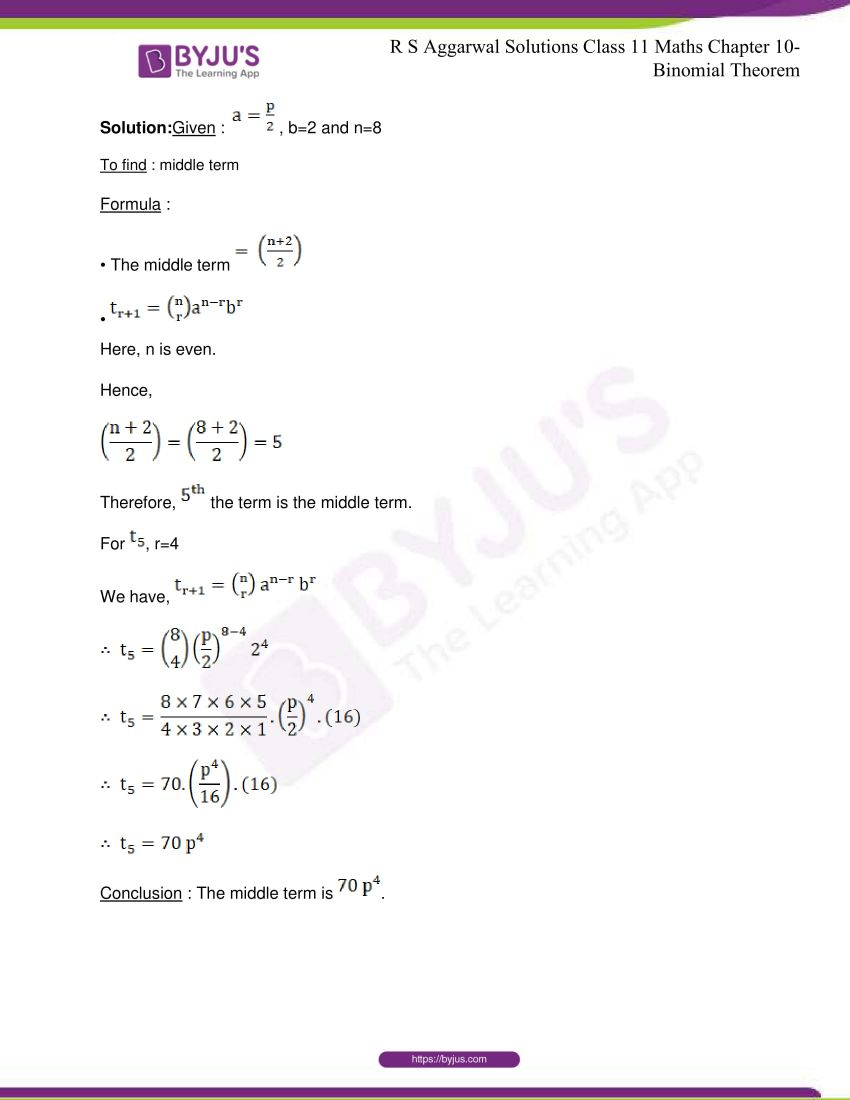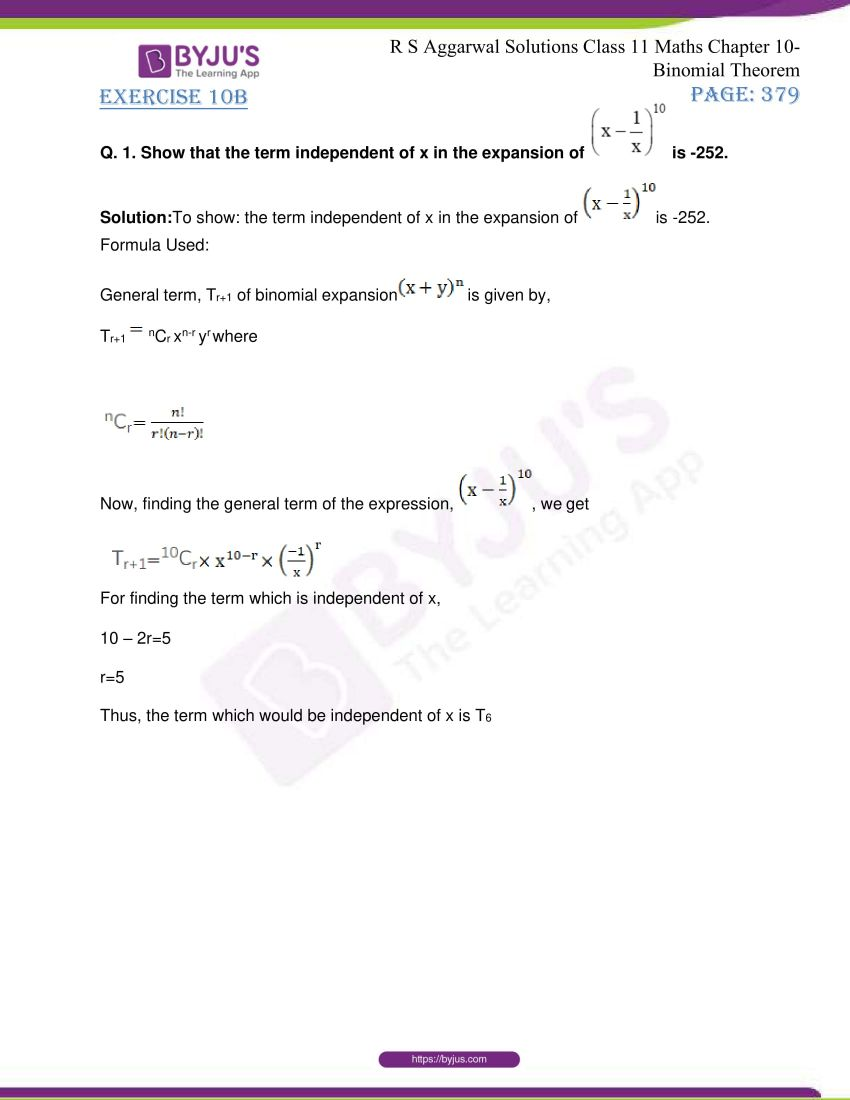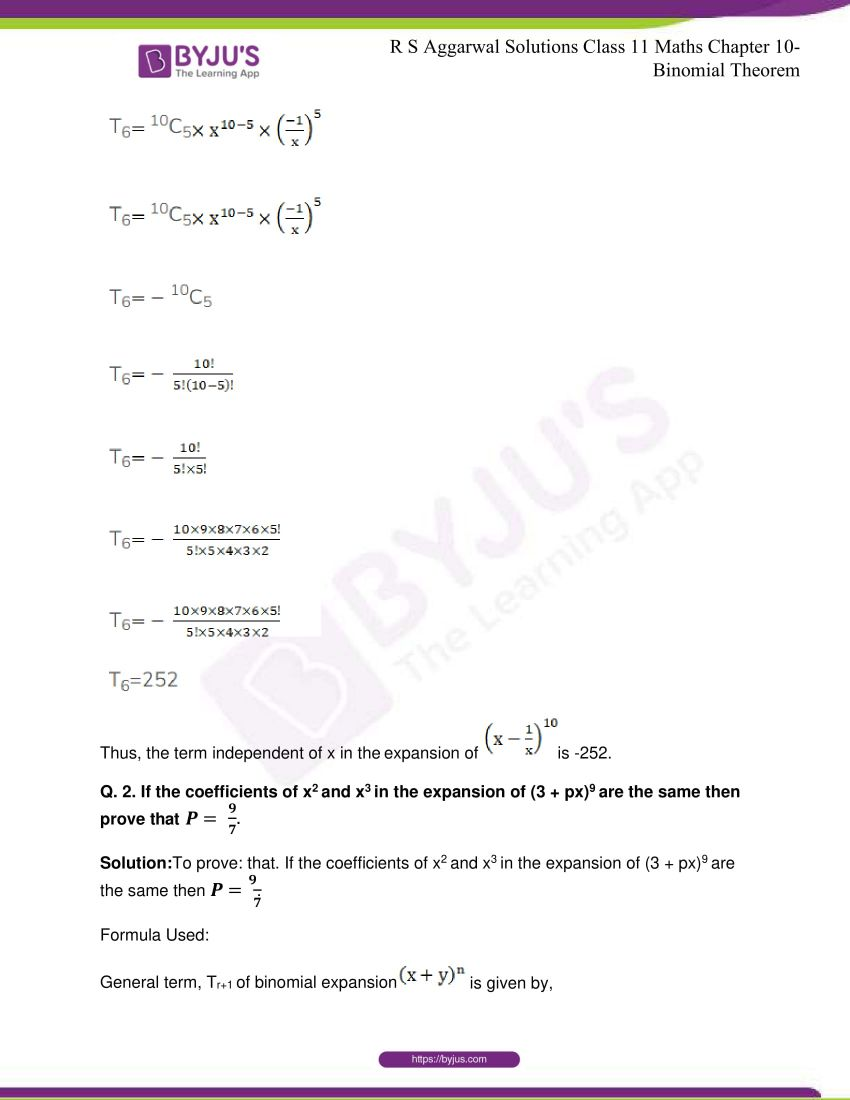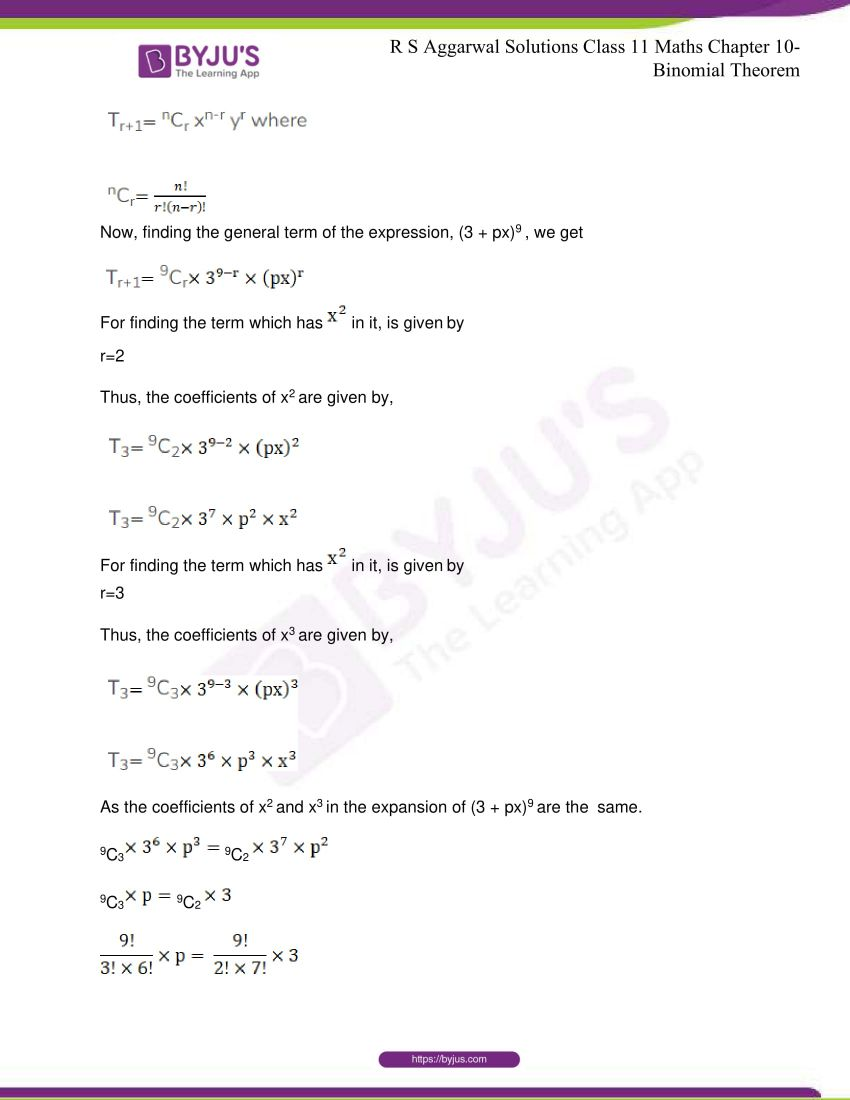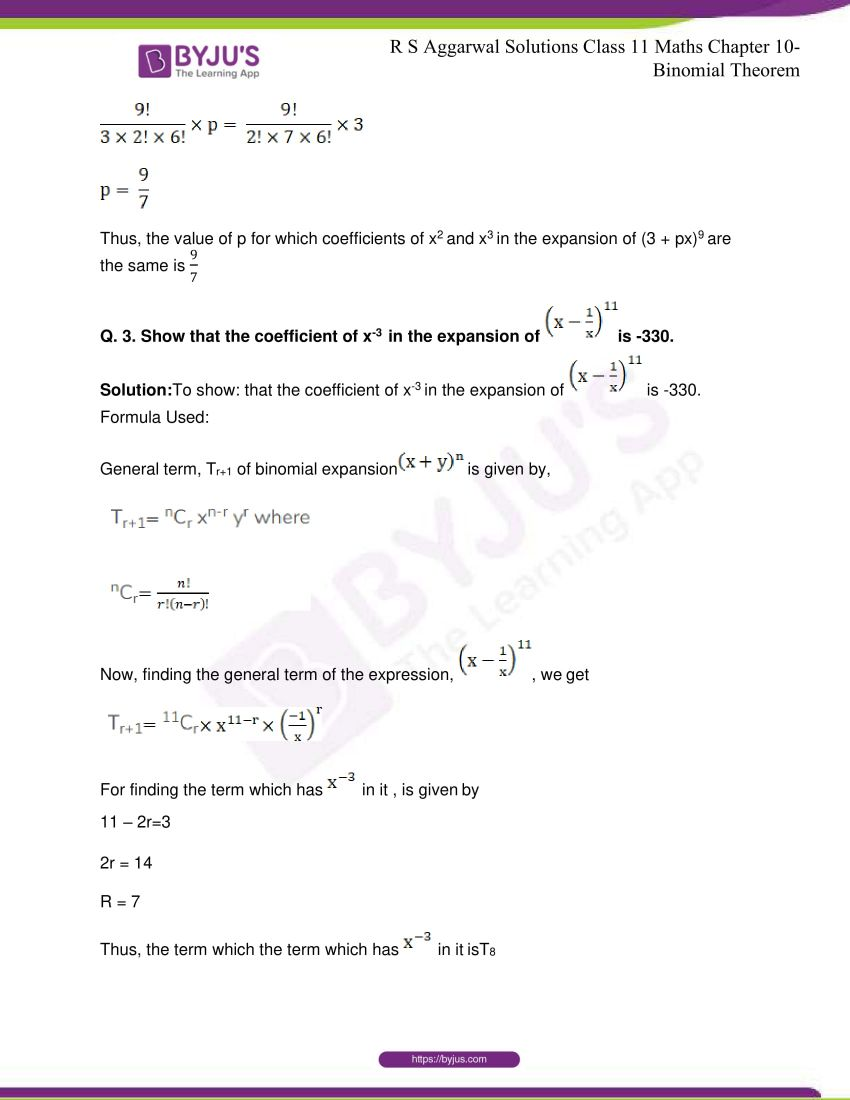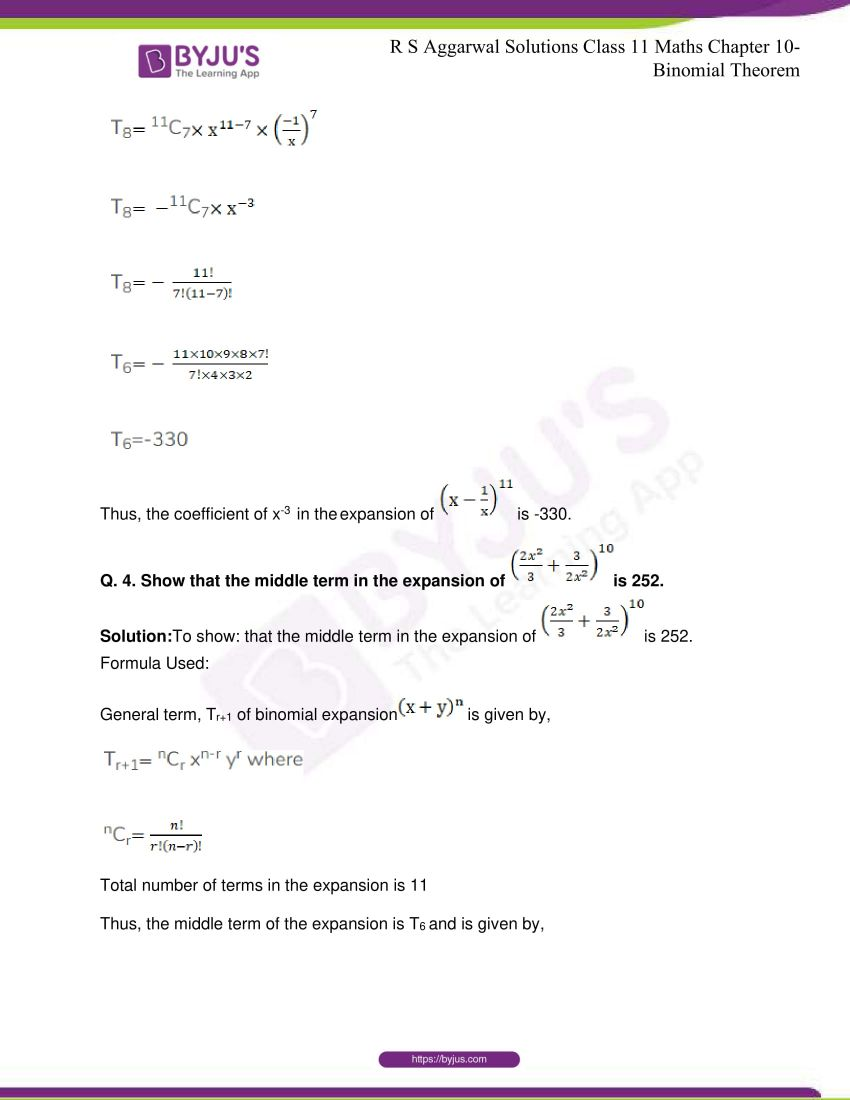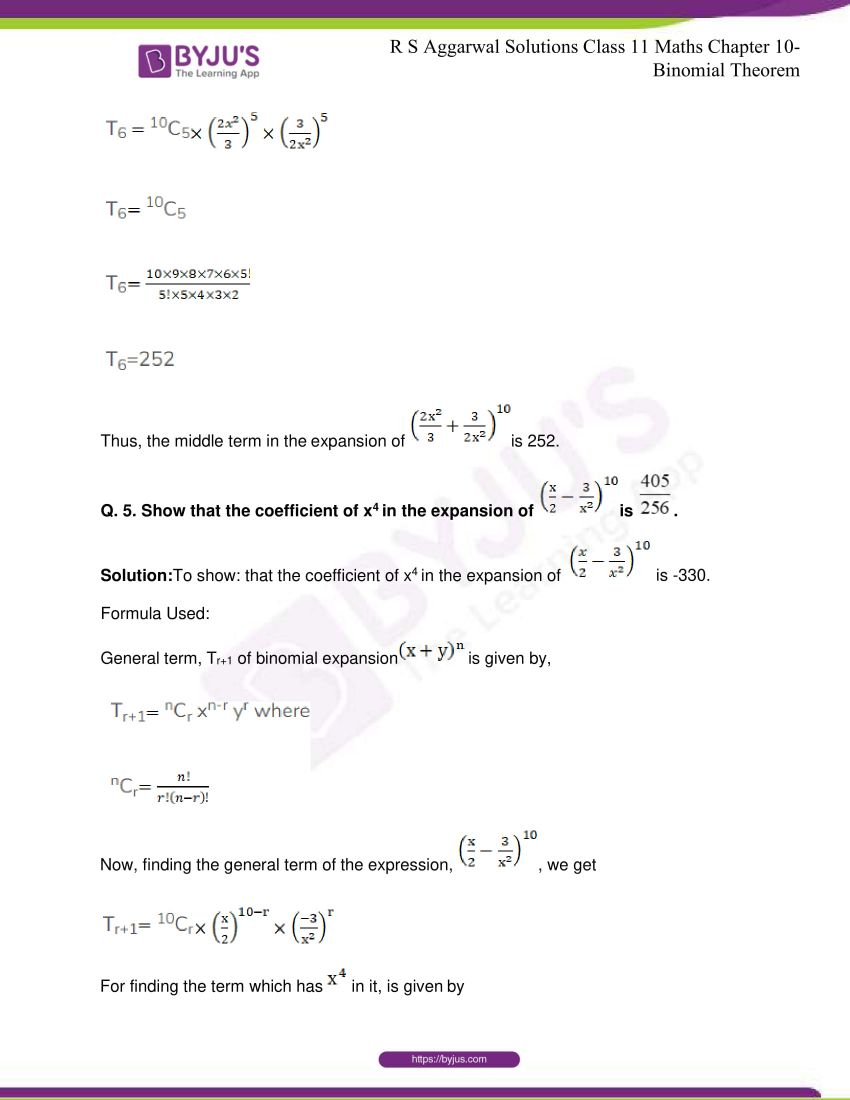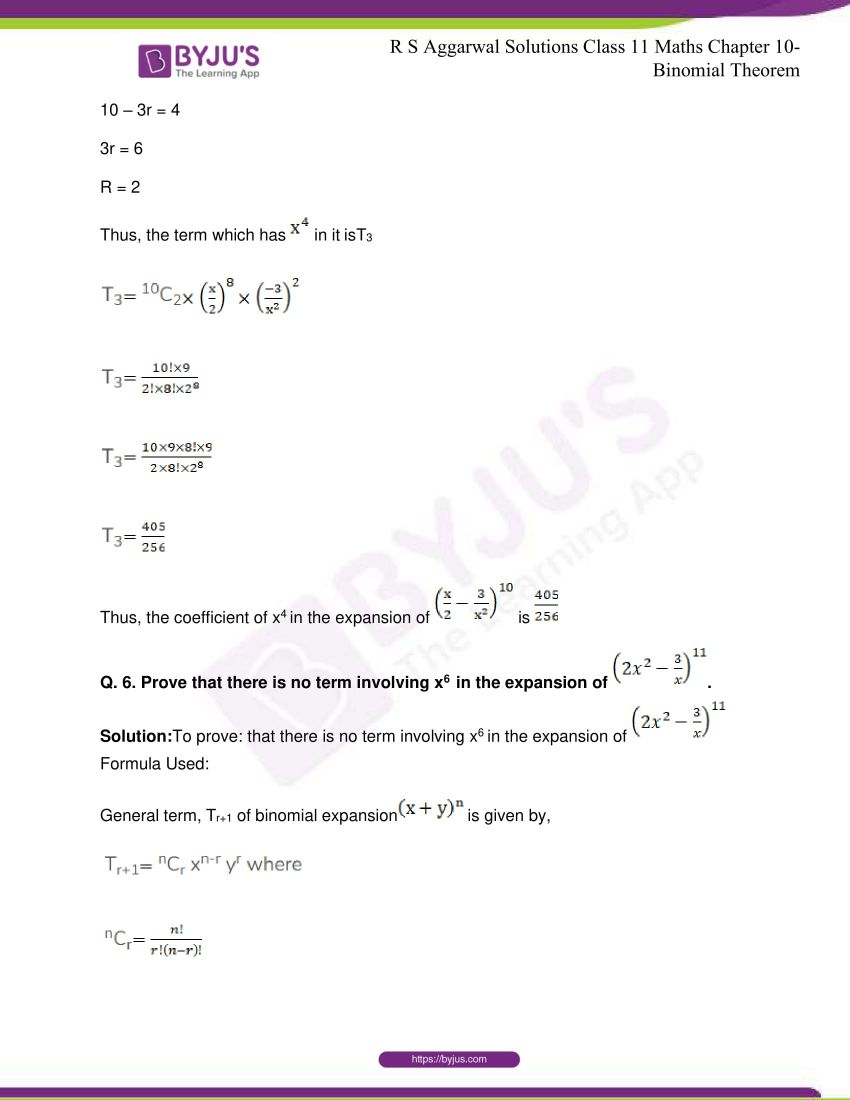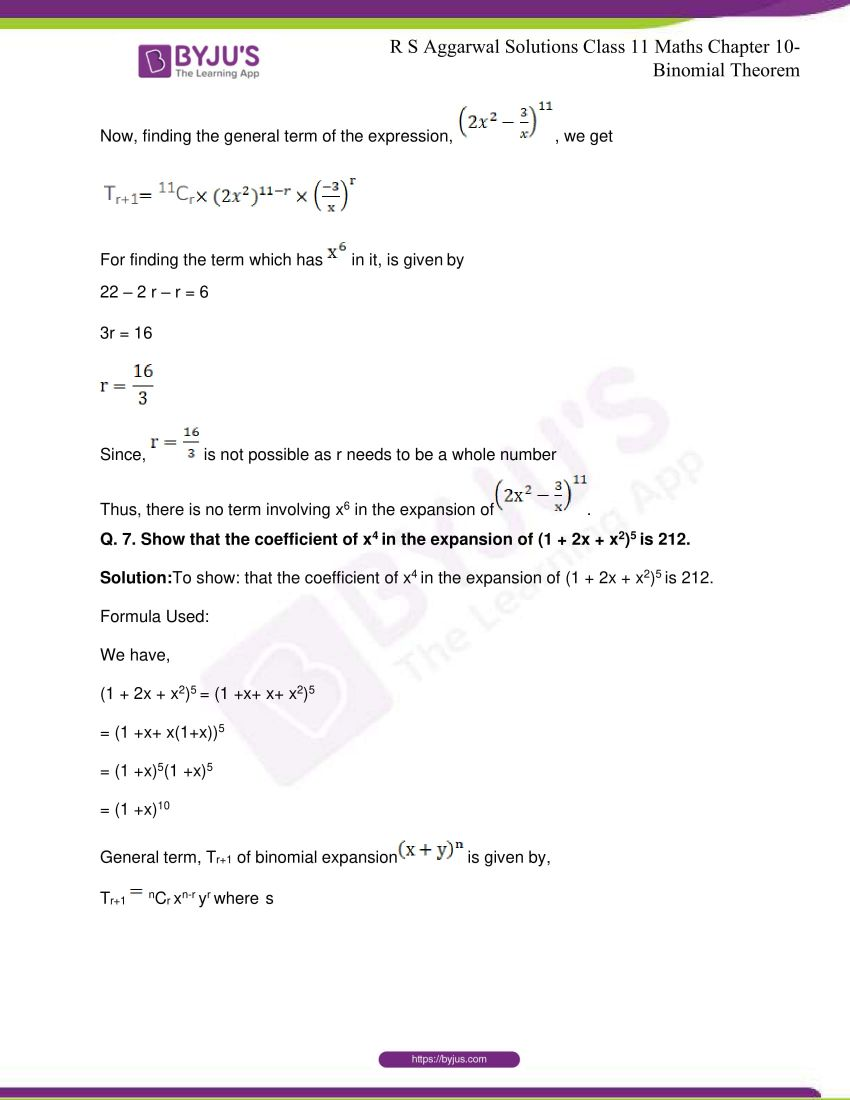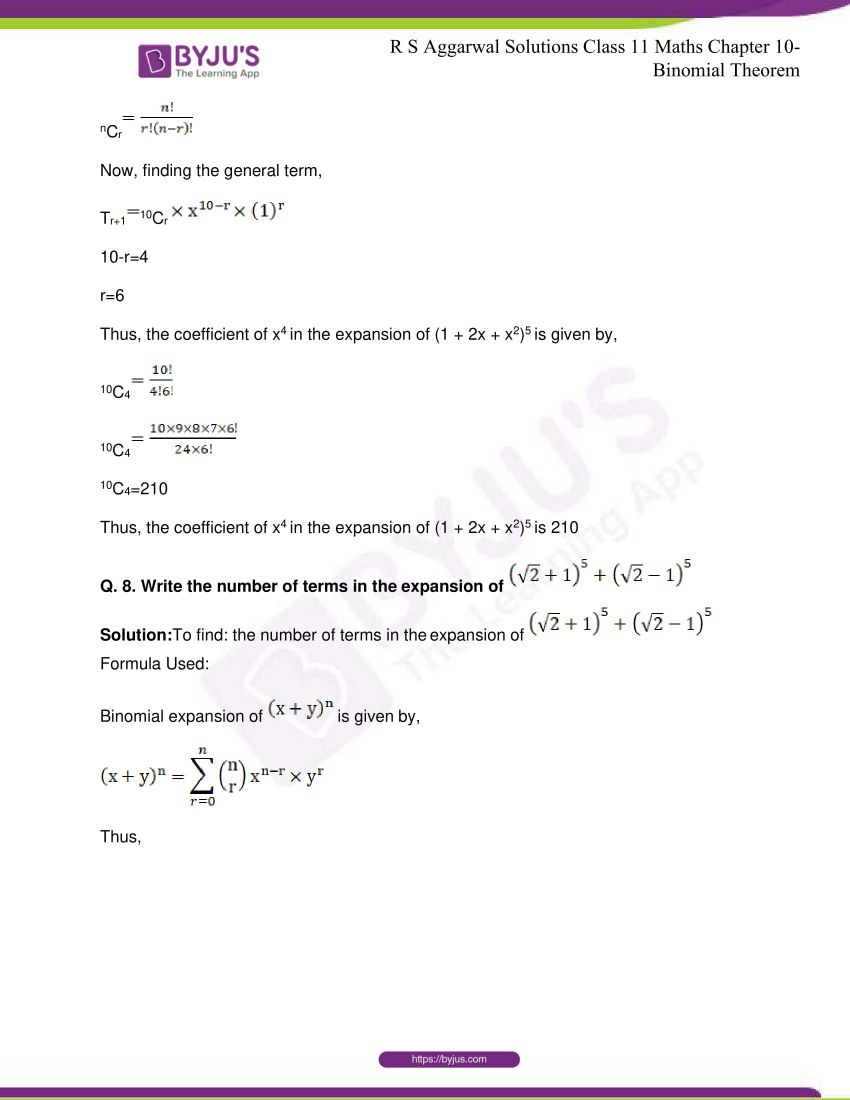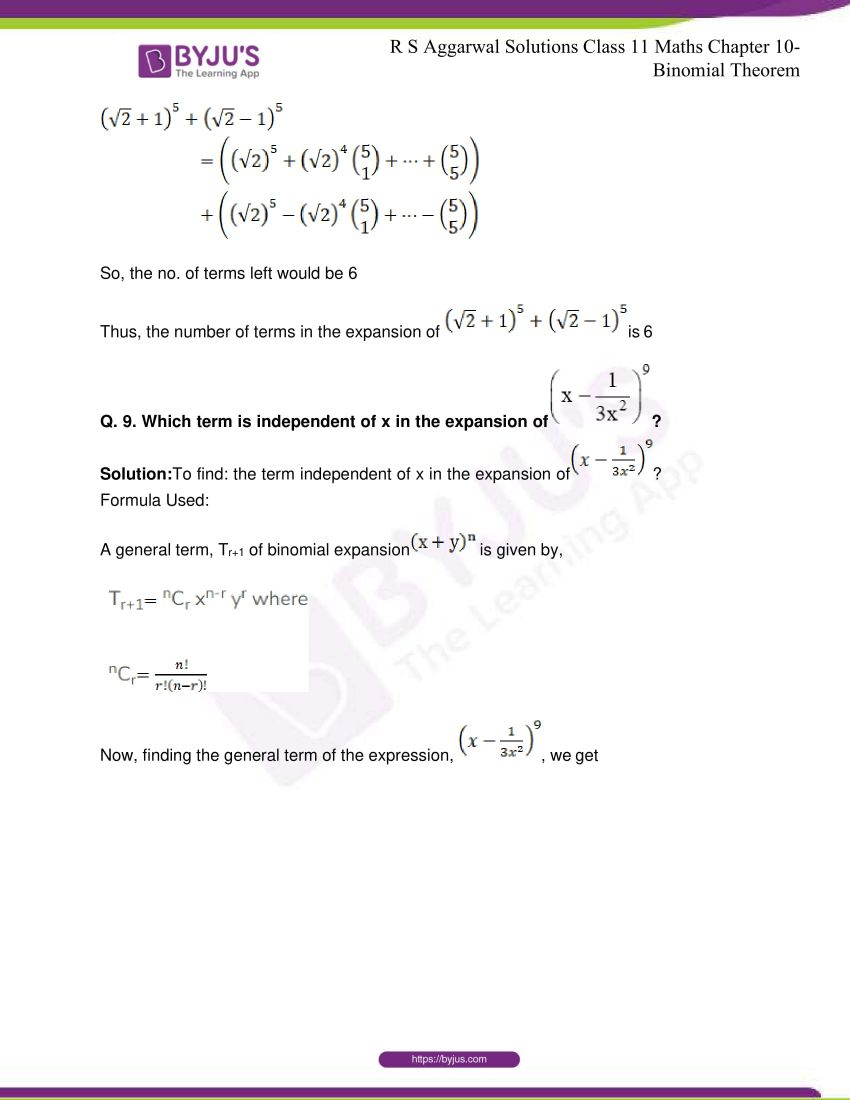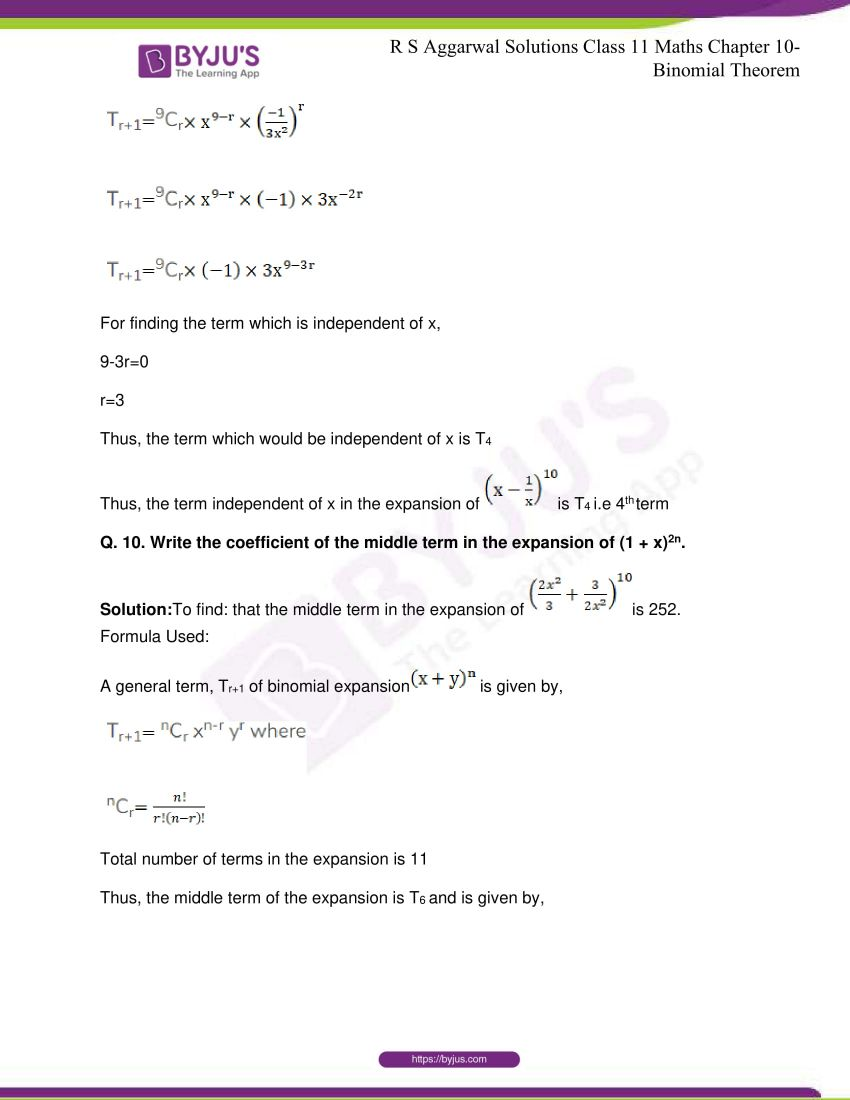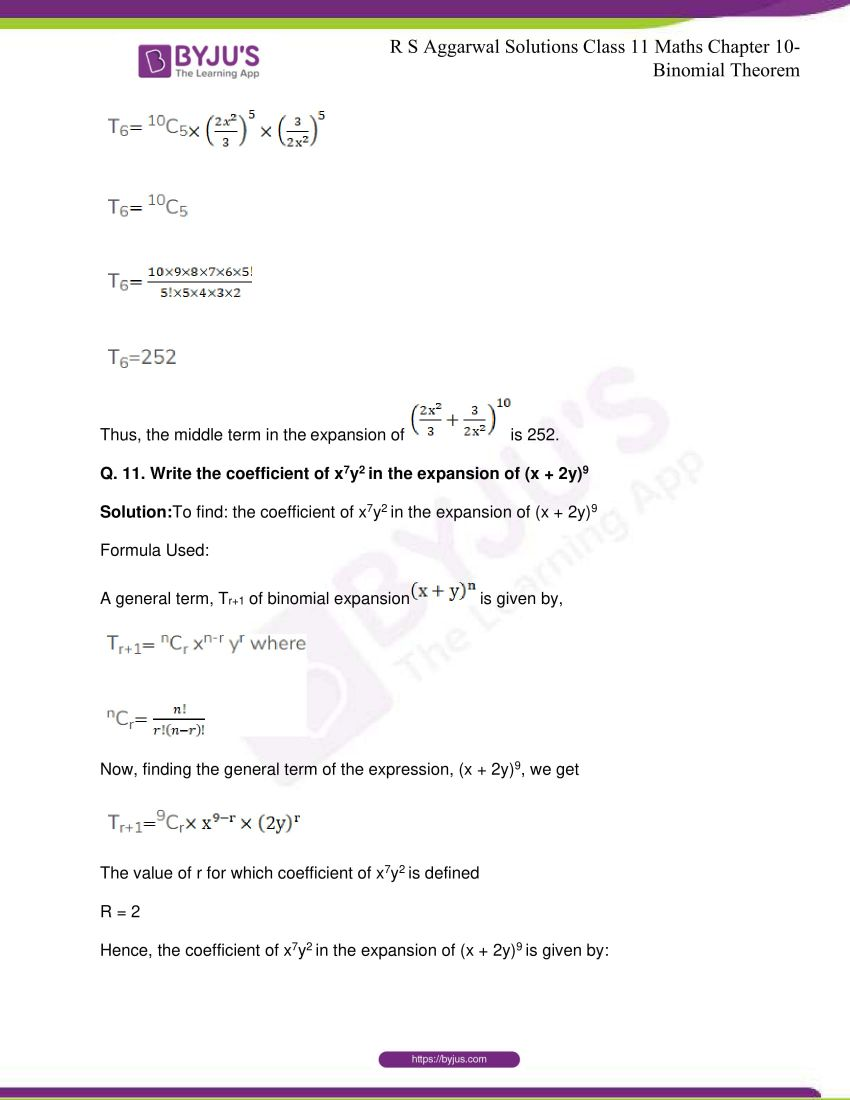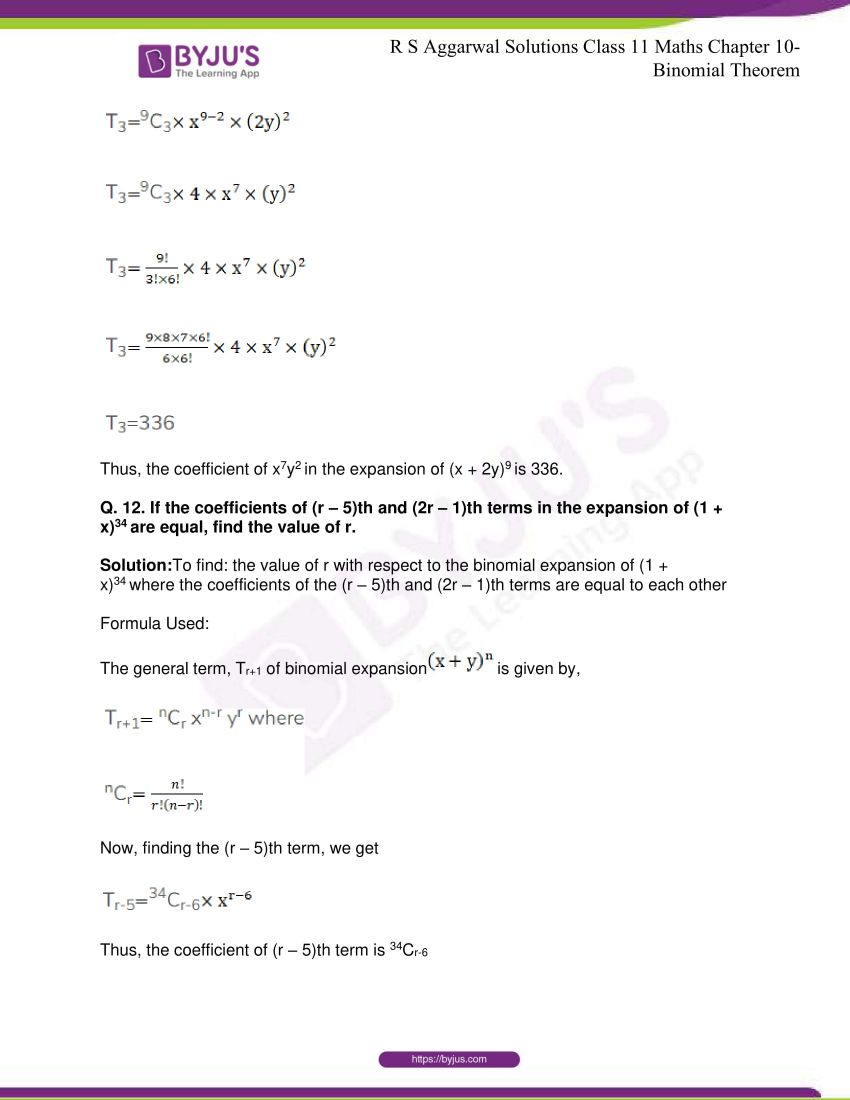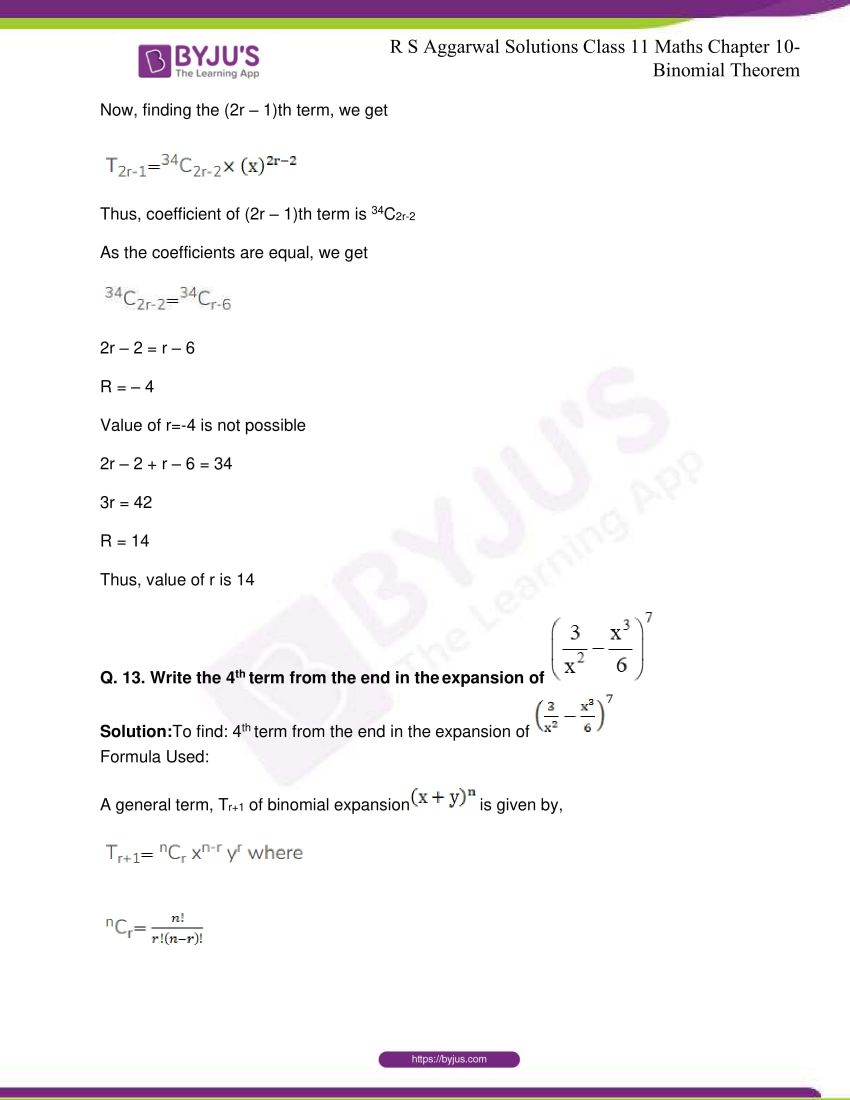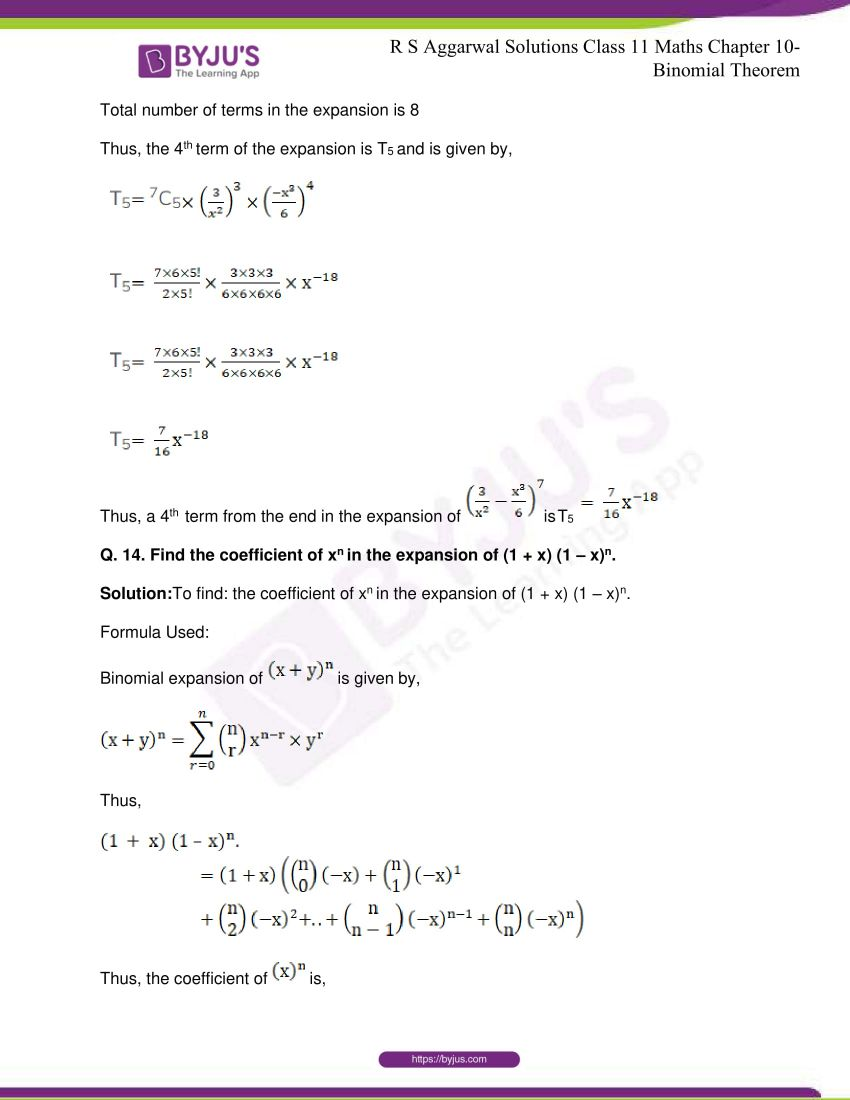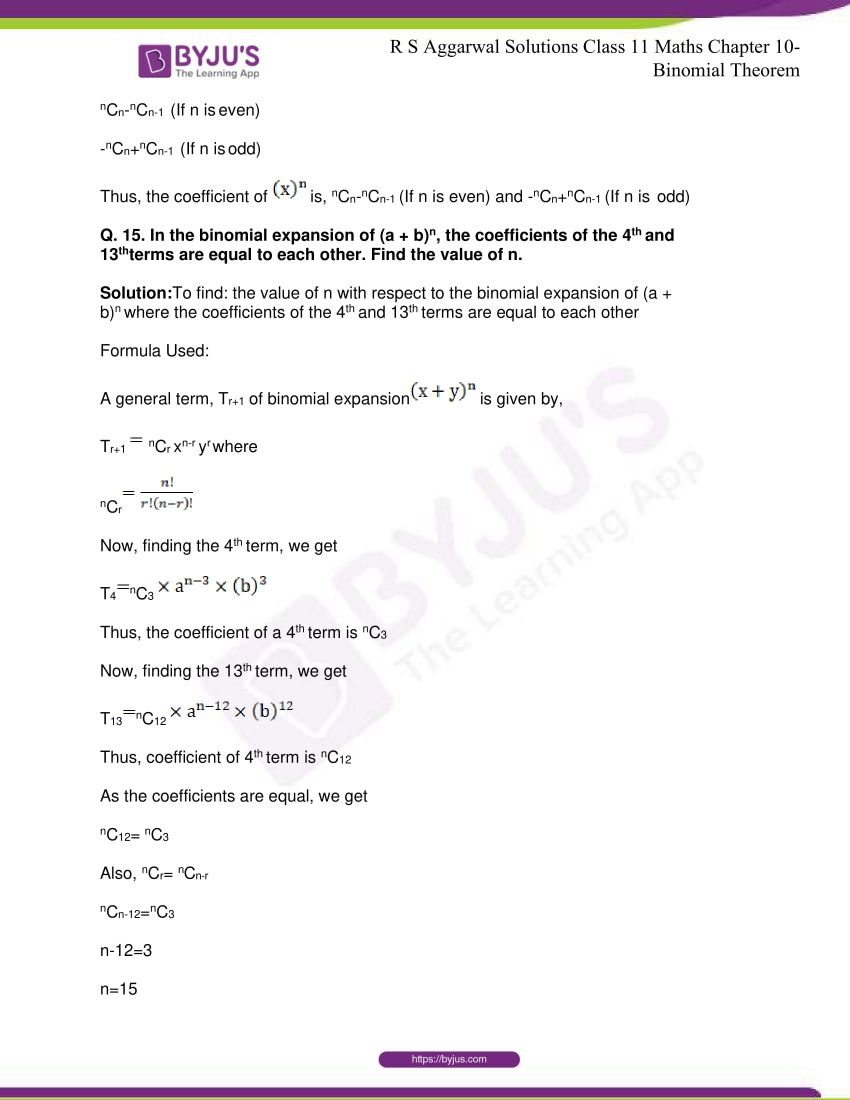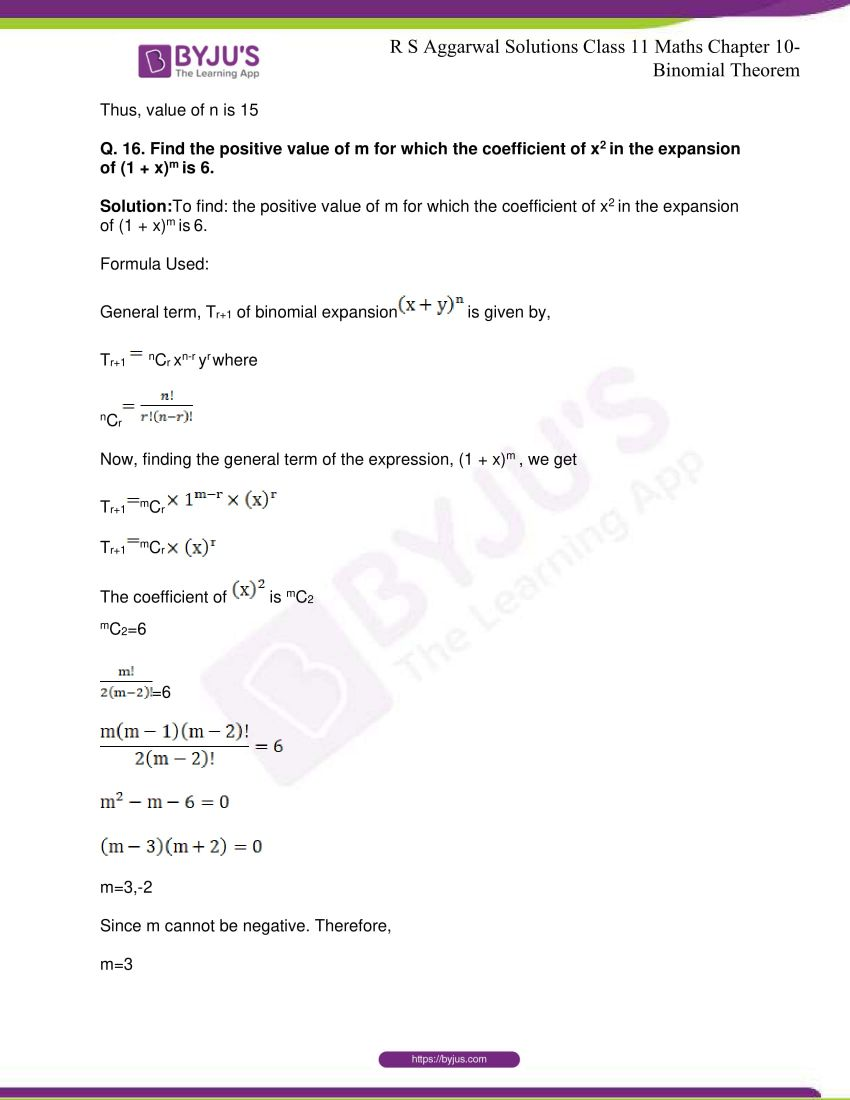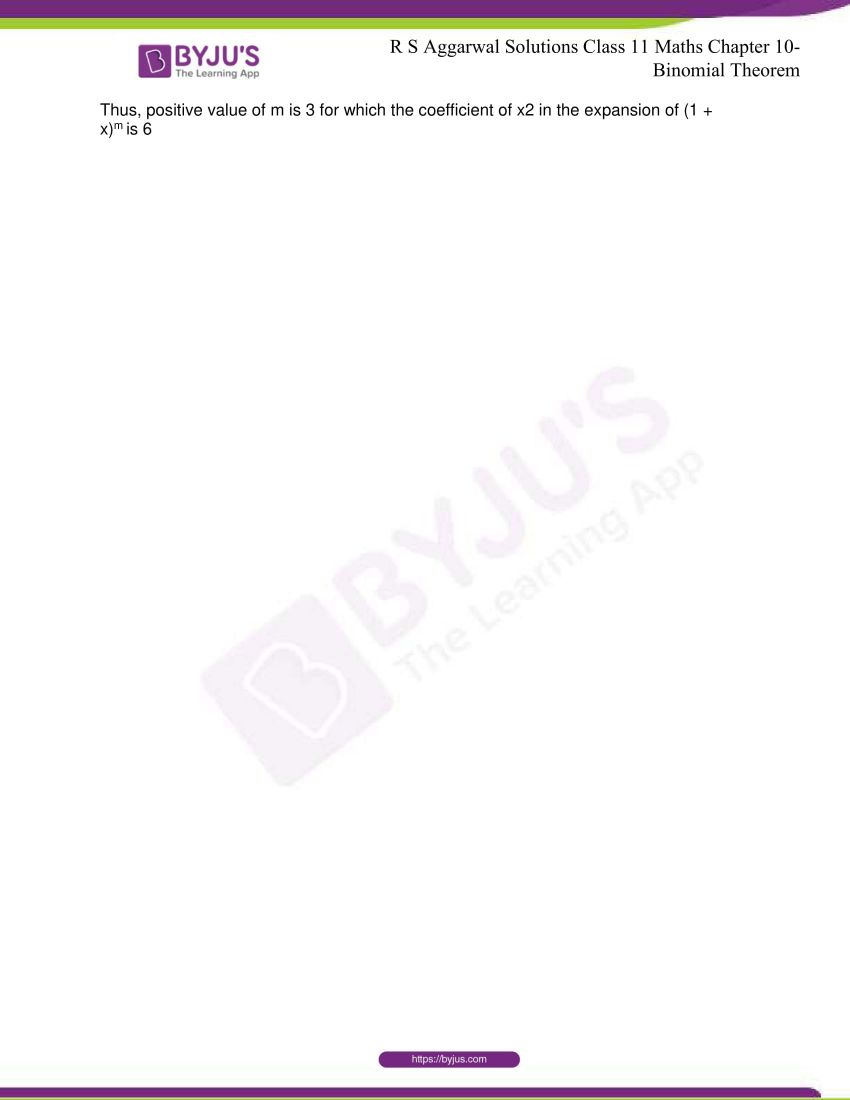## R S Aggarwal Solutions for Class 11 Maths Chapter 10- Binomial Theorem

Chapter 10, Binomial Theorem, consists of 2 exercises and the solutions given here contain answers to all the questions present in these exercises. Let us have a look at some of the topics that are being discussed in this chapter.

10.1 Binomial Theorem

10.2 Binomial Expansion

10.3 Deduction

## R S Aggarwal Solutions for Class 11 Maths Chapter 10 – Binomial Theorem

A binomial expression that has been raised to a very large power can be easily calculated with the help of Binomial Theorem. The details about binomial theorem like its definition, properties, applications, etc are discussed in this chapter. Here, in this chapter, students are also taught to solve problems dealing with Binomial Theorem. Read and learn Chapter 10 of R S Aggarwal textbook to get to know more about Binomial Theorem. Practice the R S Aggarwal Solutions for Class 11 effectively to attain excellent results in the examination.